C语言系统学习 | 风尘孤狼
0%

C语言系统学习

## 高级语言

10111000 00000001 00000000 00000101 00000001 00000000

MOV AX, 1 ADD AX, 1

1 + 1

## 什么是C语言?

C语言是一种用于和计算机交流的高级语言, 它既具有高级语言的特点，又具有汇编语言的特点

C语言是所有编程语言中的经典，很多高级语言都是从C语言中衍生出来的，

C语言是所有编程语言中的经典，很多著名的系统软件也是C语言编写的

## 函数定义格式

• 主函数定义的格式:
• int 代表函数执行之后会返回一个整数类型的值
• main 代表这个函数的名字叫做main
• () 代表这是一个函数
• {} 代表这个程序段的范围
• return 0; 代表函数执行完之后返回整数0
``````int main(void) {
// insert code here...
return 0;
}``````
• 其它函数定义的格式
• int 代表函数执行之后会返回一个整数类型的值
• call 代表这个函数的名字叫做call
• () 代表这是一个函数
• {} 代表这个程序段的范围
• return 0; 代表函数执行完之后返回整数0
``````int call(void) {
return 0;
}``````

## 如何执行定义好的函数

call 代表找到名称叫做call的某个东西
() 代表要找到的名称叫call的某个东西是一个函数
; 代表调用函数的语句已经编写完成

``````int main(void) {
call();
return 0;
}``````

1.输出内容是一个比较复杂的操作, 所以系统提前定义好了一个专门用于输出内容的函数叫做printf函数,我们只需要执行系统定义好的printf函数就可以往屏幕上输出内容
2.但凡需要执行一个函数, 都是通过函数名称+圆括号的形式来执行
3.如下代码的含义是: 当程序运行时系统会自动执行main函数, 在系统自动执行main函数时我们手动执行了call函数和printf函数
4.经过对代码的观察, 我们发现两个问题

``````int call(void){
return 0;
}

int main(void){
call();
printf();
return 0;
}``````
• 如何告诉printf函数要输出的内容
• 将要输出的内容编写到printf函数后面的圆括号中即可
• 注意: 圆括号中编写的内容必须用双引号引起来
``printf("hello world\n");``
• 如何找到printf函数的实现代码
• 由于printf函数是系统实现的函数, 所以想要使用printf函数必须在使用之前告诉系统去哪里可以找到printf函数的实现代码
• #include <stdio.h> 就是告诉系统可以去stdio这个文件中查找printf函数的声明和实现
``````#include <stdio.h>

int call(void){
return 0;
}

int main(void){
call();
printf("hello world\n");
return 0;
}``````

## main函数注意点及其它写法

• C语言中,每条完整的语句后面都必须以分号结尾
``````int main(void){
printf("hello world\n") // 如果没有分号编译时会报错
return 0;
}
``````
``````int main(void){
// 如果没有分号,多条语句合并到一行时, 系统不知道从什么地方到什么地方是一条完整语句
printf("hello world\n") return 0;
}
``````
• C语言中除了注释和双引号引起来的地方以外都不能出现中文
``````int main(void){
printf("hello world\n"); // 这里的分号如果是中文的分号就会报错
return 0;
}
``````
• 一个C语言程序只能有一个main函数
``````int main(void){
return 0;
}
int main(void){ // 编译时会报错, 重复定义
return 0;
}``````
• 一个C语言程序不能没有main函数
``````int call(){ // 编译时报错, 因为只有call函数, 没有main函数
return 0;
}``````
``````int mian(){ // 编译时报错, 因为main函数的名称写错了,还是相当于没有main函数
return 0;
}``````
• main函数前面的int可以不写或者换成void（建议不要换，只是有时候你换了可能也对，但是很多编译器市不支持的，老老实实用最标准的格式是最好不过的：`int main(void)`,其次这个void最好养成加上的习惯，避免报错的风险）
``````#include <stdio.h>
int main(void){ // 最标准的形式，前面的int不加也不会报错
printf("hello world\n");
return 0;
}``````
• main函数中的return 0可以不写
• return 语句（返回语句）是程序的最后一个语句。在 int main（void）中 int 表示 main（）函数的返回值应该是一个整数。C标准要求 main（）这样做。带有返回值的 C语言函数要使用一个 return 语句，该语句包括关键字 return，后面紧跟着要返回的值，然后是一个分号。对于 main（）函数来说，如果你漏掉了 return语句，则大多数编译器将对你的疏忽提出警告，但仍将编译该程序。此时，你可以暂时把 main（）中的 return语句看作是保持逻辑连贯性所需的内容。但对于某些操作系统（包括 DOS 和 UNIX ）而言，它有实际的用途。
``````int main(){ // 不会报错
printf("hello world\n");
}``````
• 多种写法不报错的原因
• C语言最早的时候只是一种规范和标准(例如C89, C11等)
• 标准的推行需要各大厂商的支持和实施
• 而在支持的实施的时候由于各大厂商利益、理解等问题,导致了实施的标准不同,发生了变化
• Turbo C
• Visual C(VC)
• GNU C(GCC)
• 所以大家才会看到不同的书上书写的格式有所不同, 有的返回int,有的返回void,有的甚至没有返回值
• 所以大家只需要记住最标准的写法即可
``````#include <stdio.h>
int main(void){
printf("hello world\n");
return 0;
}``````

`Tips:`
`语法错误：编译器会直接报错`
`逻辑错误：没有语法错误，只不过运行结果不正确`

## C语言程序练习

• 编写一个C语言程序,用至少2种方式在屏幕上输出以下内容
`````` *** ***
*********
*******
****
**
``````
• 普通青年实现
``````printf(" *** *** \n");
printf("*********\n");
printf(" *******\n");
printf("  ****\n");
printf("   **\n");
``````
• 2B青年实现
``````printf(" *** *** \n*********\n *******\n  ****\n   **\n");
``````
• 文艺青年实现(纯属装b)
``````int  i = 0;
while (1) {
if (i % 2 == 0) {
printf(" *** *** \n");
printf("*********\n");
printf(" *******\n");
printf("  ****\n");
printf("   **\n");
}else
{
printf("\n");
printf("   ** ** \n");
printf("  *******\n");
printf("   *****\n");
printf("    **\n");
}
sleep(1);
i++;
system("cls");
}``````

## 初学者如何避免程序出现BUG

``````                   _ooOoo_
o8888888o
88" . "88
(| -_- |)
O\ = /O
____/`---'\____
.   ' \\| |// `.
/ \\||| : |||// \
/ _||||| -:- |||||- \
| | \\\ - /// | |
| \_| ''\---/'' | |
\ .-\__ `-` ___/-. /
___`. .' /--.--\ `. . __
."" '< `.___\_<|>_/___.' >'"".
| | : `- \`.;`\ _ /`;.`/ - ` : | |
\ \ `-. \_ __\ /__ _/ .-` / /
======`-.____`-.___\_____/___.-`____.-'======
`=---='

.............................................
佛祖保佑                   有无BUG
``````
``````━━━━━━神兽出没━━━━━━
┏┓　　　 ┏┓
┏┛┻━━━━━━┛┻┓
┃　　　     ┃
┃　　　━    ┃
┃　┳┛　  ┗┳ ┃
┃　　　     ┃
┃　　　┻    ┃
┃          ┃
┗━┓　　　 ┏━┛Code is far away from bug with the animal protecting
┃　　 　┃    神兽保佑,代码无bug
┃　　 　┃
┃　　　 ┗━━━┓
┃　　　     ┣┓
┃　　　  ┏━━┛┛
┗┓┓┏━┳┓┏┛
┃┫┫ ┃┫┫
┗┻┛ ┗┻┛

━━━━━━感觉萌萌哒━━━━━━
``````

## 多语言对比

• C语言
``````#include<stdio.h>
int main(void) {
printf("好好学习");
return 0;
}
``````
• C++语言
``````#include<iostream>
using namespace std;
int main() {
cout << "好好学习" << endl;
return 0;
}
``````
• OC语言
``````#import <Foundation/Foundation.h>
int main() {
NSLog(@"好好学习");
return 0;
}
``````
• Java语言
``````class Test
{
public static viod main()
{
system.out.println("好好学习");
}
}
``````
• Go语言
``````package main
import  "fmt" //引入fmt库
func main() {
fmt.Println("好好学习")
}
``````

## 什么是注释?

• 注释是在所有计算机语言中都非常重要的一个概念，从字面上看，就是注解、解释的意思
• 注释可以用来解释某一段程序或者某一行代码是什么意思，方便程序员之间的交流沟通
• 注释可以是任何文字，也就是说可以写中文
• 被注释的内容在开发工具中会有特殊的颜色

## 为什么要使用注释?

• 没有编写任何注释的程序
``````void printMap(char map , int row, int col);
int main(int argc, const char * argv[])
{
char map = {
{'#', '#', '#', '#', '#', '#', '#'},
{'#', ' ', ' ', ' ', '#' ,' ', ' '},
{'#', 'R', ' ', '#', '#', ' ', '#'},
{'#', ' ', ' ', ' ', '#', ' ', '#'},
{'#', '#', ' ', ' ', ' ', ' ', '#'},
{'#', '#', '#', '#', '#', '#', '#'}
};
int row = sizeof(map)/sizeof(map);
int col = sizeof(map)/ sizeof(map);
printMap(map, row, col);
int pRow = 2;
int pCol = 1;
int endRow = 1;
int endCol = 6;
while ('R' != map[endRow][endCol]) {
printf("亲, 请输入相应的操作\n");
printf("w(向上走) s(向下走) a(向左走) d(向右走)\n");
char run;
run = getchar();
switch (run) {
case 's':
if ('#' != map[pRow + 1][pCol]) {
map[pRow][pCol] = ' ';
pRow++;//3
map[pRow][pCol] = 'R';
}
break;
case 'w':
if ('#' != map[pRow - 1][pCol]) {
map[pRow][pCol] = ' ';
pRow--;
map[pRow][pCol] = 'R';
}
break;
case 'a':
if ('#' != map[pRow][pCol - 1]) {
map[pRow][pCol] = ' ';
pCol--;
map[pRow][pCol] = 'R';
}
break;
case 'd':
if ('#' != map[pRow][pCol + 1]) {
map[pRow][pCol] = ' ';
pCol++;
map[pRow][pCol] = 'R';
}
break;
}
printMap(map, row, col);
}
printf("你太牛X了\n");
printf("想挑战自己,请购买完整版本\n");
return 0;
}
void printMap(char map , int row, int col)
{
system("cls");
for (int i = 0; i < row; i++) {
for (int j = 0; j < col; j++) {
printf("%c", map[i][j]);
}
printf("\n");
}
}
``````
• 编写了注释的程序
``````/*
R代表一个人
#代表一堵墙
//   0123456
####### // 0
#   #   // 1
#R ## # // 2
#   # # // 3
##    # // 4
####### // 5

分析:
>1.保存地图(二维数组)
>2.输出地图
>3.操作R前进(控制小人行走)
3.1.接收用户输入(scanf/getchar)
w(向上走) s(向下走) a(向左走) d(向右走)
3.2.判断用户的输入,控制小人行走
3.2.1.替换二维数组中保存的数据
(
1.判断是否可以修改(如果不是#就可以修改)
2.修改现有位置为空白
3.修改下一步为R
)
3.3.输出修改后的二维数组
4.判断用户是否走出出口
*/
// 声明打印地图方法
void printMap(char map , int row, int col);
int main(int argc, const char * argv[])
{
// 1.定义二维数组保存迷宫地图
char map = {
{'#', '#', '#', '#', '#', '#', '#'},
{'#', ' ', ' ', ' ', '#' ,' ', ' '},
{'#', 'R', ' ', '#', '#', ' ', '#'},
{'#', ' ', ' ', ' ', '#', ' ', '#'},
{'#', '#', ' ', ' ', ' ', ' ', '#'},
{'#', '#', '#', '#', '#', '#', '#'}
};
// 2.计算地图行数和列数
int row = sizeof(map)/sizeof(map);
int col = sizeof(map)/ sizeof(map);
// 3.输出地图
printMap(map, row, col);
// 4.定义变量记录人物位置
int pRow = 2;
int pCol = 1;
// 5.定义变量记录出口的位置
int endRow = 1;
int endCol = 6;
// 6.控制人物行走
while ('R' != map[endRow][endCol]) {
// 6.1提示用户如何控制人物行走
printf("亲, 请输入相应的操作\n");
printf("w(向上走) s(向下走) a(向左走) d(向右走)\n");
char run;
run = getchar();
// 6.2根据用户输入控制人物行走
switch (run) {
case 's':
if ('#' != map[pRow + 1][pCol]) {
map[pRow][pCol] = ' ';
pRow++;//3
map[pRow][pCol] = 'R';
}
break;
case 'w':
if ('#' != map[pRow - 1][pCol]) {
map[pRow][pCol] = ' ';
pRow--;
map[pRow][pCol] = 'R';
}
break;
case 'a':
if ('#' != map[pRow][pCol - 1]) {
map[pRow][pCol] = ' ';
pCol--;
map[pRow][pCol] = 'R';
}
break;
case 'd':
if ('#' != map[pRow][pCol + 1]) {
map[pRow][pCol] = ' ';
pCol++;
map[pRow][pCol] = 'R';
}
break;
}
// 6.3重新输出行走之后的地图
printMap(map, row, col);
}
printf("你太牛X了\n");
printf("想挑战自己,请购买完整版本\n");
return 0;
}

/**
* @brief printMap
* @param map 需要打印的二维数组
* @param row 二维数组的行数
* @param col 二维数组的列数
*/
void printMap(char map , int row, int col)
{
// 为了保证窗口的干净整洁, 每次打印都先清空上一次的打印
system("cls");
for (int i = 0; i < row; i++) {
for (int j = 0; j < col; j++) {
printf("%c", map[i][j]);
}
printf("\n");
}
}
``````

## 注释的分类

• 单行注释

• // 被注释内容
• 使用范围:任何地方都可以写注释：函数外面、里面，每一条语句后面
• 作用范围: 从第二个斜线到这一行末尾
• 快捷键:Ctrl+/
• 多行注释

• /* 被注释内容 */
• 使用范围:任何地方都可以写注释：函数外面、里面，每一条语句后面
• 作用范围: 从第一个/到最近的一个/

## 注释的注意点

• 单行注释可以嵌套单行注释、多行注释
``````// 好好学习 // hzy2003628.top
// /* 进我的博客好好学习 */
// 坚持
``````
• 多行注释可以嵌套单行注释
``````/*
// 作者：HZY
// 描述：第一个C语言程序作用：这是一个主函数，C程序的入口点
*/
``````
• 多行注释不能嵌套多行注释
``````/*

/*玩*/
睡觉
*/
``````

## 注释的应用场景

• 思路分析
``````/*
R代表一个人
#代表一堵墙
//   0123456
####### // 0
#   #   // 1
#R ## # // 2
#   # # // 3
##    # // 4
####### // 5

分析:
>1.保存地图(二维数组)
>2.输出地图
>3.操作R前进(控制小人行走)
3.1.接收用户输入(scanf/getchar)
w(向上走) s(向下走) a(向左走) d(向右走)
3.2.判断用户的输入,控制小人行走
3.2.1.替换二维数组中保存的数据
(
1.判断是否可以修改(如果不是#就可以修改)
2.修改现有位置为空白
3.修改下一步为R
)
3.3.输出修改后的二维数组
4.判断用户是否走出出口
*/
``````
• 对变量进行说明
``````// 2.计算地图行数和列数
int row = sizeof(map)/sizeof(map);
int col = sizeof(map)/ sizeof(map);
``````
• 对函数进行说明
``````/**
* @brief printMap
* @param map 需要打印的二维数组
* @param row 二维数组的行数
* @param col 二维数组的列数
*/
void printMap(char map , int row, int col)
{
system("cls");
for (int i = 0; i < row; i++) {
for (int j = 0; j < col; j++) {
printf("%c", map[i][j]);
}
printf("\n");
}
}
``````
• 多实现逻辑排序
``````// 1.定义二维数组保存迷宫地图
char map = {
{'#', '#', '#', '#', '#', '#', '#'},
{'#', ' ', ' ', ' ', '#' ,' ', ' '},
{'#', 'R', ' ', '#', '#', ' ', '#'},
{'#', ' ', ' ', ' ', '#', ' ', '#'},
{'#', '#', ' ', ' ', ' ', ' ', '#'},
{'#', '#', '#', '#', '#', '#', '#'}
};
// 2.计算地图行数和列数
int row = sizeof(map)/sizeof(map);
int col = sizeof(map)/ sizeof(map);
// 3.输出地图
printMap(map, row, col);
// 4.定义变量记录人物位置
int pRow = 2;
int pCol = 1;
// 5.定义变量记录出口的位置
int endRow = 1;
int endCol = 6;
// 6.控制人物行走
while ('R' != map[endRow][endCol]) {
... ...
}
``````

## 使用注释的好处

• 注释是一个程序员必须要具备的良好习惯
• 帮助开发人员整理实现思路
• 解释说明程序, 提高程序的可读性
• 初学者编写程序可以养成习惯：先写注释再写代码
• 将自己的思想通过注释先整理出来，在用代码去体现
• 因为代码仅仅是思想的一种体现形式而已

## 什么是关键字?

• 关键字,也叫作保留字。是指一些被C语言赋予了特殊含义的单词
• 关键字特征:
• 全部都是小写
• 在开发工具中会显示特殊颜色
• 关键字注意点:
• 因为关键字在C语言中有特殊的含义, 所以不能用作变量名、函数名等
• C语言中一共有32个关键字
1 2 3 4 5 6 7 8
char short int long float double if else
return do while for switch case break continue
default goto sizeof auto register static extern unsigned
signed typedef struct enum union void const volatile

## 关键字分类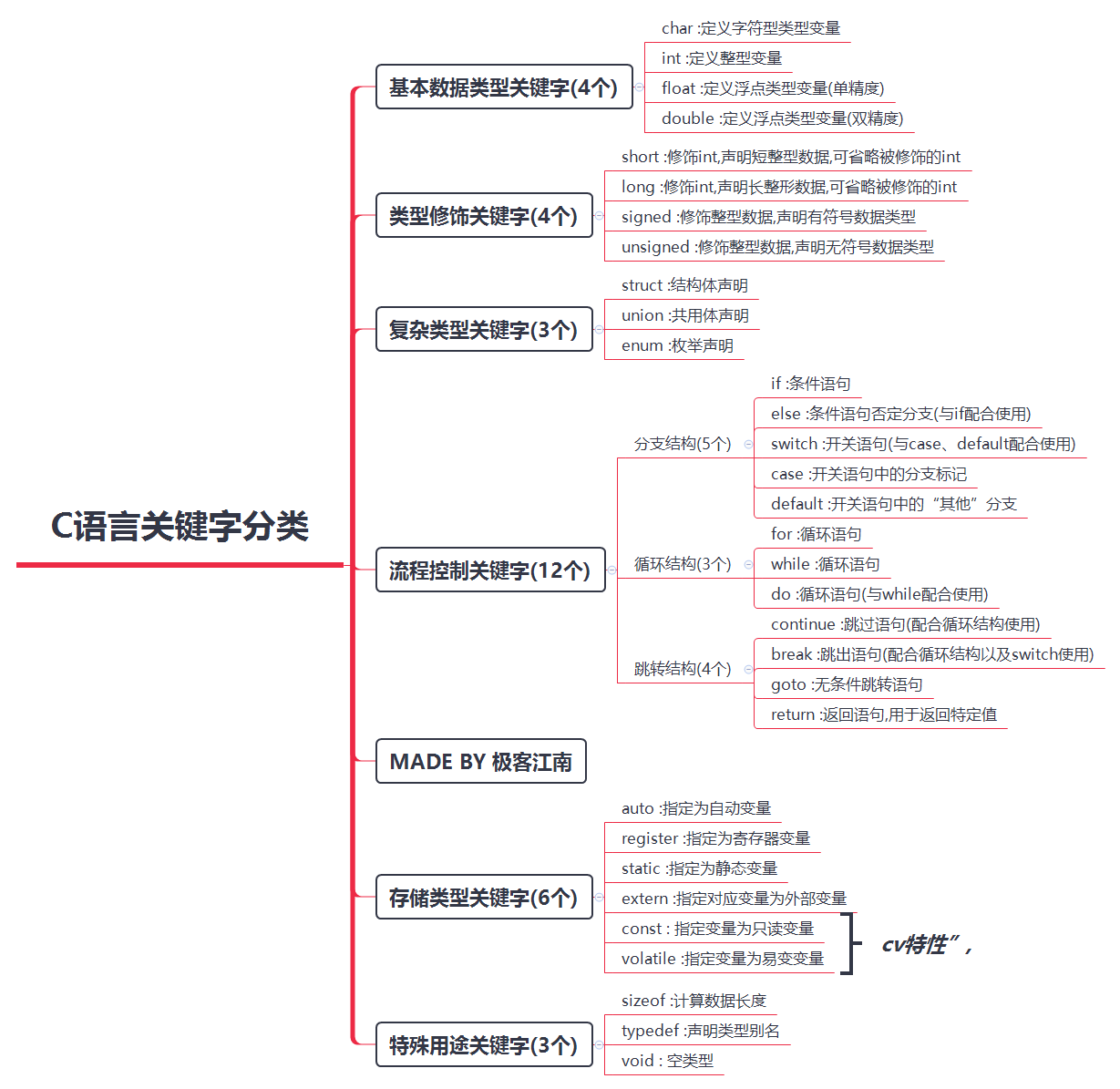## 什么是标识符?

• 从字面上理解,就是用来标识某些东西的符号，标识的目的就是为了将这些东西区分开来
• 其实标识符的作用就跟人类的名字差不多，为了区分每个人，就在每个人出生的时候起了个名字
• C语言是由函数构成的，一个C程序中可能会有多个函数，为了区分这些函数，就给每一个函数都起了个名称, 这个名称就是标识符
• 综上所述: 程序员在程序中给函数、变量等起名字就是标识符

## 标识符命名规则

• 只能由字母(a~z， A~Z)、数字、下划线组成

• 不能包含除下划线以外的其它特殊字符串

• 不能以数字开头

• 不能是C语言中的关键字

• 标识符严格区分大小写, test和Test是两个不同的标识符

## 标识符命名规范

• 见名知意,能够提高代码的可读性

• 驼峰命名,能够提高代码的可读性

• 驼峰命名法就是当变量名或函数名是由多个单词连接在一起,构成标识符时,第一个单词以小写字母开始;第二个单词的首字母大写.

• 例如: myFirstName、myLastName这样的变量名称看上去就像驼峰一样此起彼伏

• [外链图片转存中…(img-ipMTnUHa-1648814825879)]

## 什么是数据？

• 生活中无时无刻都在跟数据打交道

• 例如:人的体重、身高、收入、性别等数据等
• 在我们使用计算机的过程中，也会接触到各种各样的数据

• 例如: 文档数据、图片数据、视频数据等

## 数据分类

• 静态数据是指一些永久性的数据，一般存储在硬盘中。硬盘的存储空间一般都比较大，现在普通计算机的硬盘都有500G左右，因此硬盘中可以存放一些比较大的文件

• 存储的时长：计算机关闭之后再开启，这些数据依旧还在，只要你不主动删掉或者硬盘没坏，这些数据永远都在

• 哪些是静态数据：静态数据一般是以文件的形式存储在硬盘上，比如文档、照片、视频等。

• 动态数据指在程序运行过程中，动态产生的临时数据，一般存储在内存中。内存的存储空间一般都比较小，现在普通计算机的内存只有8G左右，因此要谨慎使用内存，不要占用太多的内存空间

• 存储的时长：计算机关闭之后，这些临时数据就会被清除

• 哪些是动态数据：当运行某个程序（软件）时，整个程序就会被加载到内存中，在程序运行过程中，会产生各种各样的临时数据，这些临时数据都是存储在内存中的。当程序停止运行或者计算机被强制关闭时，这个程序产生的所有临时数据都会被清除。

• 既然硬盘的存储空间这么大，为何不把所有的应用程序加载到硬盘中去执行呢？

• 主要原因就是内存的访问速度比硬盘快N倍
• 静态数据和动态数据的相互转换

​ 也就是从磁盘加载到内存

• 动态数据和静态数据的相互转换

​ 也就是从内存保存到磁盘

• 数据的计量单位
• 不管是静态还是动态数据，都是0和1组成的
• 数据越大，包含的0和1就越多
``````1 B(Byte字节) = 8 bit(位)
// 00000000 就是一个字节
// 111111111 也是一个字节
// 10101010 也是一个字节
// 任意8个0和1的组合都是一个字节
1 KB(KByte) = 1024 B
1 MB = 1024 KB
1 GB = 1024 MB
1 TB = 1024 GB
``````

## C语言数据类型

• 作为程序员, 我们最关心的是内存中的动态数据,因为我们写的程序就是在内存中运行的
• 程序在运行过程中会产生各种各样的临时数据,为了方便数据的运算和操作, C语言对这些数据进行了分类, 提供了丰富的数据类型
• C语言中有4大类数据类型:基本类型、构造类型、指针类型、空类型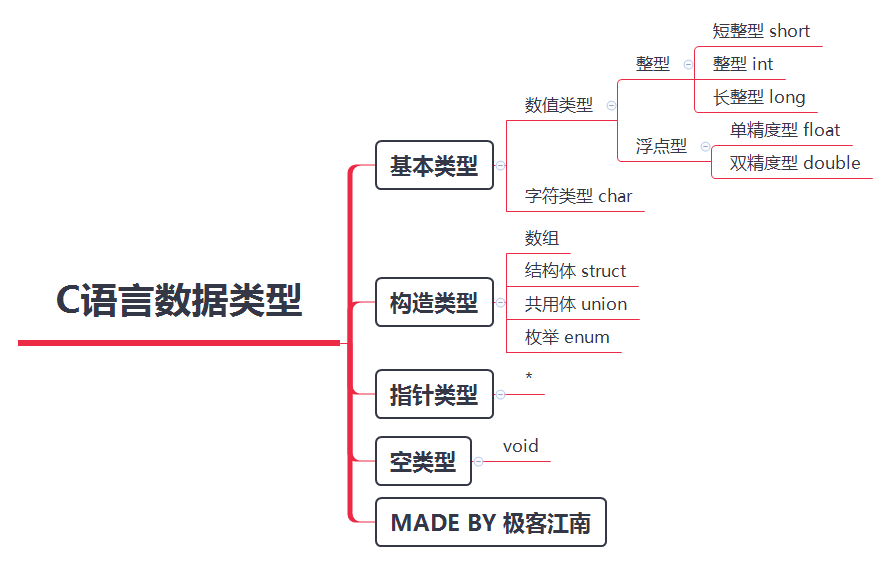## 什么是常量?

• "量"表示数据。常量，则表示一些固定的数据，也就是不能改变的数据
• 就好比现实生活中生男生女一样, 生下来是男孩永远都是男孩, 生下来是女孩就永远都是女孩, 所以性别就是现实生活中常量的一种体现

## 常量的类型

• 十进制整数。例如:666,-120, 0

• 八进制整数,八进制形式的常量都以0开头。例如:0123,也就是十进制的83;-011,也就是十进 制的-9

• 十六进制整数,十六进制的常量都是以0x开头。例如:0x123,也就是十进制的291

• 二进制整数,逢二进一 0b开头。例如: 0b0010,也就是十进制的2

• 小数形式
• 单精度小数:以字母f或字母F结尾。例如:0.0f、1.01f
• 双精度小数:十进制小数形式。例如:3.14、 6.66
• 默认就是双精度
• 可以没有整数位只有小数位。例如: .3、 .6f
• 指数形式
• 以幂的形式表示, 以字母e或字母E后跟一个10为底的幂数
• 上过初中的都应该知道科学计数法吧,指数形式的常量就是科学计数法的另一种表 示,比如123000,用科学计数法表示为1.23×10的5次方
• 用C语言表示就是1.23e5或1.23E5
• 字母e或字母E后面的指数必须为整数
• 字母e或字母E前后必须要有数
• 字母e或字母E前后不能有空格
• 字符常量
• 字符型常量都是用’’(单引号)括起来的。例如:‘a’、‘b’、‘c’
• 字符常量的单引号中只能有一个字符
• 特殊情况: 如果是转义字符,单引号中可以有两个字符。例如:’\n’、’\t’
• 字符串常量
• 字符型常量都是用""(双引号)括起来的。例如:“a”、“abc”、“lnj”
• 系统会自动在字符串常量的末尾加一个字符’\0’作为字符串结束标志

## 什么是变量?

• "量"表示数据。变量，则表示一些不固定的数据，也就是可以改变的数据

• 就好比现实生活中人的身高、体重一样, 随着年龄的增长会不断发生改变, 所以身高、体重就是现实生活中变量的一种体现

• 就好比现实生活中超市的储物格一样, 同一个格子在不同时期不同人使用,格子中存储的物品是可以变化的。张三使用这个格子的时候里面放的可能是尿不湿, 但是李四使用这个格子的时候里面放的可能是面包

## 如何定义变量

• 为什么要定义变量?

• 任何变量在使用之前，必须先进行定义, 只有定义了变量才会分配存储空间, 才有空间存储数据
• 为什么要限定类型?

• 用来约束变量所存放数据的类型。一旦给变量指明了类型，那么这个变量就只能存储这种类型的数据
• 内存空间极其有限,不同类型的变量占用不同大小的存储空间
• 为什么要指定变量名称?

• 存储数据的空间对于我们没有任何意义, 我们需要的是空间中存储的值
• 只有有了名称, 我们才能获取到空间中的值
``````int a;
float b;
char ch;
``````
• 格式2:变量类型 变量名称,变量名称;
• 连续定义, 多个变量之间用逗号(,)号隔开
``````int a,b,c;
``````
• 变量名的命名的规范
• 变量名属于标识符,所以必须严格遵守标识符的命名原则

## 如何使用变量？

• 可以利用=号往变量里面存储数据
• 在C语言中,利用=号往变量里面存储数据, 我们称之为给变量赋值
``````int value;
value = 998; // 赋值
``````

• 这里的=号，并不是数学中的“相等”，而是C语言中的赋值运算符，作用是将右边的整型常量998赋值给左边的整型变量value

• 赋值的时候,= 号的左侧必须是变量 (10=b,错误)
• 为了方便阅读代码, 习惯在 = 的两侧 各加上一个 空格

## 变量的初始化

• C语言中, 变量的第一次赋值，我们称为“初始化”
• 初始化的两种形式
• 先定义,后初始化
• `int value; value = 998; // 初始化`
• 定义时同时初始化
• `int a = 10; int b = 4, c = 2;`
• 其它表现形式(不推荐)
``````int a, b = 10; //部分初始化
int c, d, e;
c = d = e =0;
``````
• 不初始化里面存储什么?
• 随机数
• 上次程序分配的存储空间,存数一些 内容,“垃圾”
• 系统正在用的一些数据

## 如何修改变量值?

• 多次赋值即可
• 每次赋值都会覆盖原来的值
``````int i = 10;
i = 20; // 修改变量的值
``````

## 变量之间的值传递

• 可以将一个变量存储的值赋值给另一个变量
``````int a = 10;
int b = a; // 相当于把a中存储的10拷贝了一份给b
``````

## 如何查看变量的值?

• 使用printf输出一个或多个变量的值
``````int a = 10, c = 11;
printf("a=%d, c=%d", a, c);
``````

``printf("%#o %#d %#x",a,a,a);//带个#就是输出带前缀的意思，o，d。x分别代表八进制，十进制，十六进制``
• 输出其它类型变量的值
``````double height = 1.75;
char blood = 'A';
printf("height=%.2f, 血型是%c", height,  blood);
``````

## 变量的作用域

• C语言中所有变量都有自己的作用域

• 变量定义的位置不同,其作用域也不同

• 按照作用域的范围可分为两种, 即局部变量和全局变量

• 局部变量

• 局部变量也称为内部变量
• 局部变量是在代码块内定义的, 其作用域仅限于代码块内, 离开该代码块后无法使用
``````int main(){
int i = 998; // 作用域开始
return 0;// 作用域结束
}
``````
``````int main(){
{
int i = 998; // 作用域开始
}// 作用域结束
printf("i = %d\n", i); // 不能使用
return 0;
}
``````
``````int main(){
{
{
int i = 998;// 作用域开始
}// 作用域结束
printf("i = %d\n", i); // 不能使用
}
return 0;
}
``````
• 全局变量
• 全局变量也称为外部变量,它是在代码块外部定义的变量
``````int i = 666;
int main(){
printf("i = %d\n", i); // 可以使用
return 0;
}// 作用域结束
int call(){
printf("i = %d\n", i); // 可以使用
return 0;
}
``````
• 注意点:
• 同一作用域范围内不能有相同名称的变量
``````int main(){
int i = 998; // 作用域开始
int i = 666; // 报错, 重复定义
return 0;
}// 作用域结束
``````
``````int i = 666;
int i = 998; // 报错, 重复定义
int main(){
return 0;
}
``````
• 不同作用域范围内可以有相同名称的变量
``````int i = 666;
int main(){
int i = 998; // 不会报错
return 0;
}
``````
``````int main(){
int i = 998; // 不会报错
return 0;
}
int call(){
int i = 666;  // 不会报错
return 0;
}
``````

## 变量内存分析（简单版）

• 字节和地址
• 为了更好地理解变量在内存中的存储细节，先来认识一下内存中的“字节”和“地址”
• 每一个小格子代表一个字节
• 每个字节都有自己的内存地址
• 内存地址是连续的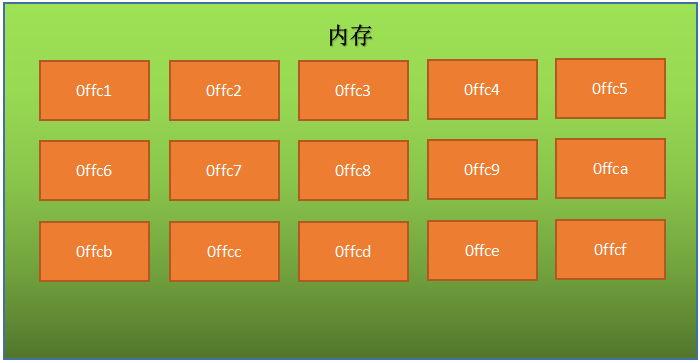• 变量存储占用的空间
• 一个变量所占用的存储空间，和定义变量时声明的类型以及当前编译环境有关

char 1 1 1
int 2 4 4
float 4 4 4
double 8 8 8
short 2 2 2
long 4 4 8
long long 8 8 8
void* 2 4 8

• 根据定义变量时声明的类型和当前编译环境确定需要开辟多大存储空间
• 在内存中开辟一块存储空间，开辟时从内存地址大的开始开辟（内存寻址从大到小）
• 将数据保存到已经开辟好的对应内存空间中
``````int main(){
int number;
int value;
number = 22;
value = 666;
}
``````
``````#include <stdio.h>
int main(){
int number;
int value;
number = 22;
value = 666;
printf("&number = %p\n", &number); // 0060FEAC
printf("&value = %p\n", &value);   // 0060FEA8
}
``````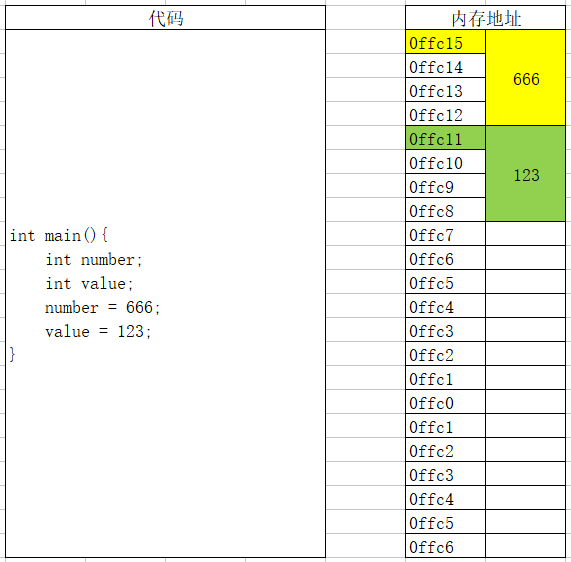## printf函数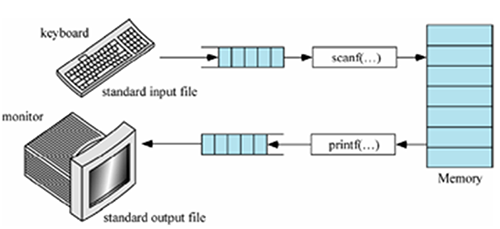• printf函数称之为格式输出函数,方法名称的最后一个字母f表示format。其功能是按照用户指定的格式,把指定的数据输出到屏幕上
• printf函数的调用格式为:
• printf(“格式控制字符串”,输出项列表 );
• 例如:printf(“a = %d, b = %d”,a, b);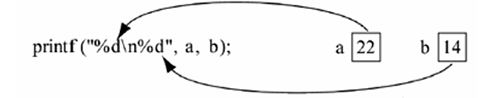• 非格式字符串原样输出, 格式控制字符串会被输出项列表中的数据替换

• 注意: 格式控制字符串和输出项在数量和类型上必须一一对应

• 格式控制字符串

• 形式: `%[标志][输出宽度][.精度][长度]类型`
• 类型

• 格式: `printf("a = %类型", a);`
• 类型字符串用以表示输出数据的类型, 其格式符和意义如下所示

d 有符号10进制整型
i 有符号10进制整型
u 无符号10进制整型
o 无符号8进制整型
x 无符号16进制整型
X 无符号16进制整型
f 单、双精度浮点数(默认保留6位小数)
e / E 以指数形式输出单、双精度浮点数
g / G 以最短输出宽度,输出单、双精度浮点数
c 字符
s 字符串
p 地址
``````#include <stdio.h>
int main(){
int a = 10;
int b = -10;
float c = 6.6f;
double d = 3.1415926;
double e = 10.10;
char f = 'a';
// 有符号整数(可以输出负数)
printf("a = %d\n", a); // 10
printf("a = %i\n", a); // 10

// 无符号整数(不可以输出负数)
printf("a = %u\n", a); // 10
printf("b = %u\n", b); // 429496786

// 无符号八进制整数(不可以输出负数)
printf("a = %o\n", a); // 12
printf("b = %o\n", b); // 37777777766

// 无符号十六进制整数(不可以输出负数)
printf("a = %x\n", a); // a
printf("b = %x\n", b); // fffffff6

// 无符号十六进制整数(不可以输出负数)
printf("a = %X\n", a); // A
printf("b = %X\n", b); // FFFFFFF6

// 单、双精度浮点数(默认保留6位小数)
printf("c = %f\n", c); // 6.600000
printf("d = %lf\n", d); // 3.141593

// 以指数形式输出单、双精度浮点数
printf("e = %e\n", e); // 1.010000e+001
printf("e = %E\n", e); // 1.010000E+001

// 以最短输出宽度,输出单、双精度浮点数
printf("e = %g\n", e); // 10.1
printf("e = %G\n", e); // 10.1

// 输出字符
printf("f = %c\n", f); // a
}
``````
• 宽度
• 格式: `printf("a = %[宽度]类型", a);`
• 用十进制整数来指定输出的宽度, 如果实际位数多于指定宽度,则按照实际位数输出, 如果实际位数少于指定宽度则以空格补位
``````#include <stdio.h>
int main(){
// 实际位数小于指定宽度
int a = 1;
printf("a =|%d|\n", a); // |1|
printf("a =|%5d|\n", a); // |    1|
// 实际位数大于指定宽度
int b = 1234567;
printf("b =|%d|\n", b); // |1234567|
printf("b =|%5d|\n", b); // |1234567|
}
``````
• 标志
• 格式: `printf("a = %[标志][宽度]类型", a);`

- 左对齐, 默认右对齐
+ 当输出值为正数时,在输出值前面加上一个+号, 默认不显示
0 右对齐时, 用0填充宽度.(默认用空格填充)

# 对c、s、d、u类型无影响
# 对o类型, 在输出时加前缀o
# 对x类型,在输出时加前缀0x
``````#include <stdio.h>
int main(){
int a = 1;
int b = -1;
// -号标志
printf("a =|%d|\n", a); // |1|
printf("a =|%5d|\n", a); // |    1|
printf("a =|%-5d|\n", a);// |1    |
// +号标志
printf("a =|%d|\n", a); // |1|
printf("a =|%+d|\n", a);// |+1|
printf("b =|%d|\n", b); // |-1|
printf("b =|%+d|\n", b);// |-1|
// 0标志
printf("a =|%5d|\n", a); // |    1|
printf("a =|%05d|\n", a); // |00001|
// 空格标志
printf("a =|% d|\n", a); // | 1|
printf("b =|% d|\n", b); // |-1|
// #号
int c = 10;
printf("c = %o\n", c); // 12
printf("c = %#o\n", c); // 012
printf("c = %x\n", c); // a
printf("c = %#x\n", c); // 0xa
}
``````
• 精度
• 格式: `printf("a = %[精度]类型", a);`
• 精度格式符以"."开头, 后面跟上十进制整数, 用于指定需要输出多少位小数, 如果输出位数大于指定的精度, 则删除超出的部分
``````#include <stdio.h>
int main(){
double a = 3.1415926;
printf("a = %.2f\n", a); // 3.14
}
``````
• 动态指定保留小数位数
• 格式: `printf("a = %.*f", a);`
``````#include <stdio.h>
int main(){
double a = 3.1415926;
printf("a = %.*f", 2, a); // 3.14
}
``````
• 实型(浮点类型)有效位数问题
• 对于单精度数,使用%f格式符输出时,仅前6~7位是有效数字
• 对于双精度数,使用%lf格式符输出时,前15~16位是有效数字
• 有效位数和精度(保留多少位)不同, 有效位数是指从第一个非零数字开始,误差不超过本数位半个单位的、精确可信的数位
• 有效位数包含小数点前的非零数位
``````#include <stdio.h>
int main(){
//        1234.567871093750000
float a = 1234.567890123456789;
//         1234.567890123456900
double b = 1234.567890123456789;
printf("a = %.15f\n", a); // 前8位数字是准确的, 后面的都不准确
printf("b = %.15f\n", b); // 前16位数字是准确的, 后面的都不准确
}
``````
• 长度
• 格式: `printf("a = %[长度]类型", a);`

hh d、i、o、u、x 输出char
h d、i、o、u、x 输出 short int
l d、i、o、u、x 输出 long int
ll d、i、o、u、x 输出 long long int
``````#include <stdio.h>
int main(){
char a = 'a';
short int b = 123;
int  c = 123;
long int d = 123;
long long int e = 123;
printf("a = %hhd\n", a); // 97
printf("b = %hd\n", b); // 123
printf("c = %d\n", c); // 123
printf("d = %ld\n", d); // 123
printf("e = %lld\n", e); // 123
}
``````
• 转义字符
• 格式: `printf("%f%%", 3.1415);`
• %号在格式控制字符串中有特殊含义, 所以想输出%必须添加一个转移字符
``````#include <stdio.h>
int main(){
printf("%f%%", 3.1415); // 输出结果3.1415%
}
``````

## scanf函数

• scanf函数用于接收键盘输入的内容, 是一个阻塞式函数,程序会停在scanf函数出现的地方, 直到接收到数据才会执行后面的代码

• printf函数的调用格式为:

• `scanf("格式控制字符串", 地址列表);`
• 例如: `scanf("%d", &num);`
• 基本用法

• 地址列表项中只能传入变量地址, 变量地址可以通过&符号+变量名称的形式获取
``````#include <stdio.h>
int main(){
int number;
scanf("%d", &number); // 接收一个整数
printf("number = %d\n", number);
}
c``````
• 接收非字符和字符串类型时, 空格、Tab和回车会被忽略
``````#include <stdio.h>
int main(){
float num;
// 例如:输入 Tab 空格 回车 回车 Tab 空格 3.14 , 得到的结果还是3.14
scanf("%f", &num);
printf("num = %f\n", num);
}
``````
• 非格式字符串原样输入, 格式控制字符串会赋值给地址项列表项中的变量
• 不推荐这种写法
``````#include <stdio.h>
int main(){
int number;
// 用户必须输入number = 数字  , 否则会得到一个意外的值
scanf("number = %d", &number);
printf("number = %d\n", number);
}
``````
• 接收多条数据
• 格式控制字符串和地址列表项在数量和类型上必须一一对应
• 非字符和字符串情况下如果没有指定多条数据的分隔符, 可以使用空格或者回车作为分隔符(不推荐这种写法)
• 非字符和字符串情况下建议明确指定多条数据之间分隔符
``````#include <stdio.h>
int main(){
int number;
scanf("%d", &number);
printf("number = %d\n", number);
int value;
scanf("%d", &value);
printf("value = %d\n", value);
}
``````
``````#include <stdio.h>
int main(){
int number;
int value;
// 可以输入 数字 空格 数字, 或者 数字 回车 数字
scanf("%d%d", &number, &value);
printf("number = %d\n", number);
printf("value = %d\n", value);
}
``````
``````#include <stdio.h>
int main(){
int number;
int value;
// 输入 数字,数字 即可
scanf("%d,%d", &number, &value);
printf("number = %d\n", number);
printf("value = %d\n", value);
}
``````
• \n是scanf函数的结束符号, 所以格式化字符串中不能出现\n
``````#include <stdio.h>
int main(){
int number;
// 输入完毕之后按下回车无法结束输入
scanf("%d\n", &number);
printf("number = %d\n", number);
}
``````

## scanf运行原理

• 系统会将用户输入的内容先放入输入缓冲区
• scanf方式会从输入缓冲区中逐个取出内容赋值给变量
• 如果输入缓冲区的内容不为空,scanf会一直从缓冲区中获取,而不要求再次输入
``````#include <stdio.h>
int main(){
int num1;
int num2;
char ch1;
scanf("%d%c%d", &num1, &ch1, &num2);
printf("num1 = %d, ch1 = %c, num2 = %d\n", num1, ch1, num2);
char ch2;
int num3;
scanf("%c%d",&ch2, &num3);
printf("ch2 = %c, num3 = %d\n", ch2, num3);
}
``````
• 利用fflush方法清空缓冲区(不是所有平台都能使用)

• 格式: fflush(stdin);
• C和C++的标准里从来没有定义过 fflush(stdin)
• MSDN 文档里清除的描述着"fflush on input stream is an extension to the C standard" （fflush 是在标准上扩充的函数, 不是标准函数, 所以不是所有平台都支持）
• 利用setbuf方法清空缓冲区(所有平台有效)

• 格式: `setbuf(stdin, NULL);`
``````#include <stdio.h>
int main(){
int num1;
int num2;
char ch1;
scanf("%d%c%d", &num1, &ch1, &num2);
printf("num1 = %d, ch1 = %c, num2 = %d\n", num1, ch1, num2);
//fflush(stdin); // 清空输入缓存区
setbuf(stdin, NULL); // 清空输入缓存区
char ch2;
int num3;
scanf("%c%d",&ch2, &num3);
printf("ch2 = %c, num3 = %d\n", ch2, num3);
}
``````

## putchar和getchar

• putchar: 向屏幕输出一个字符
``````#include <stdio.h>
int main(){
char ch = 'a';
putchar(ch); // 输出a
}
``````
• getchar: 从键盘获得一个字符
``````#include <stdio.h>
int main(){
char ch;
ch = getchar();// 获取一个字符
printf("ch = %c\n", ch);
}
``````

## 运算符基本概念

• 例如告诉程序, 某两个数相加, 相减,相乘等

• 什么是表达式

• 表达式就是利用运算符链接在一起的有意义,有结果的语句;
• 例如: a + b; 就是一个算数表达式, 它的意义是将两个数相加, 两个数相加的结果就是表达式的结果
• 注意: 表达式一定要有结果

## 运算符分类

• 按照功能划分:
• 算术运算符
• 赋值运算符
• 关系运算符
• 逻辑运算符
• 位运算符
• 按照参与运算的操作数个数划分:
• 单目运算
• 只有一个操作数 如 : i++;
• 双目运算
• 有两个操作数 如 : a + b;
• 三目运算
• C语言中唯一的一个,也称为问号表达式 如: a>b ? 1 : 0;

## 运算符的优先级和结合性

• 早在小学的数学课本中,我们就学习过"从左往右,先乘除后加减,有括号的先算括号里面的", 这句话就蕴含了优先级和结合性的问题
• C语言中,运算符的运算优先级共分为15 级。1 级最高,15 级最低
• 在C语言表达式中,不同优先级的运算符, 运算次序按照由高到低执行
• 在C语言表达式中,相同优先级的运算符, 运算次序按照结合性规定的方向执行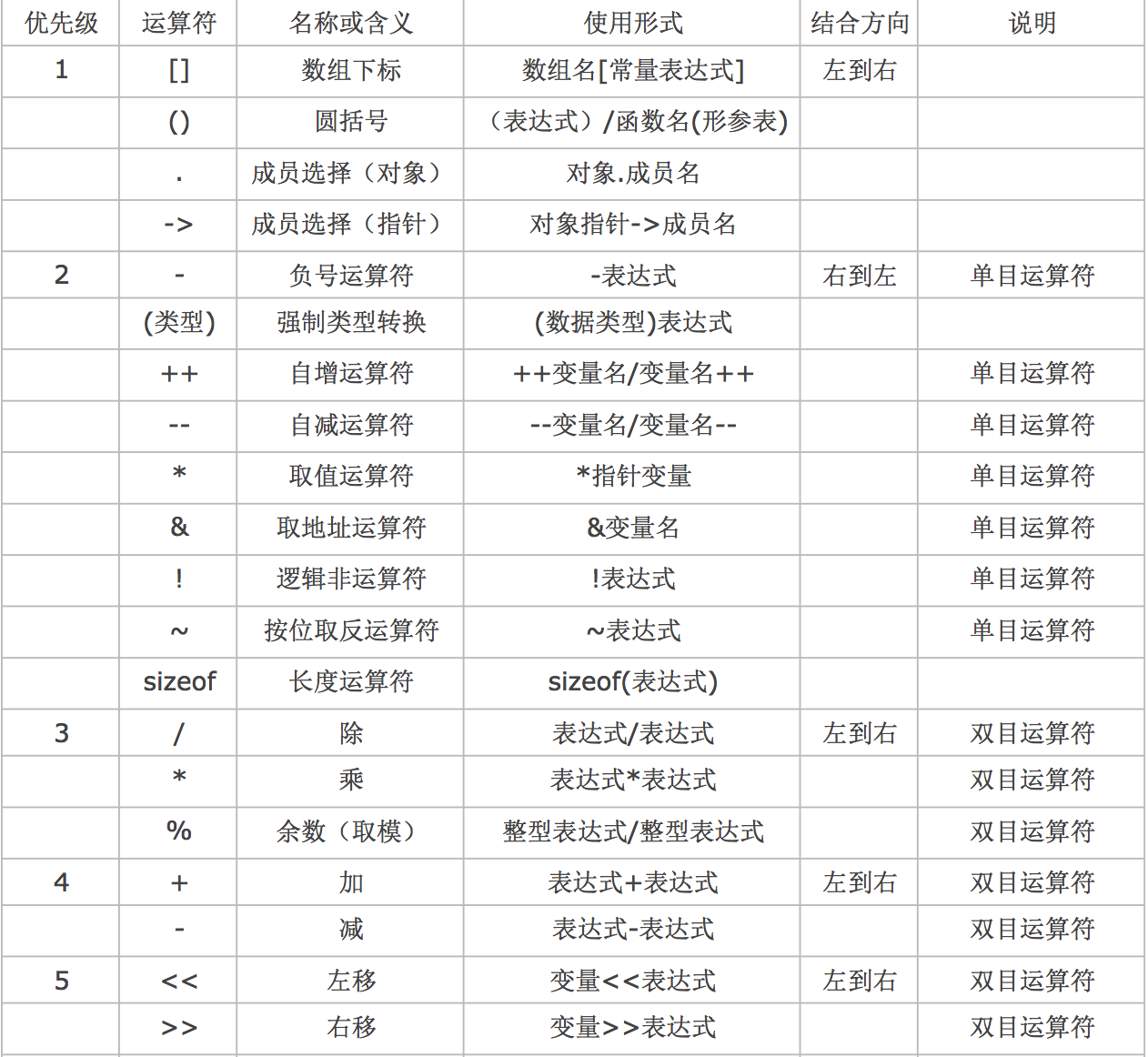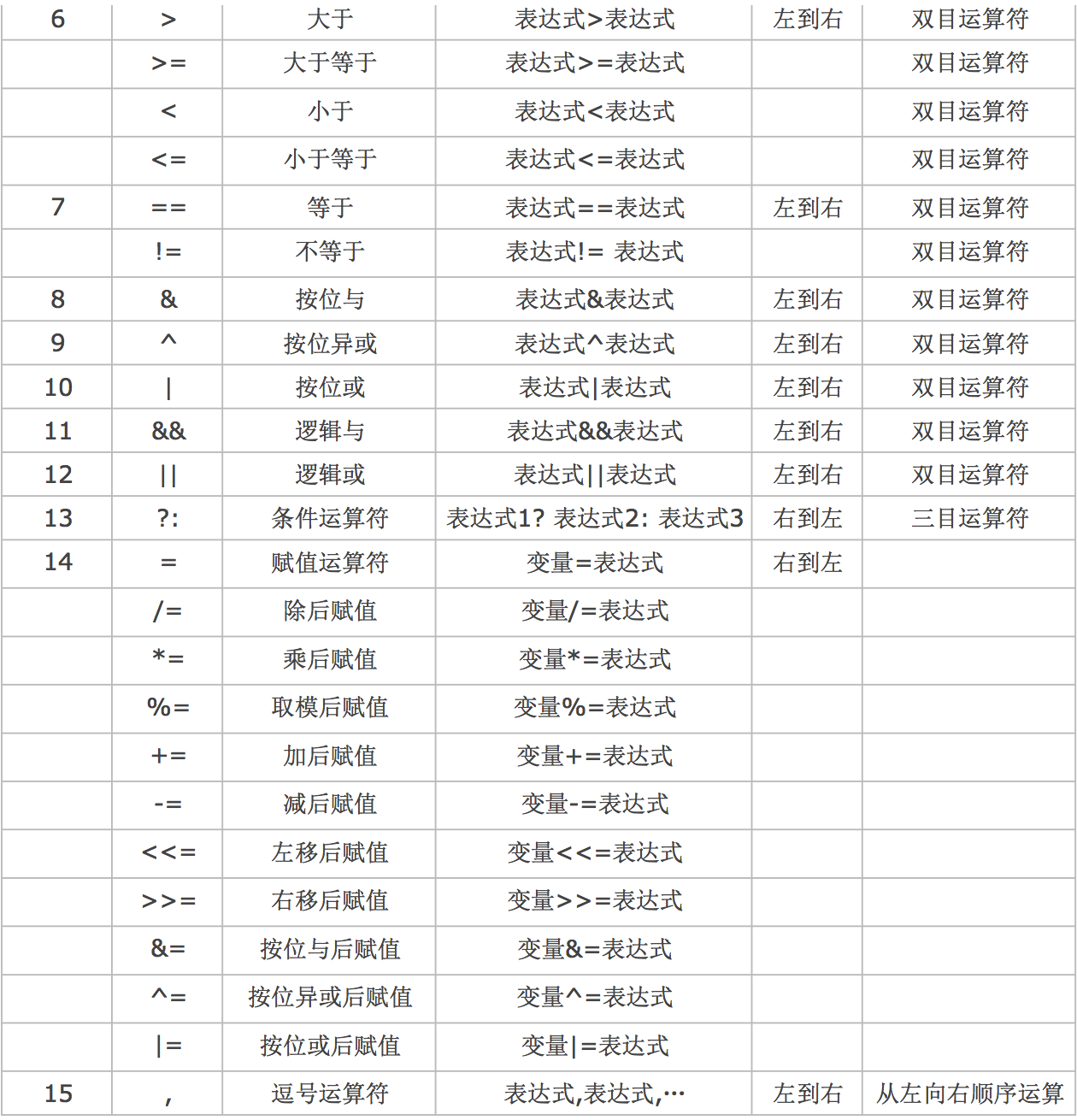## 算数运算符

3 乘法运算符 * 双目运算符,具有左结合性
3 除法运算符 / 双目运算符,具有左结合性
3 求余运算符 (模运算符) % 双目运算符,具有左结合性
4 加法运算符 + 双目运算符,具有左结合性
4 减法运算符 - 双目运算符,具有左结合性

• 如果参与运算的两个操作数皆为整数, 那么结果也为整数
• 如果参与运算的两个操作数其中一个是浮点数, 那么结果一定是浮点数
• 求余运算符, 本质上就是数学的商和余"中的余数
• 求余运算符, 参与运算的两个操作数必须都是整数, 不能包含浮点数
• 求余运算符, 被除数小于除数, 那么结果就是被除数
• 求余运算符, 运算结果的正负性取决于被除数,跟除数无关, 被除数是正数结果就是正数,被除数是负数结果就是负数
• 求余运算符, 被除数为0, 结果为0
• 求余运算符, 除数为0, 没有意义(不要这样写)
``````#include <stdio.h>
int main(){
int a = 10;
int b = 5;
// 加法
int result = a + b;
printf("%i\n", result); // 15
// 减法
result = a - b;
printf("%i\n", result); // 5
// 乘法
result = a * b;
printf("%i\n", result); // 50
// 除法
result = a / b;
printf("%i\n", result); // 2

// 算术运算符的结合性和优先级
// 结合性: 左结合性, 从左至右
int c = 50;
result = a + b + c; // 15 + c;  65;
printf("%i\n", result);

// 优先级: * / % 大于 + -
result = a + b * c; // a + 250; 260;
printf("%i\n", result);
}
``````
``````#include <stdio.h>
int main(){
// 整数除以整数, 结果还是整数
printf("%i\n", 10 / 3); // 3

// 参与运算的任何一个数是小数, 结果就是小数
printf("%f\n", 10 / 3.0); // 3.333333
}
``````
``````#include <stdio.h>
int main(){
// 10 / 3 商等于3, 余1
int result = 10 % 3;
printf("%i\n", result); // 1

// 左边小于右边, 那么结果就是左边
result = 2 % 10;
printf("%i\n", result); // 2

// 被除数是正数结果就是正数,被除数是负数结果就是负数
result = 10 % 3;
printf("%i\n", result); // 1
result = -10 % 3;
printf("%i\n", result); // -1
result = 10 % -3;
printf("%i\n", result); // 1
}
``````

## 赋值运算符

14 赋值运算符 = 双目运算符,具有右结合性
14 除后赋值运算符 /= 双目运算符,具有右结合性
14 乘后赋值运算符 (模运算符) *= 双目运算符,具有右结合性
14 取模后赋值运算符 %= 双目运算符,具有右结合性
14 加后赋值运算符 += 双目运算符,具有右结合性
14 减后赋值运算符 -= 双目运算符,具有右结合性
• 简单赋值运算符
``````#include <stdio.h>
int main(){
// 简单的赋值运算符 =
// 会将=右边的值赋值给左边
int a = 10;
printf("a = %i\n", a); // 10
}
``````
• 复合赋值运算符
``````#include <stdio.h>
int main(){
// 复合赋值运算符 += -= *= /= %=
// 将变量中的值取出之后进行对应的操作, 操作完毕之后再重新赋值给变量
int num1 = 10;
// num1 = num1 + 1; num1 = 10 + 1; num1 = 11;
num1 += 1;
printf("num1 = %i\n", num1); // 11
int num2 = 10;
// num2 = num2 - 1; num2 = 10 - 1; num2 = 9;
num2 -= 1;
printf("num2 = %i\n", num2); // 9
int num3 = 10;
// num3 = num3 * 2; num3 = 10 * 2; num3 = 20;
num3 *= 2;
printf("num3 = %i\n", num3); // 20
int num4 = 10;
// num4 = num4 / 2; num4 = 10 / 2; num4 = 5;
num4 /= 2;
printf("num4 = %i\n", num4); // 5
int num5 = 10;
// num5 = num5 % 3; num5 = 10 % 3; num5 = 1;
num5 %= 3;
printf("num5 = %i\n", num5); // 1
}
``````
• 结合性和优先级
``````#include <stdio.h>
int main(){
int number = 10;
// 赋值运算符优先级是14, 普通运算符优先级是3和4, 所以先计算普通运算符
// 普通运算符中乘法优先级是3, 加法是4, 所以先计算乘法
// number += 1 + 25; number += 26; number = number + 26; number = 36;
number += 1 + 5 * 5;
printf("number = %i\n", number); // 36
}
``````

## 自增自减运算符

• 在程序设计中,经常遇到“i=i+1”和“i=i-1”这两种极为常用的操作。
• C语言为这种操作提供了两个更为简洁的运算符,即++和–

2 自增运算符(在后) i++ 单目运算符,具有左结合性
2 自增运算符(在前) ++i 单目运算符,具有右结合性
2 自减运算符(在后) i– 单目运算符,具有左结合性
2 自减运算符(在前) –i 单目运算符,具有右结合性
• 自增
• 如果只有单个变量, 无论++写在前面还是后面都会对变量做+1操作
``````#include <stdio.h>
int main(){
int number = 10;
number++;
printf("number = %i\n", number); // 11
++number;
printf("number = %i\n", number); // 12
}
``````
• 如果出现在一个表达式中, 那么++写在前面和后面就会有所区别
• 前缀表达式:++x, --x;其中x表示变量名,先完成变量的自增自减1运算,再用x的值作为表达式的值;即“先变后用”,也就是变量的值先变,再用变量的值参与运算
• 后缀表达式:x++, x–;先用x的当前值作为表达式的值,再进行自增自减1运算。即“先用后变”,也就是先用变量的值参与运算,变量的值再进行自增自减变化
``````#include <stdio.h>
int main(){
int number = 10;
// ++在后, 先参与表达式运算, 再自增
// 表达式运算时为: 3 + 10;
int result = 3 + number++;
printf("result = %i\n", result); // 13
printf("number = %i\n", number); // 11
}
``````
``````#include <stdio.h>
int main(){
int number = 10;
// ++在前, 先自增, 再参与表达式运算
// 表达式运算时为: 3 + 11;
int result = 3 + ++number;
printf("result = %i\n", result); // 14
printf("number = %i\n", number); // 11
}
``````
• 自减
``````#include <stdio.h>
int main(){
int number = 10;
// --在后, 先参与表达式运算, 再自减
// 表达式运算时为: 10 + 3;
int result = number-- + 3;
printf("result = %i\n", result); // 13
printf("number = %i\n", number); // 9
}
``````
``````#include <stdio.h>
int main(){
int number = 10;
// --在前, 先自减, 再参与表达式运算
// 表达式运算时为: 9 + 3;
int result = --number + 3;
printf("result = %i\n", result); // 12
printf("number = %i\n", number); // 9
}
``````
• 注意点:
• 自增、自减运算只能用于单个变量,只要是标准类型的变量,不管是整型、实型,还是字符型变量等,但不能用于表达式或常量
• 错误用法: `++(a+b); 5++;`
• 企业开发中尽量让++ – 单独出现, 尽量不要和其它运算符混合在一起
``````int i = 10;
int b = i++; // 不推荐

int b = ++i; // 不推荐

int a = 10;
int b = ++a + a++;  // 不推荐
``````
• 请用如下代码替代
``````int i = 10;
int b = i; // 推荐
i++;

i++;
int b = i; // 推荐

int a = 10;
++a;
int b = a + a; // 推荐
a++;
``````
• C语言标准没有明确的规定，`同一个表达式中同一个变量自增或自减后如何运算`, 不同编译器得到结果也不同, 在企业开发中千万不要这样写
`````` int a = 1;
// 下列代码利用Qt运行时6, 利用Xcode运行是5
// 但是无论如何, 最终a的值都是3
//  在C语言中这种代码没有意义, 不用深究也不要这样写
// 特点: 参与运算的是同一个变量, 参与运算时都做了自增自减操作, 并且在同一个表达式中
int b = ++a + ++a;
printf("b = %i\n", b);
``````

## sizeof运算符

• sizeof可以用来计算一个变量或常量、数据类型所占的内存字节数

• 标准格式: sizeof(常量 or 变量);
• sizeof的几种形式

• sizeof( 变量\常量 );
• sizeof(10);
• char c = ‘a’; sizeof©;
• sizeof 变量\常量;
• sizeof 10;
• char c = ‘a’; sizeof c;
• sizeof( 数据类型);
• sizeof(float);
• 如果是数据类型不能省略括号
• sizeof面试题:

• sizeof()和+=、*=一样是一个复合运算符, 由sizeof和()两个部分组成, 但是代表的是一个整体
• 所以sizeof不是一个函数, 是一个运算符, 该运算符的优先级是2
``````#include <stdio.h>
int main(){
int a = 10;
double b = 3.14;
// 由于sizeof的优先级比+号高, 所以会先计算sizeof(a);
// a是int类型, 所以占4个字节得到结果4
// 然后再利用计算结果和b相加, 4 + 3.14 = 7.14
double res = sizeof a+b;
printf("res = %lf\n", res); // 7.14
}
``````

## 逗号运算符

• 在C语言中逗号“,”也是一种运算符,称为逗号运算符。 其功能是把多个表达式连接起来组成一个表达式,称为逗号表达式
• 逗号运算符会从左至右依次取出每个表达式的值, 最后整个逗号表达式的值等于最后一个表达式的值
• 格式: 表达式1，表达式2，… …，表达式n;
• 例如: int result = a+1,b=3*4;
``````#include <stdio.h>
int main(){
int a = 10, b = 20, c;
// ()优先级高于逗号运算符和赋值运算符, 所以先计算()中的内容
// c = (11, 21);
// ()中是一个逗号表达式, 结果是最后一个表达式的值, 所以计算结果为21
// 将逗号表达式的结果赋值给c, 所以c的结果是21
c = (a + 1, b + 1);
printf("c = %i\n", c); // 21
}
``````

## 关系运算符

• 为什么要学习关系运算符
• 默认情况下，我们在程序中写的每一句正确代码都会被执行。但很多时候，我们想在某个条件成立的情况下才执行某一段代码
• 这种情况的话可以使用条件语句来完成，但是学习条件语句之前，我们先来看一些更基础的知识：如何判断一个条件是否成立

C语言中的真假性

• 在C语言中，条件成立称为“真”，条件不成立称为“假”，因此，判断条件是否成立，就是判断条件的“真假”

• 怎么判断真假呢？C语言规定，任何数值都有真假性，任何非0值都为“真”，只有0才为“假”。也就是说，108、-18、4.5、-10.5等都是“真”，0则是“假”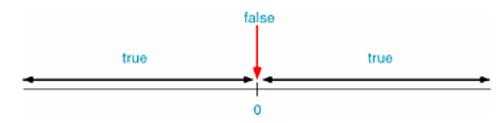• 关系运算符的运算结果只有2种：如果条件成立，结果就为1，也就是“真”；如果条件不成立，结果就为0，也就是“假”

6 大于运算符 > 双目运算符,具有左结合性
6 小于运算符 < 双目运算符,具有左结合性
6 大于等于运算符 >= 双目运算符,具有左结合性
6 小于等于运算符 <= 双目运算符,具有左结合性
7 等于运算符 == 双目运算符,具有左结合性
7 不等于运算符 != 双目运算符,具有左结合性
``````#include <stdio.h>
int main(){
int result = 10 > 5;
printf("result = %i\n", result); // 1
result = 5 < 10;
printf("result = %i\n", result); // 1
result = 5 > 10;
printf("result = %i\n", result); // 0
result = 10 >= 10;
printf("result = %i\n", result); // 1
result = 10 <= 10;
printf("result = %i\n", result); // 1
result = 10 == 10;
printf("result = %i\n", result); // 1
result = 10 != 9;
printf("result = %i\n", result); // 1
}
``````
• 优先级和结合性
``````#include <stdio.h>
int main(){
// == 优先级 小于 >, 所以先计算>
// result = 10 == 1; result = 0;
int result = 10 == 5 > 3;
printf("result = %i\n", result); // 0
}
``````
``````#include <stdio.h>
int main(){
// == 和 != 优先级一样, 所以按照结合性
// 关系运算符是左结合性, 所以从左至右计算
// result = 0 != 3; result = 1;
int result = 10 == 5 != 3;
printf("result = %i\n", result); // 1
}
``````
• 注意点:
• 无论是float还是double都有精度问题, 所以一定要避免利用==判断浮点数是否相等
``````#include <stdio.h>
int main(){
float a = 0.1;
float b = a * 10 + 0.00000000001;
double c = 1.0 + + 0.00000000001;
printf("b = %f\n", b);
printf("c = %f\n", c);
int result = b == c;
printf("result = %i\n", result); // 0
}
``````

## 逻辑运算符

2 逻辑非运算符 ! 单目运算符,具有右结合性
11 逻辑与运算符 && 双目运算符,具有左结合性
12 逻辑或运算符 ||
• 逻辑非
• 格式: `! 条件A;`
• 运算结果: 真变假,假变真
• 运算过程:
• 先判断条件A是否成立，如果添加A成立, 那么结果就为0，即“假”；
• 如果条件A不成立，结果就为1，即“真”
• 使用注意:
• 可以多次连续使用逻辑非运算符
• !!!0;相当于(!(!(!0)));最终结果为1
``````#include <stdio.h>
int main(){
// ()优先级高, 先计算()里面的内容
// 10==10为真, 所以result = !(1);
// !代表真变假, 假变真,所以结果是假0
int result = !(10 == 10);
printf("result = %i\n", result); // 0
}
``````
• 逻辑与
• 格式: `条件A && 条件B;`
• 运算结果:一假则假
• 运算过程:
• 总是先判断"条件A"是否成立
• 如果"条件A"成立，接着再判断"条件B"是否成立, 如果"条件B"也成立，结果就为1，即“真”
• 如果"条件A"成立，"条件B"不成立，结果就为0，即“假”
• 如果"条件A"不成立，不会再去判断"条件B"是否成立, 因为逻辑与只要一个不为真结果都不为真
• 使用注意:
• "条件A"为假, "条件B"不会被执行
``````#include <stdio.h>
int main(){
//               真     &&    真
int result = (10 == 10) && (5 != 1);
printf("result = %i\n", result); // 1
//          假     &&    真
result = (10 == 9) && (5 != 1);
printf("result = %i\n", result); // 0
//          真     &&    假
result = (10 == 10) && (5 != 5);
printf("result = %i\n", result); // 0
//          假     &&    假
result = (10 == 9) && (5 != 5);
printf("result = %i\n", result); // 0
}
``````
``````#include <stdio.h>
int main(){
int a = 10;
int b = 20;
// 逻辑与, 前面为假, 不会继续执行后面
int result = (a == 9) && (++b);
printf("result = %i\n", result); // 1
printf("b = %i\n", b); // 20
}
``````
• 逻辑或
• 格式: `条件A || 条件B;`
• 运算结果:一真则真
• 运算过程:
• 总是先判断"条件A"是否成立
• 如果"条件A"不成立，接着再判断"条件B"是否成立, 如果"条件B"成立，结果就为1，即“真”
• 如果"条件A"不成立，"条件B"也不成立成立, 结果就为0，即“假”
• 如果"条件A"成立, 不会再去判断"条件B"是否成立, 因为逻辑或只要一个为真结果都为真
• 使用注意:
• "条件A"为真, "条件B"不会被执行
``````#include <stdio.h>
int main(){
//               真     ||    真
int result = (10 == 10) || (5 != 1);
printf("result = %i\n", result); // 1
//          假     ||    真
result = (10 == 9) || (5 != 1);
printf("result = %i\n", result); // 1
//          真     ||    假
result = (10 == 10) || (5 != 5);
printf("result = %i\n", result); // 1
//          假     ||    假
result = (10 == 9) || (5 != 5);
printf("result = %i\n", result); // 0
}
``````
``````#include <stdio.h>
int main(){
int a = 10;
int b = 20;
// 逻辑或, 前面为真, 不会继续执行后面
int result = (a == 10) || (++b);
printf("result = %i\n", result); // 1
printf("b = %i\n", b); // 20
}
``````

## 三目运算符

• 三目运算符，它需要3个数据或表达式构成条件表达式
• 格式: `表达式1？表达式2(结果A)：表达式3(结果B)`
• 示例: `考试及格 ? 及格 : 不及格;`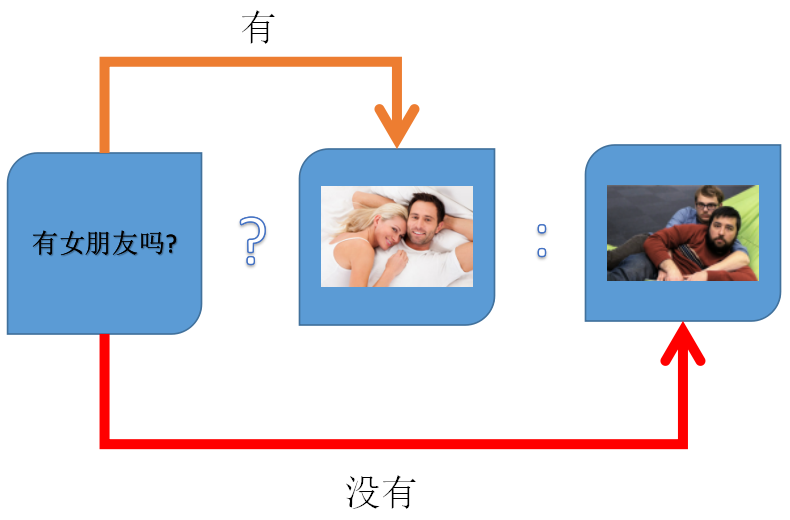• 求值规则:
• 如果"表达式1"为真，三目运算符的运算结果为"表达式2"的值(结果A)，否则为"表达式3"的值(结果B)
``````示例:
int a = 10;
int b = 20;
int max = (a > b) ? a : b;
printf("max = %d", max);
输出结果: 20

int a = 10;
int b = 20;
int max = 0;
if(a>b){
max=a;
}else {
max=b;
}
printf("max = %d", max);
``````
• 注意点
• 条件运算符的运算优先级低于关系运算符和算术运算符,但高于赋值符
• 条件运算符?和:是一个整体,不能分开使用
``````#include <stdio.h>
int main(){
int a = 10;
int b = 5;
// 先计算 a > b
// 然后再根据计算结果判定返回a还是b
// 相当于int max= (a>b) ? a : b;
int max= a>b ? a : b;
printf("max = %i\n", max); // 10
}
``````
``````#include <stdio.h>
int main(){
int a = 10;
int b = 5;
int c = 20;
int d = 10;
// 结合性是从右至左, 所以会先计算:后面的内容
// int res = a>b?a:(c>d?c:d);
// int res = a>b?a:(20>10?20:10);
// int res = a>b?a:(20);
// 然后再计算最终的结果
// int res = 10>5?10:(20);
// int res = 10;
int res = a>b?a:c>d?c:d;
printf("res = %i\n", res);
}
``````

## 类型转换

(需要转换的类型)(表达式) 1.算数转换 2.赋值转换
• 强制类型转换(显示转换)
``````// 将double转换为int
int a = (int)10.5;
``````
• 算数转换
• 系统会自动对占用内存较少的类型做一个“自动类型提升”的操作, 先将其转换为当前算数表达式中占用内存高的类型, 然后再参与运算
``````// 当前表达式用1.0占用8个字节, 2占用4个字节
// 所以会先将整数类型2转换为double类型之后再计算
double b = 1.0 / 2;
``````
• 赋值转换
``````// 赋值时左边是什么类型,就会自动将右边转换为什么类型再保存
int a = 10.6;
``````
• 注意点:
• 参与计算的是什么类型, 结果就是什么类型
``````// 结果为0, 因为参与运算的都是整型
double a = (double)(1 / 2);
// 结果为0.5, 因为1被强制转换为了double类型, 2也会被自动提升为double类型
double b = (double)1 / 2;
``````
• 类型转换并不会影响到原有变量的值
``````#include <stdio.h>
int main(){
double d = 3.14;
int num = (int)d;
printf("num = %i\n", num); // 3
printf("d = %lf\n", d); // 3.140000
}
``````

## 阶段练习

• 从键盘输入一个整数, 判断这个数是否是100到200之间的数
• 表达式 6＝＝6＝＝6 的值是多少？
• 用户从键盘上输入三个整数,找出最大值,然后输入最大值
• 用两种方式交换两个变量的保存的值
``````#include <stdio.h>
int main(){
double d = 3.14;
int num = (int)d;
printf("num = %i\n", num); // 3
printf("d = %lf\n", d); // 3.140000
}
``````

## 流程控制基本概念

• 默认情况下程序运行后，系统会按书写顺序从上至下依次执行程序中的每一行代码。但是这并不能满足我们所有的开发需求, 为了方便我们控制程序的运行流程，C语言提供3种流程控制结构，不同的流程控制结构可以实现不同的运行流程。
• 这3种流程结构分别是顺序结构、选择结构、循环结构
• 顺序结构:
• 按书写顺序从上至下依次执行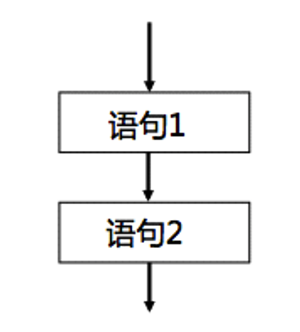• 选择结构
• 对给定的条件进行判断，再根据判断结果来决定执行代码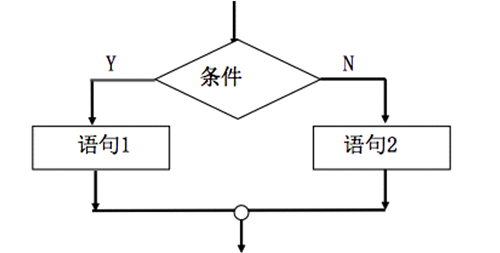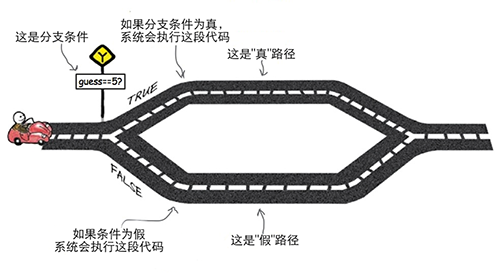• 循环结构
• 在给定条件成立的情况下，反复执行某一段代码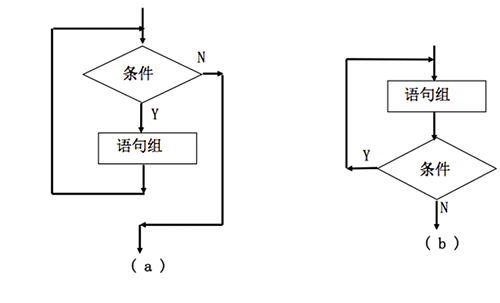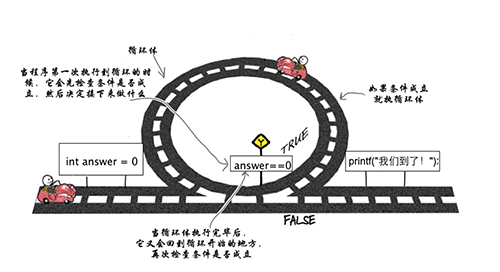## 选择结构

• C语言中提供了两大选择结构, 分别是if和switch
##选择结构if
• if第一种形式
• 表示如果表达式为真,执行语句块1,否则不执行
``````if(表达式) {
语句块1;
}

``````
``````if(age >= 18) {
printf("结婚\n");
}
printf("谈恋爱\n");
``````
• if第二种形式
• 如果表达式为真,则执行语句块1,否则执行语句块2
• else不能脱离if单独使用
``````if(表达式){
语句块1;
}else{
语句块2;
}

``````
``````if(age > 18){
printf("开网卡\n");
}else{
printf("喊家长来开\n");
}
printf("买烟\n");``````
• if第三种形式
• 如果"表达式1"为真,则执行"语句块1",否则判断"表达式2",如果为真执行"语句块2",否则再判断"表达式3",如果真执行"语句块3", 当表达式1、2、3都不满足,会执行最后一个else语句
• 众多大括号中,只有一个大括号中的内容会被执行
• 只有前面所有添加都不满足, 才会执行else大括号中的内容
``````if(表达式1) {
语句块1;
}else if(表达式2){
语句块2;
}else if(表达式3){
语句块3;
}else{
语句块4;
}

``````
``````if(age>40){
printf("给房卡");
}else if(age>25){
printf("给名片");
}else if(age>18){
printf("给网卡");
}else{
printf("给好人卡");
}
printf("买烟\n");
``````
• if嵌套
• if中可以继续嵌套if, else中也可以继续嵌套if
``````if(表达式1){
语句块1;
if(表达式2){
语句块2;
}
}else{
if(表达式3){
语句块3;
}else{
语句块4;
}
}
``````
• if注意点
• 任何数值都有真假性
``````#include <stdio.h>
int main(){
if(0){
printf("执行了if");
}else{
printf("执行了else"); // 被执行
}
}
``````
• 当if else后面只有一条语句时, if else后面的大括号可以省略
``````// 极其不推荐写法
int age = 17;
if (age >= 18)
printf("开网卡\n");
else
printf("喊家长来开\n");
``````
• 当if else后面的大括号被省略时, else会自动和距离最近的一个if匹配
``````#include <stdio.h>
int main(){
if(0)
if(1)
printf("A\n");
else // 和if(1)匹配
printf("B\n");
else // 和if(0)匹配, 因为if(1)已经被匹配过了
if (1)
printf("C\n"); // 输出C
else // 和if(1)匹配
printf("D\n");
}
``````
• 如果if else省略了大括号, 那么后面不能定义变量
``````#include <stdio.h>
int main(){
if(1)
int number = 10; // 系统会报错
printf("number = %i\n", number);
}
``````
``````#include <stdio.h>
int main(){
if(0){
int number = 10;
}else
int value = 20; // 系统会报错
printf("value = %i\n", value);
}
``````
• C语言中分号(;)也是一条语句, 称之为空语句
``````// 因为if(10 > 2)后面有一个分号, 所以系统会认为if省略了大括号
// if省略大括号时只能管控紧随其后的那条语句, 所以只能管控分号
if(10 > 2);
{
printf("10 > 2");
}
// 输出结果: 10 > 2
``````
• 但凡遇到比较一个变量等于或者不等于某一个常量的时候，把常量写在前面
``````#include <stdio.h>
int main(){
int a = 8;
//    if(a = 10){// 错误写法, 但不会报错
if (10 == a){
printf("a的值是10\n");
}else{
printf("a的值不是10\n");
}
}
``````
• if练习
• 从键盘输入一个整数，判断其是否是偶数，如果是偶数就输出YES，否则输出NO；
• 接收用户输入的1～7的整数，根据用户输入的整数，输出对应的星期几
• 接收用户输入的一个整数month代表月份，根据月份输出对应的季节
• 接收用户输入的两个整数，判断大小后输出较大的那个数
• 接收用户输入的三个整数，判断大小后输出较大的那个数
• 接收用户输入的三个整数，排序后输出
• 实现石头剪刀布
``````剪刀石头布游戏:
1)定义游戏规则
剪刀 干掉 布
石头 干掉 剪刀
布 干掉石头
2)显示玩家开始猜拳
3)接收玩家输入的内容
4)让电脑随机产生一种拳
5)判断比较
(1)玩家赢的情况(显示玩家赢了)
(2)电脑赢的情况(显示电脑赢了)
(3)平局(显示平局)
``````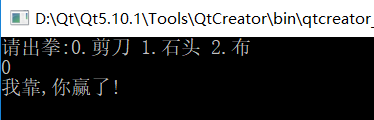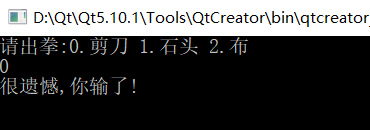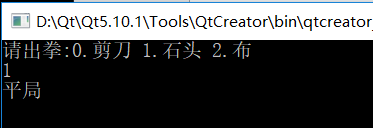## 选择结构switch

• 由于 if else if 还是不够简洁，所以switch 就应运而生了，他跟 if else if 互为补充关系。switch 提供了点的多路选择
• 格式:
``````switch(表达式){
case 常量表达式1:
语句1;
break;
case 常量表达式2:
语句2;
break;
case 常量表达式n:
语句n;
break;
default:
语句n+1;
break;
}
``````
• 语义:
• 计算"表达式"的值, 逐个与其后的"常量表达式"值相比较,当"表达式"的值与某个"常量表达式"的值相等时, 即执行其后的语句, 然后跳出switch语句
• 如果"表达式"的值与所有case后的"常量表达式"均不相同时,则执行default后的语句
• 示例:
``````#include <stdio.h>

int main() {

int num = 3;
switch(num){
case 1:
printf("星期一\n");
break;
case 2:
printf("星期二\n");
break;
case 3:
printf("星期三\n");
break;
case 4:
printf("星期四\n");
break;
case 5:
printf("星期五\n");
break;
case 6:
printf("星期六\n");
break;
case 7:
printf("星期日\n");
break;
default:
printf("回火星去\n");
break;
}
}
``````
• switch注意点
• switch条件表达式的类型必须是整型, 或者可以被提升为整型的值(char、short)
``````#include <stdio.h>

int main() {

switch(1.1){ // 报错
case 1:
printf("星期一\n");
break;
case 2:
printf("星期二\n");
break;
default:
printf("回火星去\n");
break;
}
}
``````
• +case的值只能是常量, 并且还必须是整型, 或者可以被提升为整型的值(char、short)
``````#include <stdio.h>

int main() {

int num = 3;
switch(1){
case 1:
printf("星期一\n");
break;
case 'a':
printf("星期二\n");
break;
case num: // 报错
printf("星期三\n");
break;
case 4.0: // 报错
printf("星期四\n");
break;
default:
printf("回火星去\n");
break;
}
}
``````
• case后面常量表达式的值不能相同
``````#include <stdio.h>

int main() {
switch(1){
case 1: // 报错
printf("星期一\n");
break;
case 1: // 报错
printf("星期一\n");
break;
default:
printf("回火星去\n");
break;
}
}
``````
• case后面要想定义变量,必须给case加上大括号
``````#include <stdio.h>

int main() {
switch(1){
case 1:{
int num = 10;
printf("num = %i\n", num);
printf("星期一\n");
break;
}
case 2:
printf("星期一\n");
break;
default:
printf("回火星去\n");
break;
}
}
``````
• switch中只要任意一个case匹配, 其它所有的case和default都会失效. 所以如果case和default后面没有break就会出现穿透问题
``````#include <stdio.h>

int main() {

int num = 2;
switch(num){
case 1:
printf("星期一\n");
break;
case 2:
printf("星期二\n"); // 被输出
case 3:
printf("星期三\n"); // 被输出
default:
printf("回火星去\n"); // 被输出
break;
}
}
``````
• switch中default可以省略
``````#include <stdio.h>

int main() {
switch(1){
case 1:
printf("星期一\n");
break;
case 2:
printf("星期一\n");
break;
}
}
``````
• switch中default的位置不一定要写到最后, 无论放到哪都会等到所有case都不匹配才会执行(穿透问题除外)
``````#include <stdio.h>

int main() {
switch(3){
case 1:
printf("星期一\n");
break;
default:
printf("Other,,,\n");
break;
case 2:
printf("星期一\n");
break;
}
}
``````
• if和Switch转换
• 看上去if和switch都可以实现同样的功能, 那么在企业开发中我们什么时候使用if, 什么时候使用switch呢?
• if else if 针对于范围的多路选择
• switch 是针对点的多路选择
• 判断用户输入的数据是否大于100
``````#include <stdio.h>

int main() {
int a = -1;
scanf("%d", &a);
if(a > 100){
printf("用户输入的数据大于100");
}else{
printf("用户输入的数据不大于100");
}
}
``````
``````#include <stdio.h>

int main() {
int a = -1;
scanf("%d", &a);
// 挺(T)萌(M)的(D)搞不定啊
switch (a) {
case 101:
case 102:
case 103:
case 104:
case 105:
printf("大于\n");
break;
default:
printf("不大于\n");
break;
}
}
``````
• 练习
• 实现分数等级判定
``````要求用户输入一个分数，根据输入的分数输出对应的等级
A 90～100
B 80～89
C 70～79
D 60～69
E 0～59
``````
• 实现+ - * / 简单计算器

## 循环结构

• C语言中提供了三大循环结构, 分别是while、dowhile和for
• 循环结构是程序中一种很重要的结构。
• 其特点是,在给定条件成立时,反复执行某程序段, 直到条件不成立为止。
• 给定的条件称为"循环条件",反复执行的程序段称为"循环体"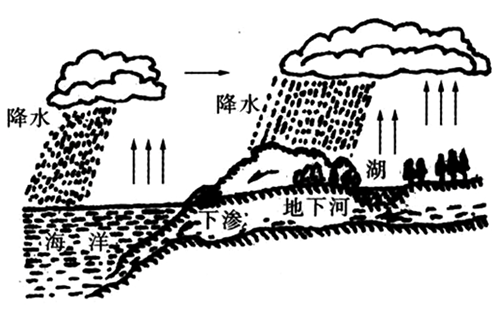## 循环结构while

• 格式:

``````while (  循环控制条件 ) {
循环体中的语句;
能够让循环结束的语句;
....
}
``````
• 构成循环结构的几个条件
• 循环控制条件
• 循环退出的主要依据,来控制循环到底什么时候退出
• 循环体
• 循环的过程中重复执行的代码段
• 能够让循环结束的语句(递增、递减、真、假等)
• 能够让循环条件为假的依据,否则退出循环
• 示例:
``````int count = 0;
while (count < 3) { // 循环控制条件
printf("发射子弹~哔哔哔哔\n"); // 需要反复执行的语句
count++; // 能够让循环结束的语句
}
``````
• while循环执行流程
• 首先会判定"循环控制条件"是否为真, 如果为假直接跳到循环语句后面
• 如果"循环控制条件"为真, 执行一次循环体, 然后再次判断"循环控制条件"是否为真, 为真继续执行循环体,为假跳出循环
• 重复以上操作, 直到"循环控制条件"为假为止
``````#include <stdio.h>
int main(){
int count = 4;
// 1.判断循环控制条件是否为真,此时为假所以跳过循环语句
while (count < 3) {
printf("发射子弹~哔哔哔哔\n");
count++;
}
// 2.执行循环语句后面的代码, 打印"循环执行完毕"
printf("循环执行完毕\n");
}
``````
``````#include <stdio.h>
int main(){
int count = 0;
// 1.判断循环控制条件是否为真,此时0 < 3为真
// 4.再次判断循环控制条件是否为真,此时1 < 3为真
// 7.再次判断循环控制条件是否为真,此时2 < 3为真
// 10.再次判断循环控制条件是否为真,此时3 < 3为假, 跳过循环语句
while (count < 3) {
// 2.执行循环体中的代码, 打印"发子弹"
// 5.执行循环体中的代码, 打印"发子弹"
// 8.执行循环体中的代码, 打印"发子弹"
printf("发射子弹~哔哔哔哔\n");
// 3.执行"能够让循环结束的语句" count = 1
// 6.执行"能够让循环结束的语句" count = 2
// 9.执行"能够让循环结束的语句" count = 3
count++;
}
// 11.执行循环语句后面的代码, 打印"循环执行完毕"
printf("循环执行完毕\n");
}
``````
• while循环注意点
• 任何数值都有真假性
``````#include <stdio.h>
int main(){
while (1) { // 死循环
printf("发射子弹~哔哔哔哔\n");
// 没有能够让循环结束的语句
}
}
``````
• 当while后面只有一条语句时,while后面的大括号可以省略
``````#include <stdio.h>
int main(){
while (1)  // 死循环
printf("发射子弹~哔哔哔哔\n");
// 没有能够让循环结束的语句
}
``````
• 如果while省略了大括号, 那么后面不能定义变量
``````#include <stdio.h>
int main(){
while (1)  // 死循环
int num = 10; // 报错
// 没有能够让循环结束的语句
}
``````
• C语言中分号(;)也是一条语句, 称之为空语句
``````#include <stdio.h>
int main(){
int count = 0;
while (count < 3);{ // 死循环
printf("发射子弹~哔哔哔哔\n");
count++;
}
}
``````
• 最简单的死循环
``````// 死循环一般在操作系统级别的应用程序会比较多, 日常开发中很少用
while (1);
``````
• while练习
• 计算1 + 2 + 3 + …n的和
• 获取1～100之间 7的倍数的个数

## 循环结构do while

• 格式:
``````do {
循环体中的语句;
能够让循环结束的语句;
....
} while (循环控制条件 );
``````
• 示例
``````int count = 0;
do {
printf("发射子弹~哔哔哔哔\n");
count++;
}while(count < 10);
``````
• do-while循环执行流程
• 首先不管while中的条件是否成立, 都会执行一次"循环体"
• 执行完一次循环体,接着再次判断while中的条件是否为真, 为真继续执行循环体,为假跳出循环
• 重复以上操作, 直到"循环控制条件"为假为止
• 应用场景
• 口令校验
``````#include<stdio.h>
int main()
{
int num = -1;
do{
printf("请输入密码,验证您的身份\n");
scanf("%d", &num);
}while(123456 != num);
printf("主人,您终于回来了\n");
}
``````
• while和dowhile应用场景
• 绝大多数情况下while和dowhile可以互换, 所以能用while就用while
• 无论如何都需要先执行一次循环体的情况, 才使用dowhile
• do while 曾一度提议废除，但是他在输入性检查方面还是有点用的

## 循环结构for

• 格式:
``````for(初始化表达式；循环条件表达式；循环后的操作表达式) {
循环体中的语句;
}
``````
• 示例

``````for(int i = 0; i < 10; i++){
printf("发射子弹~哔哔哔哔\n");
}
``````
• for循环执行流程
• 首先执行"初始化表达式"，而且在整个循环过程中,只会执行一次初始化表达式
• 接着判断"循环条件表达式"是否为真，为真执行循环体中的语句
• 循环体执行完毕后，接下来会执行"循环后的操作表达式"，然后再次判断条件是否为真,为真继续执行循环体,为假跳出循环
• 重复上述过程，直到条件不成立就结束for循环
• for循环注意点:
• 和while一模一样
• 最简单的死循环`for(;;);`
• for和while应用场景
• while能做的for都能做, 所以企业开发中能用for就用for, 因为for更为灵活
• 而且对比while来说for更节约内存空间
``````int count = 0; // 初始化表达式
while (count < 10) { // 条件表达式
printf("发射子弹~哔哔哔哔 %i\n", count);
count++; // 循环后增量表达式
}
// 如果初始化表达式的值, 需要在循环之后使用, 那么就用while
printf("count = %i\n", count);
``````
``````// 注意: 在for循环初始化表达式中定义的变量, 只能在for循环后面的{}中访问
// 所以: 如果初始化表达式的值, 不需要在循环之后使用, 那么就用for
// 因为如果初始化表达式的值, 在循环之后就不需要使用了 , 那么用while会导致性能问题
for (int count = 0; count < 10; count++) {
printf("发射子弹~哔哔哔哔 %i\n", count);
}
//     printf("count = %i\n", count);
``````
``````// 如果需要使用初始化表达式的值, 也可以将初始化表达式写到外面
int count = 0;
for (; count < 10; count++) {
printf("发射子弹~哔哔哔哔\n", count);
}
printf("count = %i\n", count);
``````

## 四大跳转

• C语言中提供了四大跳转语句, 分别是return、break、continue、goto
• break:
• 立即跳出switch语句或循环
• 应用场景:
• switch
• 循环结构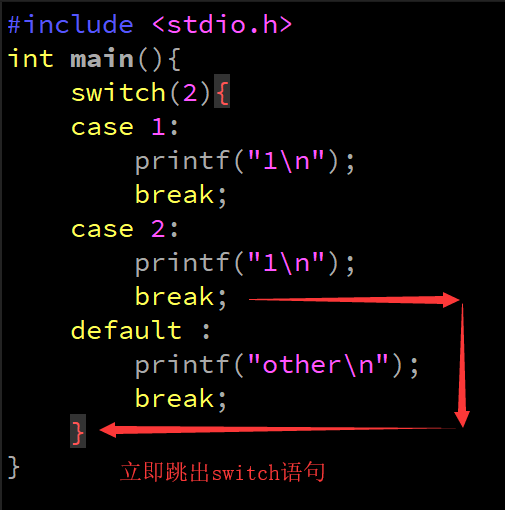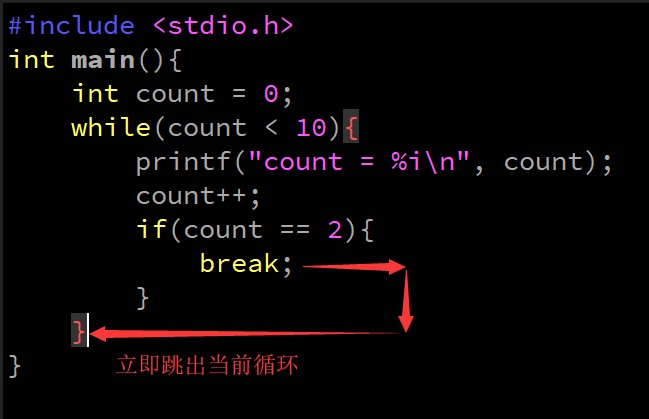• break注意点:
• break离开应用范围，存在是没有意义的
``````if(1) {
break; // 会报错
}
``````
• 在多层循环中,一个break语句只向外跳一层
``````while(1) {
while(2) {
break;// 只对while2有效, 不会影响while1
}
printf("while1循环体\n");
}
``````
• break下面不可以有语句，因为执行不到
``````while(2){
break;
printf("打我啊!");// 执行不到
}
``````
• continue
• 结束本轮循环，进入下一轮循环
• 应用场景:
• 循环结构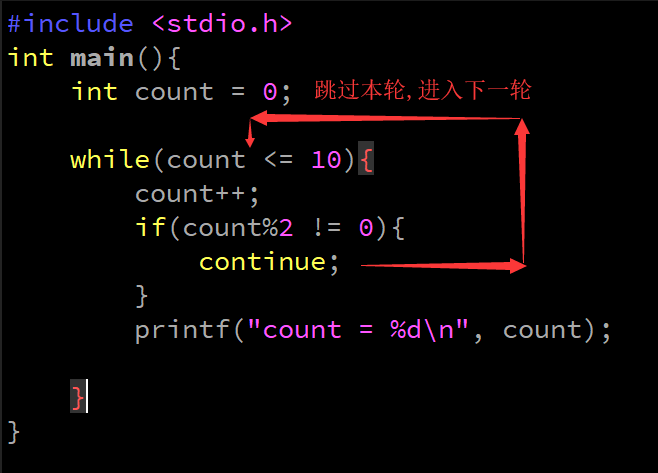• continue注意点:
• continue离开应用范围，存在是没有意义的
``````if(1) {
continue; // 会报错
}
``````

goto

• 这是一个不太值得探讨的话题，goto 会破坏结构化程序设计流程，它将使程序层次不清，且不易读，所以慎用
• goto 语句，仅能在本函数内实现跳转，不能实现跨函数跳转(短跳转)。但是他在跳出多重循环的时候效率还是蛮高的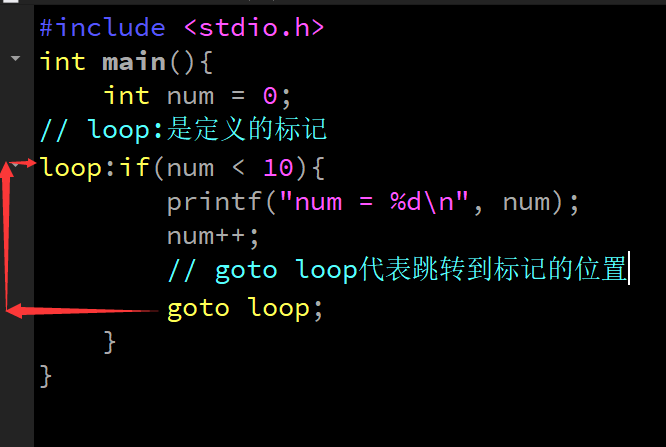``````#include <stdio.h>
int main(){
int num = 0;
// loop:是定义的标记
loop:if(num < 10){
printf("num = %d\n", num);
num++;
// goto loop代表跳转到标记的位置
goto loop;
}
}
``````
``````#include <stdio.h>
int main(){
while (1) {
while(2){
goto lnj;
}
}
lnj:printf("跳过了所有循环");
}
``````
• return
• 结束当前函数，将结果返回给调用者
• 不着急, 放一放,学到函数我们再回头来看它

## 循环的嵌套

• 循环结构的循环体中存在其他的循环结构，我们称之为循环嵌套
• 注意: 一般循环嵌套不超过三层
• 外循环执行的次数 * 内循环执行的次数就是内循环总共执行的次数
• 格式:
``````while(条件表达式) {
while循环结构 or dowhile循环结构 or for循环结构
}
``````
``````for(初始化表达式；循环条件表达式；循环后的操作表达式) {
while循环结构 or dowhile循环结构 or for循环结构
}
``````
``````do {
while循环结构 or dowhile循环结构 or for循环结构
} while (循环控制条件 );
``````
• 循环优化
• 在多重循环中，如果有可能，应当将最长的循环放在最内层，最短的循环放在最外层，以减少 CPU 跨切循环层的次数
``````for (row=0; row<100; row++) {
// 低效率：长循环在最外层
for ( col=0; col<5; col++ ) {
sum = sum + a[row][col];
}
}
``````
``````for (col=0; col<5; col++ ) {
// 高效率：长循环在最内层
for (row=0; row<100; row++) {
sum = sum + a[row][col];
}
}
``````
• 练习
• 打印好友列表
``````好友列表1
好友1
好友2

好友1
好友2

好友1
好友2
``````
``````for (int i = 0; i < 4; i++) {
printf("好友列表%d\n", i+1);
for (int j = 0; j < 4; j++) {
printf("    角色%d\n", j);
}
}
``````

## 图形打印

• 一重循环解决线性的问题，而二重循环和三重循环就可以解决平面和立体的问题了
• 打印矩形
``````****
****
****
``````
``````// 3行4列
//  外循环控制行数
for (int i = 0; i < 3; i++) {
//        内循环控制列数
for (int j = 0; j < 4; j++) {
printf("*");
}
printf("\n");
}
``````
• 打印三角形
• 尖尖朝上，改变内循环的条件表达式，让内循环的条件表达式随着外循环的i值变化
• 尖尖朝下，改变内循环的初始化表达式，让内循环的初始化表达式随着外循环的i值变化
``````*
**
***
****
*****
``````
``````/*

*/
for(int i = 0; i< 5; i++) {
for(int j = 0; j <= i; j++) {
printf("*");
}
printf("\n");
}
``````
``````*****
****
***
**
*
``````
``````for(int i = 0; i< 5; i++) {
for(int j = i; j < 5; j++) {
printf("*");
}
printf("\n");
}
``````
• 练习
• 打印特殊三角形
``````1
12
123
``````
``````for (int i = 0; i < 3; i++) {
for (int j = 0; j <= i; j++) {
printf("%d", j+1);
}
printf("\n");
}
``````
• 打印特殊三角形
``````1
22
333
``````
``````for (int i = 1; i <= 3; i++) {
for (int j = 1; j <= i; j++) {
printf("%d", i);
}
printf("\n");
}
``````
• 打印特殊三角形
``````--*
-***
*****
``````
``````for (int i = 0; i <= 5; i++) {
for (int j = 0; j < 5 - i; j++) {
printf("-");
}
for (int m = 0; m < 2*i+1; m++) {
printf("*");
}
printf("\n");
}
``````
• 打印99乘法表
``````1 * 1 = 1
1 * 2 = 2     2 * 2 = 4
1 * 3 = 3     2 * 3 = 6     3 * 3 = 9
``````
``````for (int i = 1; i <= 9; i++) {
for (int j = 1; j <= i; j++) {
printf("%d * %d = %d \t", j, i, (j * i));
}
printf("\n");
}
``````

## 函数基本概念

• C源程序是由函数组成的
• 例如: 我们前面学习的课程当中,通过main函数+scanf函数+printf函数+逻辑代码就可以组成一个C语言程序
• C语言不仅提供了极为丰富的库函数, 还允许用户建立自己定义的函数。用户可把自己的算法编写成一个个相对独立的函数，然后再需要的时候调用它
• 例如:你用C语言编写了一个MP3播放器程序，那么它的程序结构如下图所示
•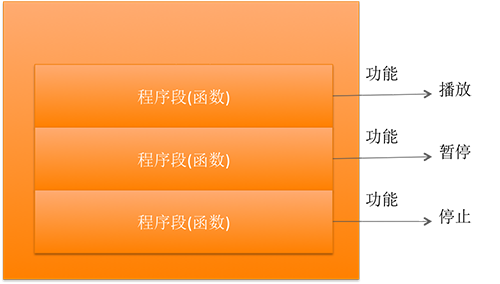• 可以说C程序的全部工作都是由各式各样的函数完成的,所以也把C语言称为函数式语言

## 函数的分类

• 在C语言中可从不同的角度对函数分类
• 从函数定义的角度看,函数可分为库函数和用户定义函数两种
• 库函数: 由C语言系统提供,用户无须定义,也不必在程序中作类型说明,只需在程序前包含有该函数原型的头文件即可在程序中直接调用。在前面各章的例题中反复用到printf、scanf、getchar、putchar等函数均属此类
• ***用户定义函数:***由用户按需编写的函数。对于用户自定义函数,不仅要在程序中定义函数本身,而且在主调函数模块中还必须对该被调函数进行类型说明,然后才能使用
• 从函数执行结果的角度来看, 函数可分为有返回值函数和无返回值函数两种
• 有返回值函数: 此类函数被调用执行完后将向调用者返回一个执行结果,称为函数返回值。(必须指定返回值类型和使用return关键字返回对应数据)
• 无返回值函数: 此类函数用于完成某项特定的处理任务,执行完成后不向调用者返回函数值。(返回值类型为void, 不用使用return关键字返回对应数据)
• 从主调函数和被调函数之间数据传送的角度看,又可分为无参函数和有参函数两种
• 无参函数: 在函数定义及函数说明及函数调用中均不带参数。主调函数和被调函数之间不进行参数传送。
• 有参函数: 在函数定义及函数说明时都有参数,称为形式参数(简称为形参)。在函数调用时也必须给出参数,称为实际参数(简称为实参)

## 函数的定义

• 定义函数的目的
• 将一个常用的功能封装起来，方便以后调用
• 自定义函数的书写格式
``````返回值类型 函数名(参数类型 形式参数1，参数类型 形式参数2，…) {
函数体;
返回值;
}
``````
• 示例
``````int main(){
printf("hello world\n");
retrun 0;
}
``````
• 定义函数的步骤
• 函数名：函数叫什么名字
• 函数体：函数是干啥的，里面包含了什么代码
• 返回值类型: 函数执行完毕返回什么和调用者

• 无参无返回值函数定义
• 没有返回值时return可以省略
• 格式:
``````void 函数名() {
函数体;
}
``````
• 示例:
``````// 1.没有返回值/没有形参
// 如果一个函数不需要返回任何数据给调用者, 那么返回值类型就是void
void printRose() {
printf(" {@}\n");
printf("  |\n");
printf(" \\|/\n"); // 注意: \是一个特殊的符号(转意字符), 想输出\必须写两个斜线
printf("  |\n");
// 如果函数不需要返回数据给调用者, 那么函数中的return可以不写
}
``````

• 格式:

``````返回值类型 函数名() {
函数体;
return 值;
}
``````
• 示例:
``````int getMax() {
printf("请输入两个整数, 以逗号隔开, 以回车结束\n");
int number1, number2;
scanf("%i,%i", &number1, &number2);
int max = number1 > number2 ? number1 : number2;
return max;
}
``````

• 形式参数表列表的格式: `类型 变量名,类型 变量2,......`
• 格式:
``````void 函数名(参数类型 形式参数1，参数类型 形式参数2，…) {
函数体;
}
``````
• 示例:
``````void printMax(int value1, int value2) {
int max = value1 > value2 ? value1 : value2;
printf("max = %i\n", max);
}
``````

• 格式:
``````返回值类型 函数名(参数类型 形式参数1，参数类型 形式参数2，…) {
函数体;
return 0;
}``````
• 示例:
`````` int printMax(int value1, int value2) {
int max = value1 > value2 ? value1 : value2;
return max;
}``````
• 函数定义注意

• 函数名称不能相同
``````void test() {
}
void test() { // 报错
}``````

## 函数的参数和返回值

• 形式参数
• 定义函数时，函数名后面小括号()中定义的变量称为形式参数，简称形参
• 形参变量只有在被调用时才分配内存单元,在调用结束时,即刻释放所分配的内存单元。
• 因此,形参只有在函数内部有效,函数调用结束返回主调函数后则不能再使用该形参变量
``````int max(int number1, int number2) //  形式参数
{
return number1 > number2 ? number1 : number2;
}``````

• 实际参数
• 调用函数时, 传入的值称为实际参数，简称实参
• 实参可以是常量、变量、表达式、函数等,无论实参是何种类型的量,在进行函数调用时,它们都必须具有确定的值,以便把这些值传送给形参
• 因此应预先用赋值,输入等办法使实参获得确定值
``````int main() {
int num = 99;
// 88, num, 22+44均能得到一个确定的值, 所以都可以作为实参
max(88, num, 22+44); // 实际参数
return 0;
}``````

• 调用函数时传递的实参个数必须和函数的形参个数必须保持一致
``````int max(int number1, int number2) { //  形式参数
return number1 > number2 ? number1 : number2;
}
int main() {
// 函数需要2个形参, 但是我们只传递了一个实参, 所以报错
max(88); // 实际参数
return 0;
}
``````
• 形参实参类型不一致, 会自动转换为形参类型
``````void change(double number1, double number2) {//  形式参数
// 输出结果: 10.000000, 20.000000
// 自动将实参转换为double类型后保存
printf("number1 = %f, number2 = %f", number1, number2);
}
int main() {
change(10, 20);
return 0;
}
``````
• 当使用基本数据类型（char、int、float等）作为实参时，实参和形参之间只是值传递，修改形参的值并不影响到实参函数可以没有形参
``````void change(int number1, int number2) { //  形式参数
number1 = 250; // 不会影响实参
number2 = 222;
}
int main() {
int a = 88;
int b = 99;
change(a, b);
printf("a  = %d, b = %d", a, b); // 输出结果: 88, 99
return 0;
}
``````

• 如果没有写返回值类型，默认是int
``````max(int number1, int number2) {//  形式参数
return number1 > number2 ? number1 : number2;
}``````
• 函数返回值的类型和return实际返回的值类型应保持一致。如果两者不一致,则以返回值类型为准,自动进行类型转换
``````int height() {
return 3.14;
}
int main() {
double temp = height();
printf("%lf", temp);// 输出结果: 3.000000
}``````
• 一个函数内部可以多次使用return语句，但是return语句后面的代码就不再被执行
``````int max(int number1, int number2) {//  形式参数
return number1 > number2 ? number1 : number2;
printf("执行不到"); // 执行不到
return 250; // 执行不到
}``````

## 函数的声明

• 在C语言中，函数的定义顺序是有讲究的：
• 默认情况下，只有后面定义的函数才可以调用前面定义过的函数
• 如果想把函数的定义写在main函数后面，而且main函数能正常调用这些函数，那就必须在main函数的前面进行函数的声明, 否则
• 系统搞不清楚有没有这个函数
• 系统搞不清楚这个函数接收几个参数
• 系统搞不清楚这个函数的返回值类型是什么
• 所以函数声明,就是在函数调用之前告诉系统, 该函数叫什么名称, 该函数接收几个参数, 该函数的返回值类型是什么
• 函数的声明格式：
• 将自定义函数时{}之前的内容拷贝到调用之间即可
• 例如: `int max( int a, int b );`
• 或者: `int max( int, int );`
``````// 函数声明
void getMax(int v1, int v2);
int main(int argc, const char * argv[]) {
getMax(10, 20); // 调用函数
return 0;
}
// 函数实现
void getMax(int v1, int v2) {
int max = v1 > v2 ? v1 : v2;
printf("max = %i\n", max);
}``````
• 函数的声明与实现的关系

• 声明仅仅代表着告诉系统一定有这个函数, 和这个函数的参数、返回值是什么
• 实现代表着告诉系统, 这个函数具体的业务逻辑是怎么运作的
• 函数声明注意点:

• 函数的实现不能重复, 而函数的声明可以重复
``````// 函数声明
void getMax(int v1, int v2);
void getMax(int v1, int v2);
void getMax(int v1, int v2); // 不会报错
int main(int argc, const char * argv[]) {
getMax(10, 20); // 调用函数
return 0;
}
// 函数实现
void getMax(int v1, int v2) {
int max = v1 > v2 ? v1 : v2;
printf("max = %i\n", max);
}``````

``````int main(int argc, const char * argv[]) {
void getMax(int v1, int v2); // 函数声明, 不会报错
getMax(10, 20); // 调用函数
return 0;
}
// 函数实现
void getMax(int v1, int v2) {
int max = v1 > v2 ? v1 : v2;
printf("max = %i\n", max);
}``````
• 当被调函数的函数定义出现在主调函数之前时,在主调函数中也可以不对被调函数再作声明
``````// 函数实现
void getMax(int v1, int v2) {
int max = v1 > v2 ? v1 : v2;
printf("max = %i\n", max);
}
int main(int argc, const char * argv[]) {
getMax(10, 20); // 调用函数
return 0;
}``````

``````int main(int argc, const char * argv[]) {
int res = getMin(5, 3); // 不会报错
printf("result = %d\n", res );
return 0;
}
int getMin(int num1, int num2) {// 返回int, 不用声明
return num1 < num2 ? num1 : num2;
}``````

## main函数分析

• main的含义:
• main是函数的名称, 和我们自定义的函数名称一样, 也是一个标识符
• 只不过main这个名称比较特殊, 程序已启动就会自动调用它
• return 0;的含义:
• 告诉系统main函数是否正确的被执行了
• 如果main函数的执行正常, 那么就返回0
• 如果main函数执行不正常, 那么就返回一个非0的数
• 返回值类型:
• 一个函数return后面写的是什么类型, 函数的返回值类型就必须是什么类型, 所以写int
• 形参列表的含义
• int argc :
• 系统在启动程序时调用main函数时传递给argv的值的个数
• const char * argv[] :
• 系统在启动程序时传入的的值, 默认情况下系统只会传入一个值, 这个值就是main函数执行文件的路径
• 也可以通过命令行或项目设置传入其它参数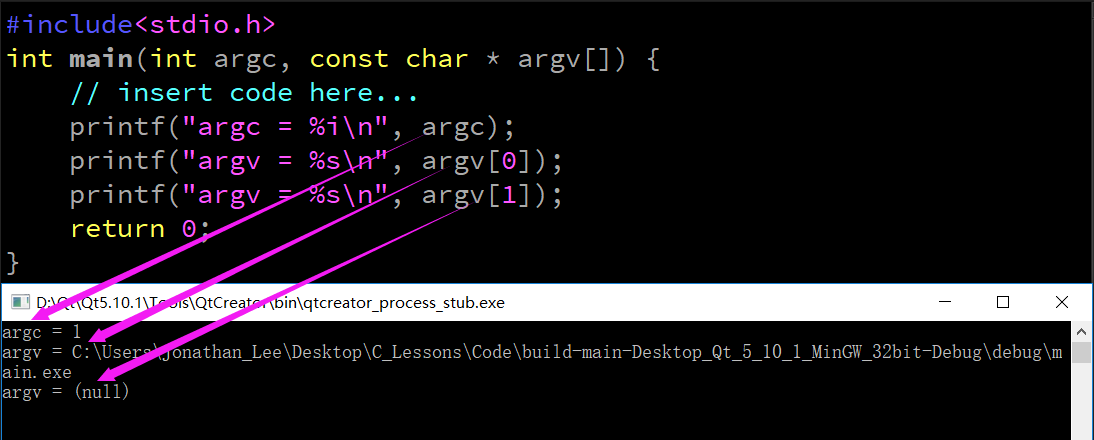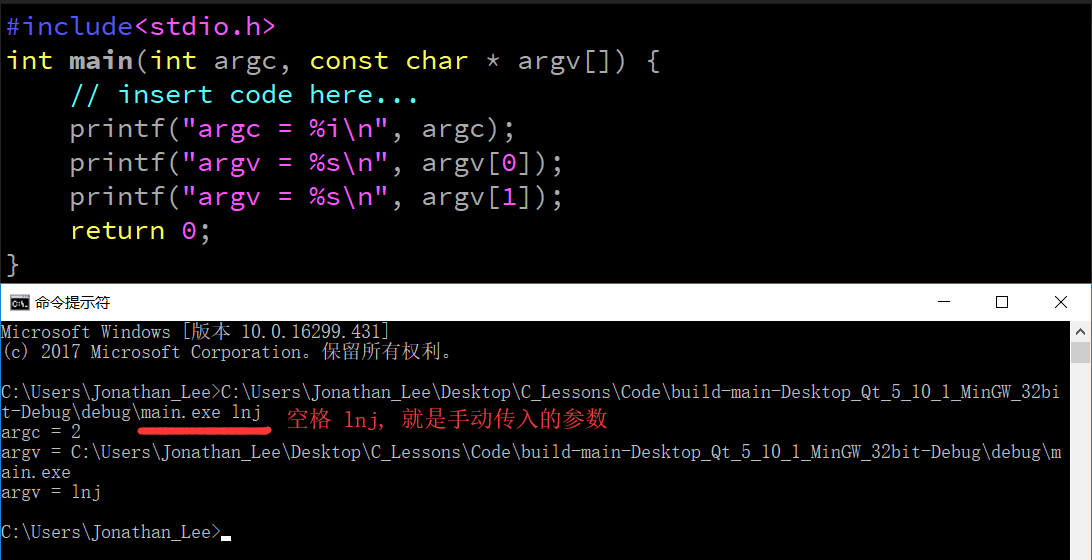• 函数练习
• 写一个函数从键盘输入三个整型数字,找出其最大值
• 写一个函数求三个数的平均值

## 递归函数(了解)

• 什么是递归函数?

• 一个函数在它的函数体内调用它自身称为递归调用
``````void function(int x){
function(x);
}``````
• 递归函数构成条件

• 自己搞自己
• 存在一个条件能够让递归结束
• 问题的规模能够缩小
• 示例:

• 获取用户输入的数字, 直到用户输入一个正数为止
``````void getNumber(){
int number = -1;
while (number < 0) {
printf("请输入一个正数\n");
scanf("%d", &number);
}

printf("number = %d\n", number);
}``````
``````void getNumber2(){
int number = -1;
printf("请输入一个正数abc\n");
scanf("%d", &number);
if (number < 0) {
//        负数
getNumber2();
}else{
//        正数
printf("number = %d\n", number);
}
}
``````
• 递归和循环区别
• 能用循环实现的功能,用递归都可以实现
• 递归常用于"回溯", “树的遍历”,"图的搜索"等问题
• 但代码理解难度大，内存消耗大(易导致栈溢出), 所以考虑到代码理解难度和内存消耗问题, 在企业开发中一般能用循环都不会使用递归
• 递归练习
• 有5个人坐在一起,问第5个人多少岁?他说比第4个人大两岁。问 第4个人岁数,他说比第3个人大两岁。问第3个人,又说比第2个 人大两岁。问第2个人,说比第1个人大两岁。最后问第1个人, 他说是10岁。请问第5个人多大?
• 用递归法求N的阶乘
• 设计一个函数用来计算B的n次方

## 进制基本概念

• 什么是进制?

• 进制是一种计数的方式,数值的表示形式
• 常见的进制

• 十进制、二进制、八进制、十六进制
• 进制书写的格式和规律

• 十进制 0、1、2、3、4、5、6、7、8、9 逢十进一

• 二进制 0、1 逢二进一

• 书写形式:需要以0b或者0B开头,例如: 0b101
• 八进制 0、1、2、3、4、5、6、7 逢八进一

• 书写形式:在前面加个0,例如: 061
• 十六进制 0、1、2、3、4、5、6、7、8、9、A、B、C、D、E、F 逢十六进一

• 书写形式:在前面加个0x或者0X,例如: 0x45

• 练习

• 1.用不同进制表示如下有多少个方格
•• 2.判断下列数字是否合理
``````00011  0x001  0x7h4  10.98  0986  .089-109
+178  0b325  0b0010  0xffdc 96f 96.0f 96.oF  -.003``````

## 进制转换

• 10 进制转 2 进制
• 除2取余, 余数倒序; 得到的序列就是二进制表示形式
• 例如: 将十进制(97) 10转换为二进制数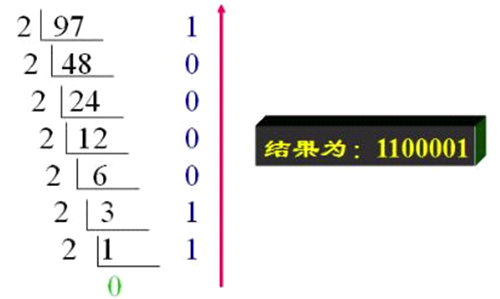• 2 进制转 10 进制

• 每一位二进制进制位的值 * 2的当前索引次幂; 再将所有位求出的值相加
• 例如: 将二进制01100100转换为十进制
``````01100100
索引从右至左, 从零开始
第0位: 0 * 2^0 = 0;
第1位: 0 * 2^1 = 0;
第2位: 1 * 2^2 = 4;
第3位: 0 * 2^3 = 0;
第4位: 0 * 2^4 = 0;
第5位: 1 * 2^5 = 32;
第6位: 1 * 2^6 = 64;
第7位: 0 * 2^7 = 0;
最终结果为: 0 + 0 + 4 + 0 + 0 + 32 + 64 + 0 = 100``````

2 进制转 8 进制

• 三个二进制位代表一个八进制位, 因为3个二进制位的最大值是7，而八进制是逢8进1
• 例如: 将二进制01100100转换为八进制数
``````从右至左每3位划分为8进制的1位, 不够前面补0
001 100 100

2 进制转 16 进制

• 四个二进制位代表一个十六进制位，因为4个二进制位的最大值是15，而十六进制是逢16进1
• 例如: 将二进制01100100转换为十六进制数
``````从右至左每4位划分为16进制的1位, 不够前面补0
0110 0100

• 系数 * 基数 ^ 索引 之和
`````` 十进制           -->          十进制
12345   =  10000 + 2000 + 300 + 40 + 5
=  (1 * 10 ^ 4)  + (2 * 10 ^ 3) + (3 * 10 ^ 2) + (4 * 10 ^ 1) + (5 * 10 ^ 0)
=  (1 * 10000) + (2 + 1000) + (3 * 100) + (4 * 10) + (5 * 1)
=  10000 + 2000 + 300 + 40 + 5
=  12345

``````二进制        -->      十进制
543210
101101 = (1 * 2 ^ 5) + (0 * 2 ^ 4) + (1 * 2 ^ 3) + (1 * 2 ^ 2) + (0 * 2 ^ 1) + (1 * 2 ^ 0)
= 32 + 0 + 8 + 4 + 0 + 1
= 45

016  =   (0 * 8 ^ 2) + (1 * 8 ^ 1) + (6 * 8 ^ 0)
=    0  + 8 + 6
=    14

0x11f =  (1 * 16 ^ 2) + (1 * 16 ^ 1) + (15 * 16 ^ 0)
=   256  + 16 + 15
=   287
``````

• 十进制除以`基数`取余, 倒叙读取
``````十进制        -->     二进制
100          -->    1100100
100 / 2   = 50     0
50  / 2   = 25     0
25  / 2   = 12     1
12  / 2   = 6      0
6   / 2   = 3      0
3   / 2   = 1      1
1   / 2   = 0      1

100          -->     144
100 / 8    = 12    4
12  / 8    = 1     4
1   / 8    = 0     1

100          --> 64
100 / 16   =  6    4
6   / 16   =  0    6``````

## 十进制小数转换为二进制小数

• 整数部分,直接转换为二进制即可
• 小数部分,使用"乘2取整，顺序排列"
• 用2乘十进制小数,可以得到积,将积的整数部分取出,再用2乘余下的小数部分,直到积中的小数部分为零，或者达到所要求的精度为止
• 然后把取出的整数部分按顺序排列起来, 即是小数部分二进制
• 最后将整数部分的二进制和小数部分的二进制合并起来, 即是一个二进制小数
• 例如: 将12.125转换为二进制
``````// 整数部分(除2取余)
12
/  2
------
6    // 余0
/  2
------
3    // 余0
/  2
------
1   // 余1
/  2
------
0   // 余1
//12 --> 1100

// 小数部分(乘2取整数积)
0.125
*     2
------
0.25  //0
0.25
*     2
------
0.5  //0
0.5
*     2
------
1.0  //1
0.0
// 0.125 --> 0.001

// 12.8125 --> 1100.001
``````

## 二进制小数转换为十进制小数

• 整数部分按照二进制转十进制即可
• 小数部分从最高位开始乘以2的负n次方, n从1开始
• 例如: 将 1100.001转换为十进制
``````// 整数部分(乘以2的n次方, n从0开始)
0 * 2^0 = 0
0 * 2^1 = 0
1 * 2^2 = 4
1 * 2^3 = 8
// 1100 == 8 + 4 + 0 + 0 == 12

// 小数部分(乘以2的负n次方, n从0开始)
0 * (1/2) = 0
0 * (1/4) = 0
1 * (1/8) = 0.125
// .100 == 0 + 0 + 0.125 == 0.125

// 1100.001  --> 12.125``````
• 练习:
• 将0.8125转换为二进制
• 将0.1101转换为十进制
``````  0.8125
*      2
--------
1.625  // 1
0.625
*      2
--------
1.25 // 1
0.25
*      2
--------
0.5 // 0
*      2
--------
1.0 // 1
0.0

// 0. 8125  --> 0.1101``````
``````1*(1/2) = 0.5
1*(1/4)=0.25
0*(1/8)=0
1*(1/16)=0.0625

//0.1101 --> 0.5 + 0.25 + 0 + 0.0625 == 0.8125
``````

## 原码反码补码

• 计算机只能识别0和1, 所以计算机中存储的数据都是以0和1的形式存储的

• 数据在计算机内部是以补码的形式储存的, 所有数据的运算都是以补码进行的

• 正数的原码、反码和补码

• 正数的原码、反码和补码都是它的二进制
• 例如: 12的原码、反码和补码分别为
• `0000 0000 0000 0000 0000 0000 0000 1100`
• `0000 0000 0000 0000 0000 0000 0000 1100`
• `0000 0000 0000 0000 0000 0000 0000 1100`
• 负数的原码、反码和补码

• 二进制的最高位我们称之为符号位, 最高位是0代表是一个正数, 最高位是1代表是一个负数
• 一个负数的原码, 是将该负数的二进制最高位变为1
• 一个负数的反码, 是将该数的原码`除了符号位`以外的其它位取反
• 一个负数的补码, 就是它的反码 + 1
• 例如: -12的原码、反码和补码分别为
``````0000 0000 0000 0000 0000 0000 0000 1100 // 12二进制
1000 0000 0000 0000 0000 0000 0000 1100 // -12原码
1111 1111 1111 1111 1111 1111 1111 0011  // -12反码
1111 1111 1111 1111 1111 1111 1111 0100 // -12补码``````

• 反码 = 补码-1
• 原码= 反码最高位不变, 其它位取反
``````1111 1111 1111 1111 1111 1111 1111 0100 // -12补码
1111 1111 1111 1111 1111 1111 1111 0011  // -12反码
1000 0000 0000 0000 0000 0000 0000 1100 // -12原码``````

• 在学习本节内容之前,大家必须明白一个东西, 就是计算机只能做加法运算, 不能做减法和乘除法, 所以的减法和乘除法内部都是用加法来实现的

• 例如: 1 - 1, 内部其实就是 1 + (-1);
• 例如: 3 * 3, 内部其实就是 3 + 3 + 3;
• 例如: 9 / 3, 内部其实就是 9 + (-3) + (-3) + (-3);
• 首先我们先来观察一下,如果只有原码会存储什么问题

• 很明显, 通过我们的观察, 如果只有原码, 1-1的结果不对
``````// 1 + 1
0000 0000 0000 0000 0000 0000 0000 0001 // 1原码
+0000 0000 0000 0000 0000 0000 0000 0001 // 1原码
---------------------------------------
0000 0000 0000 0000 0000 0000 0000 0010  == 2

// 1 - 1; 1 + (-1);
0000 0000 0000 0000 0000 0000 0000 0001 // 1原码
+1000 0000 0000 0000 0000 0000 0000 0001 // -1原码
---------------------------------------
1000 0000 0000 0000 0000 0000 0000 0010 == -2``````

• 通过反码计算减法的结果, 得到的也是一个反码;
• 将计算的结果符号位不变其余位取反,就得到了计算结果的原码
• 通过对原码的转换, 很明显我们计算的结果是-0, 符合我们的预期
``````// 1 - 1; 1 + (-1);
0000 0000 0000 0000 0000 0000 0000 0001 // 1反码
1111 1111 1111 1111 1111 1111 1111 1110   // -1反码
---------------------------------------
1111 1111 1111 1111 1111 1111 1111 1111 // 计算结果反码
1000 0000 0000 0000 0000 0000 0000 0000 // 计算结果原码 == -0``````

• 由于int只能存储4个字节, 也就是32位数据, 而计算的结果又33位, 所以最高位溢出了,符号位变成了0, 所以最终得到的结果是0
`````` // 1 - 1; 1 + (-1);
0000 0000 0000 0000 0000 0000 0000 0001 // 1补码
1111 1111 1111 1111 1111 1111 1111 1111   // -1补码
---------------------------------------
10000 0000 0000 0000 0000 0000 0000 0000 // 计算结果补码
0000 0000 0000 0000 0000 0000 0000 0000 //  == 0``````

## 位运算符

• 程序中的所有数据在计算机内存中都是以二进制的形式储存的。
• 位运算就是直接对整数在内存中的二进制位进行操作
• C语言提供了6个位操作运算符, 这些运算符只能用于整型操作数

& 按位与 同1为1
| 按位或 有1为1
^ 按位异或 不同为1
~ 按位取反 0变1,1变0
<< 按位左移 乘以2的n次方
>> 按位右移 除以2的n次方
• 按位与:
• 只有对应的两个二进位均为1时，结果位才为1，否则为0
• 规律: 二进制中，与1相&就保持原位，与0相&就为0
``````9&5 = 1

1001
&0101
------
0001``````
• 按位或:
• 只要对应的二个二进位有一个为1时，结果位就为1，否则为0
``````9|5 = 13

1001
|0101
------
1101``````
• 按位异或
• 当对应的二进位相异（不相同）时，结果为1，否则为0
• 规律:
• 相同整数相的结果是0。比如55=0
• 多个整数相^的结果跟顺序无关。例如: 567=576
• 同一个数异或另外一个数两次, 结果还是那个数。例如: 577 = 5
``````9^5 = 12

1001
^0101
------
1100``````
• 按位取反
• 各二进位进行取反（0变1，1变0）
``````~9 =-10
0000 0000 0000 0000 0000 1001 // 取反前
1111 1111 1111 1111 1111 0110 // 取反后

// 根据负数补码得出结果
1111 1111 1111 1111 1111 0110 // 补码
1111 1111 1111 1111 1111 0101 // 反码
1000 0000 0000 0000 0000 1010 // 源码 == -10``````

• 判断奇偶(按位或)
`````` 偶数: 的二进制是以0结尾
8   -> 1000
10  -> 1010

奇数: 的二进制是以1结尾
9   -> 1001
11  -> 1011

任何数和1进行&操作,得到这个数的最低位
1000
&0001
-----
0000  // 结果为0, 代表是偶数

1011
&0001
-----
0001 // 结果为1, 代表是奇数``````
• 权限系统
``````  enum Unix {
S_IRUSR = 256,// 100000000 用户可读
S_IWUSR = 128,//  10000000 用户可写
S_IXUSR = 64,//    1000000 用户可执行
S_IRGRP = 32,//     100000 组可读
S_IWGRP = 16,//      10000 组可写
S_IXGRP = 8,//        1000 组可执行
S_IROTH = 4,//         100 其它可读
S_IWOTH = 2,//          10 其它可写
S_IXOTH = 1 //           1 其它可执行
};
// 假设设置用户权限为可读可写
printf("%d\n", S_IRUSR | S_IWUSR); // 384 // 110000000``````
• 交换两个数的值(按位异或)
``````a = a^b;
b = b^a;
a = a^b;``````
• 按位左移
• 把整数a的各二进位全部左移n位，高位丢弃，低位补0
• 由于左移是丢弃最高位，0补最低位，所以符号位也会被丢弃，左移出来的结果值可能会改变正负性
• 规律: 左移n位其实就是乘以2的n次方
``````2<<1; //相当于 2 *= 2 // 4
0010
<<0100

2<<2; //相当于 2 *= 2^2; // 8
0010
<<1000``````
• 按位右移
• 把整数a的各二进位全部右移n位，保持符号位不变
• 为正数时， 符号位为0，最高位补0
• 为负数时，符号位为1，最高位是补0或是补1(取决于编译系统的规定)
• 规律: 快速计算一个数除以2的n次方
``````2>>1; //相当于 2 /= 2 // 1
0010
>>0001
4>>2; //相当于 4 /= 2^2 // 1
0100
>>0001``````
• 练习:
• 写一个函数把一个10进制数按照二进制格式输出
``````#include <stdio.h>
void printBinary(int num);
int main(int argc, const char * argv[]) {
printBinary(13);
}
void printBinary(int num){
int len = sizeof(int)*8;
int temp;
for (int i=0; i<len; i++) {
temp = num; //每次都在原数的基础上进行移位运算
temp = temp>>(31-i); //每次移动的位数
int t = temp&1; //取出最后一位
if(i!=0&&i%4==0)printf(" "); printf("%d",t);
}
}``````

## 变量内存分析

• 内存模型
• 内存模型是线性的(有序的)
• 对于 32 机而言，最大的内存地址是2^32次方bit(4294967296)(4GB)
• 对于 64 机而言，最大的内存地址是2^64次方bit(18446744073709552000)(171亿GB)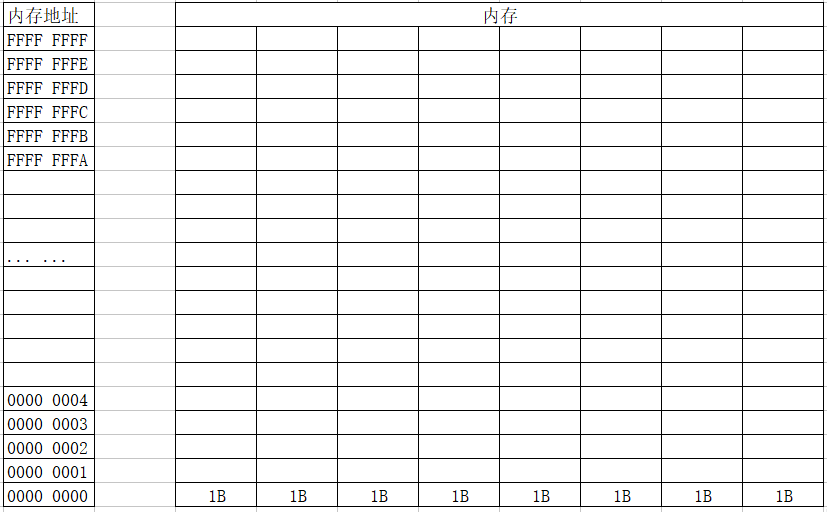• CPU 读写内存
• CPU 在运作时要明确三件事
• 存储单元的地址（地址信息）
• 器件的选择，读 or 写 （控制信息）
• 读写的数据 （数据信息）
• 如何明确这三件事情
• 通过地址总线找到存储单元的地址
• 通过控制总线发送内存读写指令
• 通过数据总线传输需要读写的数据
• `地址总线: 地址总线宽度决定了CPU可以访问的物理地址空间(寻址能力)`
• `例如: 地址总线的宽度是1位, 那么表示可以访问 0 和 1的内存`
• `例如: 地址总线的位数是2位, 那么表示可以访问 00、01、10、11的内存`
• `数据总线: 数据总线的位数决定CPU单次通信能交换的信息数量`
• `例如: 数据总线:的宽度是1位, 那么一次可以传输1位二进制数据`
• `例如: 地址总线的位数是2位,那么一次可以传输2位二进制数据`
• `控制总线: 用来传送各种控制信号`

• CPU 通过地址线将找到地址为 FFFFFFFB 的内存
• CPU 通过控制线发出内存写入命令，选中存储器芯片，并通知它，要其写入数据。
• CPU 通过数据线将数据 8 送入内存 FFFFFFFB 单元中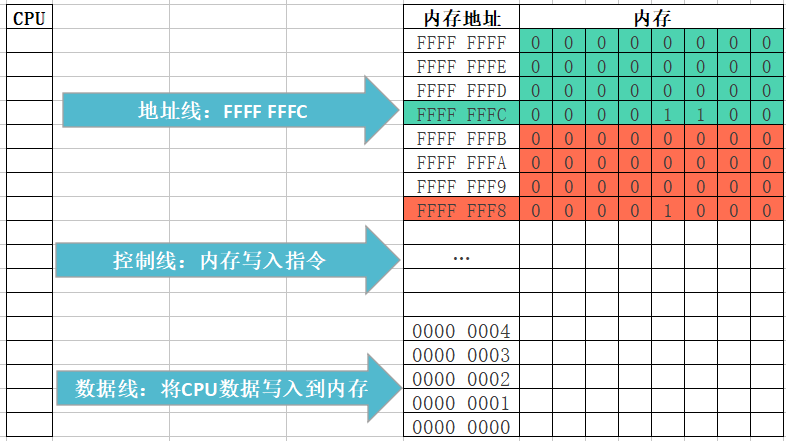• CPU 通过地址线将找到地址为 FFFFFFFB 的内存
• CPU 通过控制线发出内存读取命令，选中存储器芯片，并通知它，将要从中读取数据
• 存储器将 FFFFFFFB 号单元中的数据 8 通过数据线送入 CPU寄存器中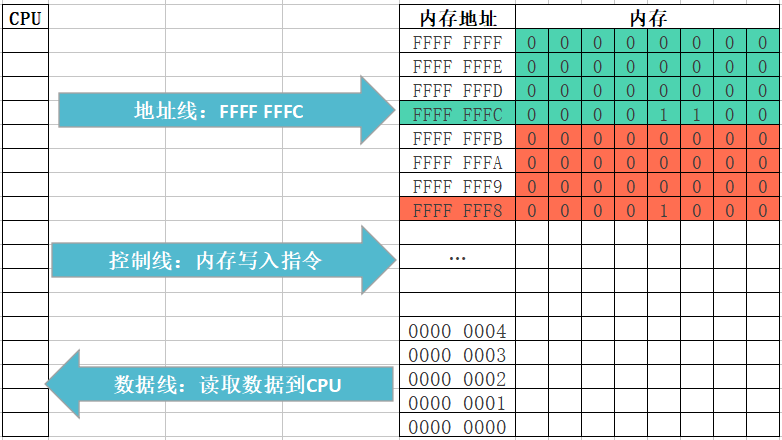• 先分配字节地址大内存,然后分配字节地址小的内存(内存寻址是由大到小)
• 变量的首地址,是变量所占存储空间字节地址(最小的那个地址 )
• 低位保存在低地址字节上,高位保存在高地址字节上
``````10的二进制: 0b00000000 00000000 00000000 00001010
高字节←                        →低字节``````

## char类型内存存储细节

• char类型基本概念
• char是C语言中比较灵活的一种数据类型，称为“字符型”
• char类型变量占1个字节存储空间，共8位
• 除单个字符以外, C语言的的转义字符也可以利用char类型存储

\b 退格(BS)当前位置向后回退一个字符
\r 回车(CR),将当前位置移至本行开头
\n 换行(LF),将当前位置移至下一行开头
\t 水平制表(HT),跳到下一个 TAB 位置
\0 用于表示字符串的结束标记
\ 代表一个反斜线字符 \
" 代表一个双引号字符"
\’ 代表一个单引号字符’

char型数据存储原理

• 计算机只能识别0和1, 所以char类型存储数据并不是存储一个字符, 而是将字符转换为0和1之后再存储
• 正是因为存储字符类型时需要将字符转换为0和1, 所以为了统一, 老美就定义了一个叫做ASCII表的东东
• ASCII表中定义了每一个字符对应的整数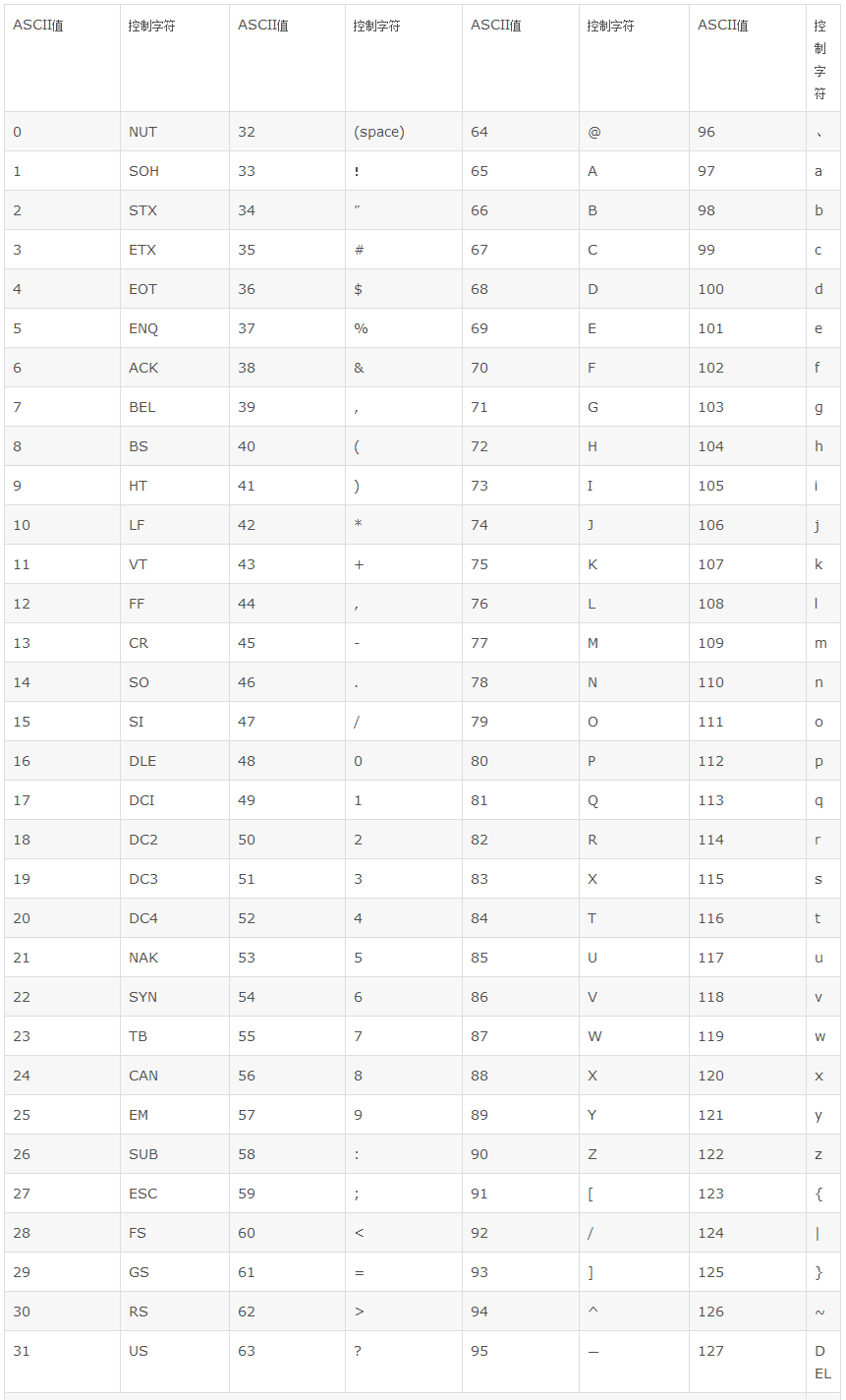``````char ch1 = 'a';
printf("%i\n", ch1); // 97

char ch2 = 97;
printf("%c\n", ch2); // a``````

char类型注意点

• char类型占一个字节, 一个中文字符占3字节(unicode表),所有char不可以存储中文
``char c = '我'; // 错误写法``
• 除转义字符以外, 不支持多个字符
``char ch = 'ab'; // 错误写法``
• char类型存储字符时会先查找对应的ASCII码值, 存储的是ASCII值, 所以字符6和数字6存储的内容不同
``````char ch1 = '6'; // 存储的是ASCII码 64
char ch2 = 6; //  存储的是数字 6``````
• 练习
• 定义一个函数, 实现输入一个小写字母,要求转换成大写输出

## 类型说明符

• 类型说明符基本概念

• C语言提供了

说明长度

说明符号位

的两种类型说明符, 这两种类型说明符一共有4个：

• short 短整型 (说明长度)
• long 长整型 (说明长度)
• signed 有符号型 (说明符号位)
• unsigned 无符号型 (说明符号位)
• 这些说明符一般都是用来修饰int类型的，所以在使用时可以省略int

• 这些说明符都属于C语言关键字

## short和long

• short和long可以提供不同长度的整型数，也就是可以改变整型数的取值范围。
• 在64bit编译器环境下，int占用4个字节（32bit），取值范围是-2^31 ~ 2^31-1；
• short占用2个字节（16bit），取值范围是-2^15 ~ 2^15-1；
• long占用8个字节（64bit），取值范围是-2^63 ~ 2^63-1
• 总结一下：在64位编译器环境下:
• short占2个字节(16位)
• int占4个字节(32位)
• long占8个字节(64位)。
• 因此，如果使用的整数不是很大的话，可以使用short代替int，这样的话，更节省内存开销。
• 世界上的编译器林林总总，不同编译器环境下，int、short、long的取值范围和占用的长度又是不一样的。比如在16bit编译器环境下，long只占用4个字节。不过幸运的是，ANSI \ ISO制定了以下规则：
• short跟int至少为16位(2字节)
• long至少为32位(4字节)
• short的长度不能大于int，int的长度不能大于long
• char一定为为8位(1字节)，毕竟char是我们编程能用的最小数据类型
• 可以连续使用2个long，也就是long long。一般来说，long long的范围是不小于long的，比如在32bit编译器环境下，long long占用8个字节，long占用4个字节。不过在64bit编译器环境下，long long跟long是一样的，都占用8个字节。
``````#include <stdio.h>

int main()
{
// char占1个字节, char的取值范围 -2^7~2^7
char num = 129;
printf("size = %i\n", sizeof(num)); // 1
printf("num = %i\n", num); // -127
// short int 占2个字节, short int的取值范围 -2^15~2^15-1
short int num1 = 32769;// -32767
printf("size = %i\n", sizeof(num1)); // 2
printf("num1 = %hi\n", num1);

// int占4个字节, int的取值范围 -2^31~2^31-1
int num2 = 12345678901;
printf("size = %i\n", sizeof(num2)); // 4
printf("num2 = %i\n", num2);

// long在32位占4个字节, 在64位占8个字节
long int num3 = 12345678901;
printf("size = %i\n", sizeof(num3)); // 4或8
printf("num3 = %ld\n", num3);

// long在32位占8个字节, 在64位占8个字节 -2^63~2^63-1
long long int num4 = 12345678901;
printf("size = %i\n", sizeof(num4)); // 8
printf("num4 = %lld\n", num4);

// 由于short/long/long long一般都是用于修饰int, 所以int可以省略
short num5 = 123;
printf("num5 = %lld\n", num5);
long num6 = 123;
printf("num6 = %lld\n", num6);
long long num7 = 123;
printf("num7 = %lld\n", num7);
return 0;
}``````

## signed和unsigned

• 首先要明确的：signed int等价于signed，unsigned int等价于unsigned
• signed和unsigned的区别就是它们的最高位是否要当做符号位，并不会像short和long那样改变数据的长度，即所占的字节数。
• signed：表示有符号，也就是说最高位要当做符号位。但是int的最高位本来就是符号位，因此signed和int是一样的，signed等价于signed int，也等价于int。signed的取值范围是-2^31 ~ 2^31 - 1
• unsigned：表示无符号，也就是说最高位并不当做符号位，所以不包括负数。
• 因此unsigned的取值范围是：0000 0000 0000 0000 0000 0000 0000 0000 ~ 1111 1111 1111 1111 1111 1111 1111 1111，也就是0 ~ 2^32 - 1
``````#include <stdio.h>

int main()
{
// 1.默认情况下所有类型都是由符号的
int num1 = 9;
int num2 = -9;
int num3 = 0;
printf("num1 = %i\n", num1);
printf("num2 = %i\n", num2);
printf("num3 = %i\n", num3);

// 2.signed用于明确说明, 当前保存的数据可以是有符号的, 一般情况下很少使用
signed int num4 = 9;
signed int num5 = -9;
signed int num6 = 0;
printf("num4 = %i\n", num4);
printf("num5 = %i\n", num5);
printf("num6 = %i\n", num6);

// signed也可以省略数据类型, 但是不推荐这样编写
signed num7 = 9;
printf("num7 = %i\n", num7);

// 3.unsigned用于明确说明, 当前不能保存有符号的值, 只能保存0和正数
// 应用场景: 保存银行存款,学生分数等不能是负数的情况
unsigned int num8 = -9;
unsigned int num9 = 0;
unsigned int num10 = 9;
// 注意: 不看怎么存只看怎么取
printf("num8 = %u\n", num8);
printf("num9 = %u\n", num9);
printf("num10 = %u\n", num10);
return 0;
}``````
• 注意点:
• 修饰符号的说明符可以和修饰长度的说明符混合使用
• 相同类型的说明符不能混合使用
``````signed short int num1 = 666;
signed unsigned int num2 = 666; // 报错``````

## 数组的基本概念

• 数组，从字面上看，就是一组数据的意思，没错，数组就是用来存储一组数据的
• 在C语言中,数组属于构造数据类型
• 数组的几个名词
• 数组:一组`相同数据类型`数据的`有序`的集合
• 数组元素: 构成数组的每一个数据。
• 数组的下标: 数组元素位置的索引(从0开始)
• 数组的应用场景
• 一个int类型的变量能保存一个人的年龄，如果想保存整个班的年龄呢？
• 第一种方法是定义很多个int类型的变量来存储
• 第二种方法是只需要定义一个int类型的数组来存储
``````#include <stdio.h>

int main(int argc, const char * argv[]) {
/*
// 需求: 保存2个人的分数
int score1 = 99;
int score2 = 60;

// 需求: 保存全班同学的分数(130人)
int score3 = 78;
int score4 = 68;
...
int score130 = 88;
*/
// 数组: 如果需要保存`一组``相同类型`的数据, 就可以定义一个数组来保存
// 只要定义好一个数组, 数组内部会给每一块小的存储空间一个编号, 这个编号我们称之为 索引, 索引从0开始
// 1.定义一个可以保存3个int类型的数组
int scores;

// 2.通过数组的下标往数组中存放数据
scores = 998;
scores = 123;
scores = 567;

// 3.通过数组的下标从数组中取出存放的数据
printf("%i\n", scores);
printf("%i\n", scores);
printf("%i\n", scores);
return 0;
}``````

## 定义数组

• 元素类型 数组名[元素个数];
``````// int 元素类型
// ages 数组名称
//  元素个数
int ages;``````

## 初始化数组

• 定义的同时初始化
• 指定元素个数,完全初始化
• 其中在{ }中的各数据值即为各元素的初值,各值之间用逗号间隔
``int ages = {4, 6, 9};``
• 不指定元素个数,完全初始化
• 根据大括号中的元素的个数来确定数组的元素个数
``int nums[] = {1,2,3,5,6};``
• 指定元素个数,部分初始化
• 没有显式初始化的元素,那么系统会自动将其初始化为0
``int nums = {1,2};``
• 指定元素个数,部分初始化
``int nums = { = 3, = 2};``
• 不指定元素个数,部分初始化
``int nums[] = { = 3};``
• 先定义后初始化
``````int nums;
nums = 1;
nums = 2;
nums = 3;``````
• 没有初始化会怎样?
• 如果定义数组后,没有初始化,数组中是有值的,是随机的垃圾数,所以如果想要正确使用数组应该要进行初始化。
``````int nums;
printf("%d\n", nums);
printf("%d\n", nums);
printf("%d\n", nums);
printf("%d\n", nums);
printf("%d\n", nums);

0
0
1606416312
0
1606416414``````
• 注意点:

• 使用数组时不能超出数组的索引范围使用, 索引从0开始, 到元素个数-1结束

• 使用数组时不要随意使用未初始化的元素, 有可能是一个随机值

• 对于数组来说, 只能在定义的同时初始化多个值, 不能先定义再初始化多个值

``````int ages;
ages = {4, 6, 9}; // 报错``````

## 数组的使用

• 通过下标（索引）访问：
``````// 找到下标为0的元素, 赋值为10
ages=10;
// 取出下标为2的元素保存的值
int a = ages;
printf("a = %d", a);``````

## 数组的遍历

• 数组的遍历:遍历的意思就是有序地查看数组的每一个元素
``````int ages = {19, 22, 33, 13};
for (int i = 0; i < 4; i++) {
printf("ages[%d] = %d\n", i, ages[i]);
}``````

## 数组长度计算方法

• 因为数组在内存中占用的字节数取决于其存储的数据类型和数据的个数
• 数组所占用存储空间 = 一个元素所占用存储空间 * 元素个数(数组长度)
• 所以计算数组长度可以使用如下方法
数组的长度 = 数组占用的总字节数 / 数组元素占用的字节数
``````    int ages = {19, 22, 33, 13};
int length =  sizeof(ages)/sizeof(int);
printf("length = %d", length);

## 练习

• 正序输出(遍历)数组
``````int ages = {19, 22, 33, 13};
for (int i = 0; i < 4; i++) {
printf("ages[%d] = %d\n", i, ages[i]);
}``````
• 逆序输出(遍历)数组
``````int ages = {19, 22, 33, 13};
for (int i = 3; i >=0; i--) {
printf("ages[%d] = %d\n", i, ages[i]);
}``````
• 从键盘输入数组长度,构建一个数组,然后再通过for循环从键 盘接收数字给数组初始化。并使用for循环输出查看

## 数组内部存储细节

• 存储方式:

• 1)内存寻址从大到小, 从高地址开辟一块连续没有被使用的内存给数组
• 2)从分配的连续存储空间中, 地址小的位置开始给每个元素分配空间
• 3)从每个元素分配的存储空间中, 地址最大的位置开始存储数据
• 4)用数组名指向整个存储空间最小的地址
• 示例

``````#include <stdio.h>
int main()
{
int num = 9;
char cs[] = {'l','n','j'};
printf("cs = %p\n", &cs);       // cs = 0060FEA9
printf("cs = %p\n", &cs); // cs = 0060FEA9
printf("cs = %p\n", &cs); // cs = 0060FEAA
printf("cs = %p\n", &cs); // cs = 0060FEAB

int nums[] = {2, 6};
printf("nums = %p\n", &nums);      // nums = 0060FEA0
printf("nums = %p\n", &nums);// nums = 0060FEA0
printf("nums = %p\n", &nums);// nums = 0060FEA4

return 0;
}``````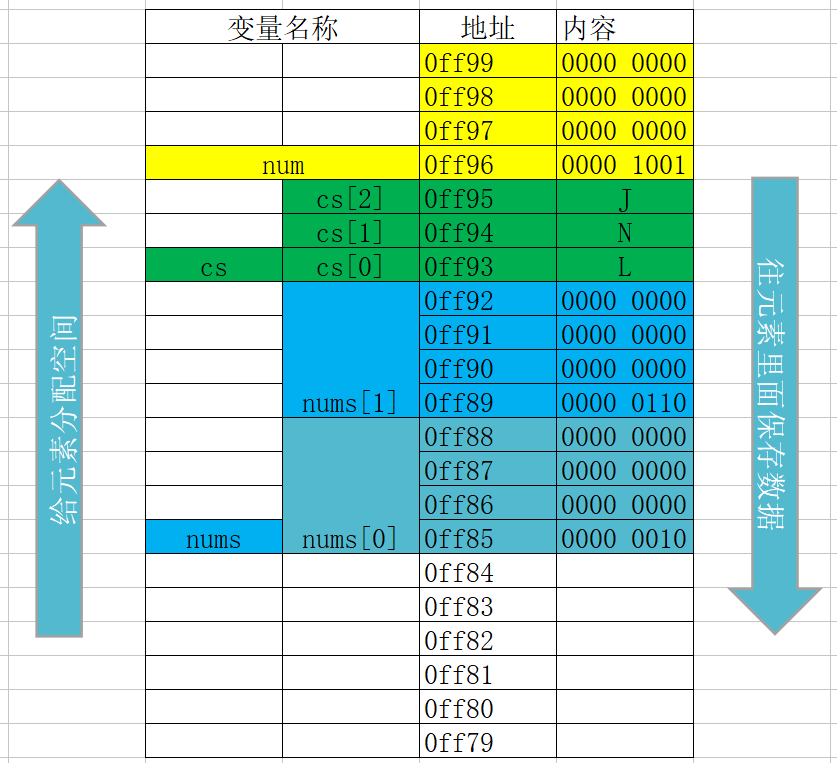• 注意:字符在内存中是以对应ASCII码值的二进制形式存储的,而非上述的形式。

## 数组的越界问题

• 数组越界导致的问题
• 约错对象
• 程序崩溃
``````    char cs1 = {1, 2};
char cs2 = {3, 4, 5};
cs2 = 88; // 注意:这句访问到了不属于cs1的内存
printf("cs1 = %d\n", cs1 );

## 数组注意事项

• 在定义数组的时候[]里面只能写整型常量或者是返回整型常量的表达式
``````int ages4['A'] = {19, 22, 33};
printf("ages4 = %d\n", ages4);

int ages5[5 + 5] = {19, 22, 33};
printf("ages5 = %d\n", ages5);

int ages5['A' + 5] = {19, 22, 33};
printf("ages5 = %d\n", ages5);``````
• 错误写法
``````// 没有指定元素个数，错误
int a[];

// []中不能放变量
int number = 10;
int ages[number]; // 老版本的C语言规范不支持
printf("%d\n", ages);

int number = 10;
int ages2[number] = {19, 22, 33} // 直接报错

// 只能在定义数组的时候进行一次性（全部赋值）的初始化
int ages3;
ages10 = {19, 22, 33};

// 一个长度为n的数组,最大下标为n-1, 下标范围:0~n-1
int ages4 = {19, 22, 33}
ages4; // 数组角标越界``````
• 练习
• 从键盘录入当天出售BTC的价格并计算出售的BTC的总价和平均价(比如说一天出售了10个比特币)

## 数组和函数

• 数组可以作为函数的参数使用,数组用作函数参数有两种形式:
• ￼一种是把数组元素作为实参使用
• ￼一种是把数组名作为函数的形参和实参使用

## 数组元素作为函数参数

• 数组的元素作为函数实参，与同类型的简单变量作为实参一样，如果是基本数据类型, 那么形参的改变不影响实参
``````void change(int val)// int val = number
{
val = 55;
}
int main(int argc, const char * argv[])
{
int ages = {1, 5, 8};
printf("ages = %d", ages);// 1
change(ages);
printf("ages = %d", ages);// 1
}``````
• 用数组元素作函数参数不要求形参也必须是数组元素

## 数组名作为函数参数

• 在C语言中,数组名除作为变量的标识符之外,数组名还代表了该数组在内存中的起始地址,因此,当数组名作函数参数时,实参与形参之间不是"值传递",而是"地址传递"
• 实参数组名将该数组的起始地址传递给形参数组,两个数组共享一段内存单元, 系统不再为形参数组分配存储单元
• 既然两个数组共享一段内存单元, 所以形参数组修改时，实参数组也同时被修改了
``````void change2(int array)// int array = 0ffd1
{
array = 88;
}
int main(int argc, const char * argv[])
{
int ages = {1, 5, 8};
printf("ages = %d", ages);// 1
change(ages);
printf("ages = %d", ages);// 88
}``````

## 数组名作函数参数的注意点

• 在函数形参表中,允许不给出形参数组的长度
``````void change(int array[])
{
array = 88;
}``````
• 形参数组和实参数组的类型必须一致,否则将引起错误。
``````void prtArray(double array) // 错误写法
{
for (int i = 0; i < 3; i++) {
printf("array[%d], %f", i, array[i]);
}
}
int main(int argc, const char * argv[])
{
int ages = {1, 5, 8};
prtArray(ages);
}``````
• 当数组名作为函数参数时, 因为自动转换为了指针类型，所以在函数中无法动态计算除数组的元素个数
``````void printArray(int array[])
{
printf("printArray size = %lu\n", sizeof(array)); // 8
int length = sizeof(array)/ sizeof(int); // 2
printf("length = %d", length);
}``````
• 练习:
• 设计一个函数int arrayMax(int a[], int count)找出数组元素的最大值
• 从键盘输入3个0-9的数字,然后输出0~9中哪些数字没有出现过
• 要求从键盘输入6个0~9的数字,排序后输出

## 计数排序（Counting Sort）

• 计数排序是一个非基于比较的排序算法，该算法于1954年由 Harold H. Seward 提出。它的优势在于在`对一定范围内的整数排序`时，快于任何比较排序算法。

• 排序思路:

• 1.找出待排序数组最大值
• 2.定义一个索引最大值为待排序数组最大值的数组
• 3.遍历待排序数组, 将待排序数组遍历到的值作新数组索引
• 4.在新数组对应索引存储值原有基础上+1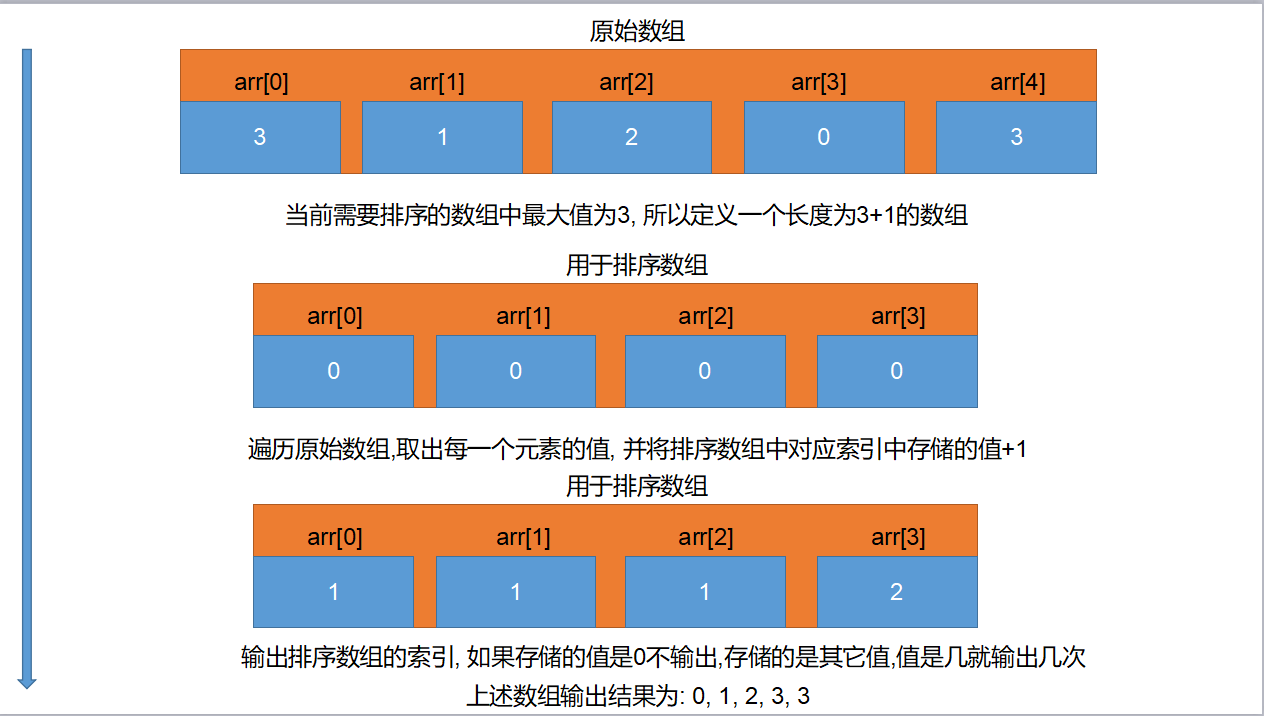• 简单代码实现:

``````int main()
{
// 待排序数组
int nums = {3, 1, 2, 0, 3};
// 用于排序数组
int newNums = {0};
// 计算待排序数组长度
int len = sizeof(nums) / sizeof(nums);
// 遍历待排序数组
for(int i = 0; i < len; i++){
// 取出待排序数组当前值
int index = nums[i];
// 将待排序数组当前值作为排序数组索引
// 将用于排序数组对应索引原有值+1
newNums[index] = newNums[index] +1;
}

// 计算待排序数组长度
int len2 = sizeof(newNums) / sizeof(newNums);
// 输出排序数组索引, 就是排序之后结果
for(int i = 0; i < len2; i++){
for(int j = 0; j < newNums[i]; j++){
printf("%i\n", i);
}
}
/*
// 计算待排序数组长度
int len2 = sizeof(newNums) / sizeof(newNums);
// 还原排序结果到待排序数组
for(int i = 0; i < len2; i++){
int index = 0;
for(int i = 0; i < len; i++){
for(int j = 0; j < newNums[i]; j++){
nums[index++] = i;
}
}
}
*/
return 0;
}``````

## 选择排序

• 选择排序(Selection sort)是一种简单直观的排序算法。它的工作原理如下。首先在未排序序列中找到最小元素,存放到排序序列的起始位置,然后,再从剩余未排序元素中继续寻找最小元素,然后放到排序序列末尾。以此类推,直到所有元素均排序完毕。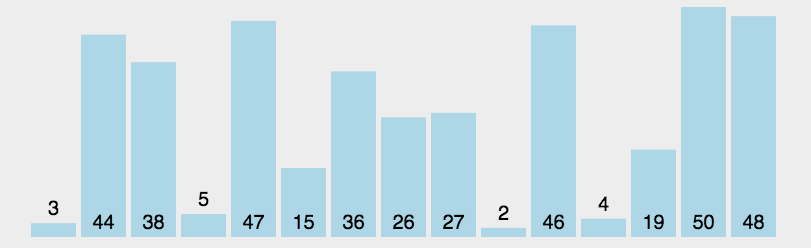• 排序思路:
• 假设按照升序排序
• 1.用第0个元素和后面所有元素依次比较
• 2.判断第0个元素是否大于当前被比较元素, 一旦小于就交换位置
• 3.第0个元素和后续所有元素比较完成后, 第0个元素就是最小值
• 4.排除第0个元素, 用第1个元素重复1~3操作, 比较完成后第1个元素就是倒数第二小的值
• 以此类推, 直到当前元素没有可比较的元素, 排序完成
• 代码实现:
``````// 选择排序
void selectSort(int numbers[], int length) {

// 外循环为什么要-1?
// 最后一位不用比较, 也没有下一位和它比较, 否则会出现错误访问
for (int i = 0; i < length; i++) {
for (int j = i; j < length - 1; j++) {
// 1.用当前元素和后续所有元素比较
if (numbers[i] < numbers[j + 1]) {
//  2.一旦发现小于就交换位置
swapEle(numbers, i, j + 1);
}
}
}
}
// 交换两个元素的值, i/j需要交换的索引
void swapEle(int array[], int i, int j) {
int temp = array[i];
array[i] = array[j];
array[j] = temp;
}``````

## 冒泡排序

• 冒泡排序(Bubble Sort)是一种简单的排序算法。它重复 地走访过要排序的数列,一次比较两个元素,如果他们的顺序错误就把他们交换过来。走访数列的工作是重复地进行直到没有再需要交换,也就是说该数列已经排序完成。这个算法的名字由来是因为越小的元素会经由交换慢慢“浮”到数列的顶端。
[外链图片转存中…(img-jCHVM4B0-1648814825893)]
• 排序思路:
• 假设按照升序排序
• 1.从第0个元素开始, 每次都用相邻两个元素进行比较
• 2.一旦发现后面一个元素小于前面一个元素就交换位置
• 3.经过一轮比较之后最后一个元素就是最大值
• 4.排除最后一个元素, 以此类推, 每次比较完成之后最大值都会出现再被比较所有元素的最后
• 直到当前元素没有可比较的元素, 排序完成
• 代码实现:
``````// 冒泡排序
void bubbleSort(int numbers[], int length) {
for (int i = 0; i < length; i++) {
// -1防止`角标越界`: 访问到了不属于自己的索引
for (int j = 0; j < length - i - 1; j++) {
//  1.用当前元素和相邻元素比较
if (numbers[j] < numbers[j + 1]) {
//  2.一旦发现小于就交换位置
swapEle(numbers, j, j + 1);
}
}
}
}
// 交换两个元素的值, i/j需要交换的索引
void swapEle(int array[], int i, int j) {
int temp = array[i];
array[i] = array[j];
array[j] = temp;
}``````

## 插入排序

• 插入排序（Insertion-Sort）的算法描述是一种简单直观的排序算法。它的工作原理是通过构建有序序列，对于未排序数据，在已排序序列中从后向前扫描，找到相应位置并插入。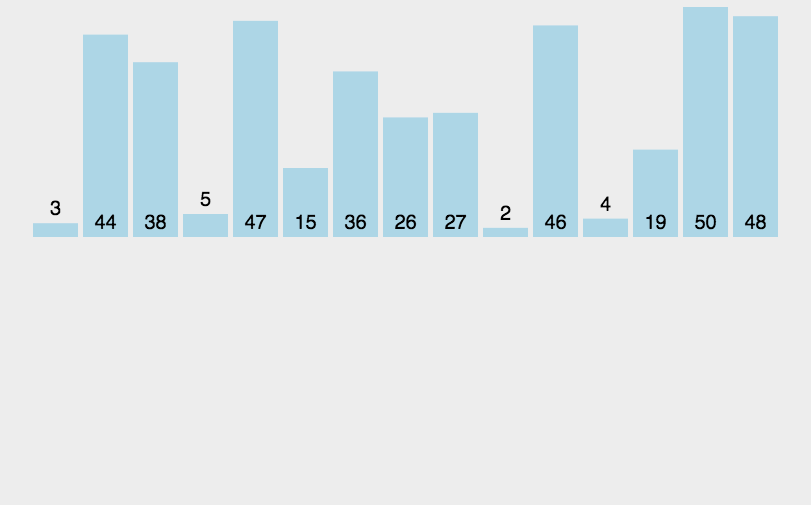• 排序思路:
• 假设按照升序排序
• 1.从索引为1的元素开始向前比较, 一旦前面一个元素大于自己就让前面的元素先后移动
• 2.直到没有可比较元素或者前面的元素小于自己的时候, 就将自己插入到当前空出来的位置
• 代码实现:
``````int main()
{
// 待排序数组
int nums = {3, 1, 2, 0, 3};
// 0.计算待排序数组长度
int len = sizeof(nums) / sizeof(nums);

//  1.从第一个元素开始依次取出所有用于比较元素
for (int i = 1; i < len; i++)
{
// 2.取出用于比较元素
int temp = nums[i];
int j = i;
while(j > 0){
// 3.判断元素是否小于前一个元素
if(temp < nums[j - 1]){
// 4.让前一个元素向后移动一位
nums[j] = nums[j - 1];
}else{
break;
}
j--;
}
// 5.将元素插入到空出来的位置
nums[j] = temp;
}
}``````
``````int main()
{
// 待排序数组
int nums = {3, 1, 2, 0, 3};
// 0.计算待排序数组长度
int len = sizeof(nums) / sizeof(nums);

//  1.从第一个元素开始依次取出所有用于比较元素
for (int i = 1; i < len; i++)
{
// 2.遍历取出前面元素进行比较
for(int j = i; j > 0; j--)
{
// 3.如果前面一个元素大于当前元素,就交换位置
if(nums[j-1] > nums[j]){
int temp = nums[j];
nums[j] = nums[j - 1];
nums[j - 1] = temp;
}else{
break;
}
}
}
}``````

## 希尔排序

• 1959年Shell发明，第一个突破O(n2)的排序算法，是简单插入排序的改进版。它与插入排序的不同之处在于，它会优先比较距离较远的元素。希尔排序又叫缩小增量排序。
[外链图片转存失败,源站可能有防盗链机制,建议将图片保存下来直接上传(img-Umu8w50P-1648814825894)(https://gitee.com/hzy2003628/drawing-bed/raw/master/images/20220313182846.gif)]

• 排序思路:

• 1.希尔排序可以理解为插入排序的升级版, 先将待排序数组按照指定步长划分为几个小数组
• 2.利用插入排序对小数组进行排序, 然后将几个排序的小数组重新合并为原始数组
• 3.重复上述操作, 直到步长为1时,再利用插入排序排序即可
• 代码实现:

``````int main()
{
// 待排序数组
int nums = {3, 1, 2, 0, 3};
// 0.计算待排序数组长度
int len = sizeof(nums) / sizeof(nums);

// 2.计算步长
int gap = len / 2;
do{
//  1.从第一个元素开始依次取出所有用于比较元素
for (int i = gap; i < len; i++)
{
// 2.遍历取出前面元素进行比较
int j = i;
while((j - gap) >= 0)
{
printf("%i > %i\n", nums[j - gap], nums[j]);
// 3.如果前面一个元素大于当前元素,就交换位置
if(nums[j - gap] > nums[j]){
int temp = nums[j];
nums[j] = nums[j - gap];
nums[j - gap] = temp;
}else{
break;
}
j--;
}
}
// 每个小数组排序完成, 重新计算步长
gap = gap / 2;
}while(gap >= 1);
}``````

## 折半查找

• 基本思路
• 在有序表中,取中间元素作为比较对象,若给定值与中间元素的要查找的数相等,则查找成功;若给定值小于中间元素的要查找的数,则在中间元素的左半区继续查找;
• 若给定值大于中间元素的要查找的数,则在中间元素的右半区继续查找。不断重复上述查找过 程,直到查找成功,或所查找的区域无数据元素,查找失败

• 实现步骤
• 在有序表中,取中间元素作为比较对象,若给定值与中间元素的要查找的数相等,则查找成功;
• 若给定值小于中间元素的要查找的数,则在中间元素的左半区继续查找;
• 若给定值大于中间元素的要查找的数,则在中间元素的右半区继续查找。
• 不断重复上述查找过 程,直到查找成功,或所查找的区域无数据元素,查找失败。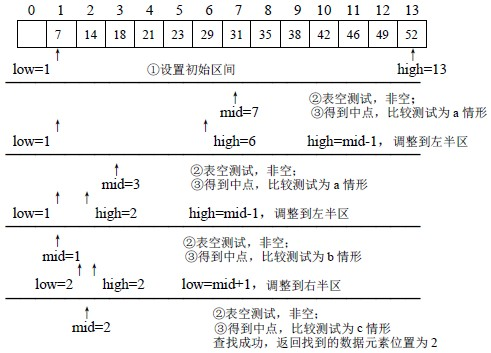• 代码实现

``````int findKey(int values[], int length, int key) {
// 定义一个变量记录最小索引
int min = 0;
// 定义一个变量记录最大索引
int max = length - 1;
// 定义一个变量记录中间索引
int mid = (min + max) * 0.5;

while (min <= max) {
// 如果mid对应的值 大于 key, 那么max要变小
if (values[mid] > key) {
max = mid - 1;
// 如果mid对应的值 小于 key, 那么min要变
}else if (values[mid] < key) {
min = mid + 1;
}else {
return mid;
}
// 修改完min/max之后, 重新计算mid的值
mid = (min + max) * 0.5;
}
return -1;
}``````

## 进制转换(查表法)

• 实现思路:
• 将二进制、八进制、十进制、十六进制所有可能的字符都存入数组
• 利用按位与运算符和右移依次取出当前进制对应位置的值
• 利用取出的值到数组中查询当前位输出的结果
• 将查询的结果存入一个新的数组, 当所有位都查询存储完毕, 新数组中的值就是对应进制的值
• 代码实现
``````#include <stdio.h>
void toBinary(int num)
{
total(num, 1, 1);
}
void toOct(int num)
{
total(num, 7, 3);
}
void toHex(int num)
{
total(num, 15, 4);
}

void total(int num , int base, int offset)
{
//    1.定义表用于查询结果
char cs[] = {
'0', '1', '2', '3', '4', '5',
'6', '7', '8', '9', 'a', 'b',
'c', 'd', 'e', 'f'
};
//    2.定义保存结果的数组
char rs;
//    计算最大的角标位置
int length = sizeof(rs)/sizeof(char);
int pos = length;//8

while (num != 0) {
int index = num & base;
rs[--pos] = cs[index];
num = num >> offset;
}

for (int i = pos; i < length; i++) {
printf("%c", rs[i]);
}
printf("\n");
}
int main()
{
toBinary(9);
return 0;
}``````

## 二维数组

• 所谓二维数组就是一个一维数组的每个元素又被声明为一 维数组,从而构成二维数组. 可以说二维数组是特殊的一维数组。
• 示例:
• int a = { {80,75,92}, {61,65,71}};
• 可以看作由一维数组a和一维数组a组成，这两个一维数组都包含了3个int类型的元素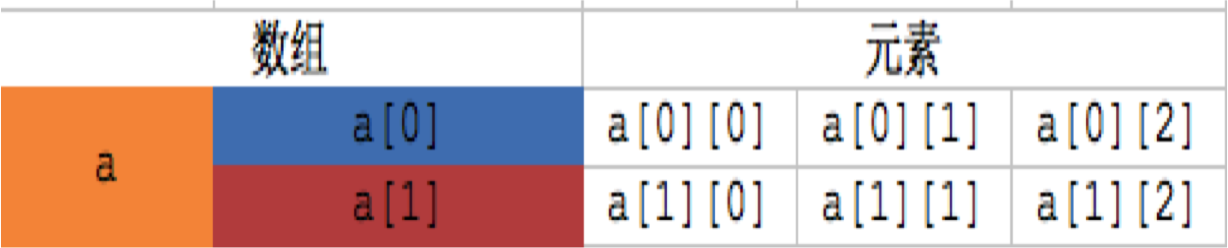## 二维数组的定义

• 格式:
• 数据类型 数组名[一维数组的个数][一维数组的元素个数]
• 其中"一维数组的个数"表示当前二维数组中包含多少个一维数组
• 其中"一维数组的元素个数"表示当前前二维数组中每个一维数组元素的个数

## 二维数组的初始化

• 二维数的初始化可分为两种:
• 定义的同时初始化
• 先定义后初始化
• 定义的同时初始化
``int a={ {80,75,92}, {61,65,71}};``
• 先定义后初始化
``````int a;
a = 80;
a = 75;
a = 92;
a = 61;
a = 65;
a = 71;``````
• 按行分段赋值
``int a={ {80,75,92}, {61,65,71}};``
• 按行连续赋值
``int a={ 80,75,92,61,65,71};``
• 其它写法
• 完全初始化,可以省略第一维的长度
``````int a[]={{1,2,3},{4,5,6}};
int a[]={1,2,3,4,5,6};``````
• 部分初始化,可以省略第一维的长度
``````int a[]={{1},{4,5}};
int a[]={1,2,3,4};``````
• 注意: 有些人可能想不明白，为什么可以省略行数，但不可以省略列数。也有人可能会问，可不可以只指定行数，但是省略列数？其实这个问题很简单，如果我们这样写：
int a[] = {1, 2, 3, 4, 5, 6}; // 错误写法
大家都知道，二维数组会先存放第1行的元素，由于不确定列数，也就是不确定第1行要存放多少个元素，所以这里会产生很多种情况，可能1、2是属于第1行的，也可能1、2、3、4是第一行的，甚至1、2、3、4、5、6全部都是属于第1行的
• 指定元素的初始化
``````int a={=10};
int a={={1,2,3}}``````

## 二维数组的应用场景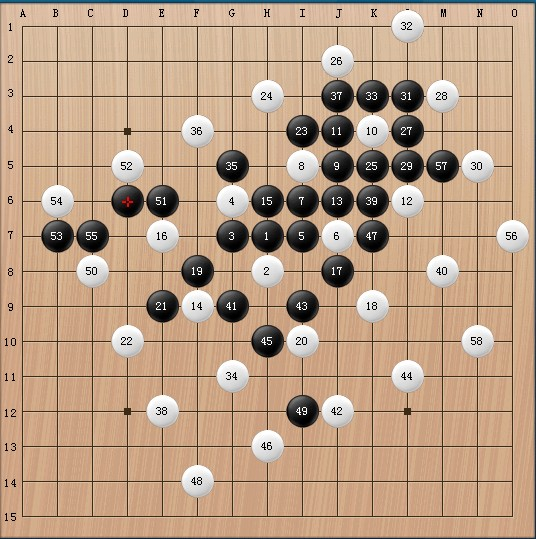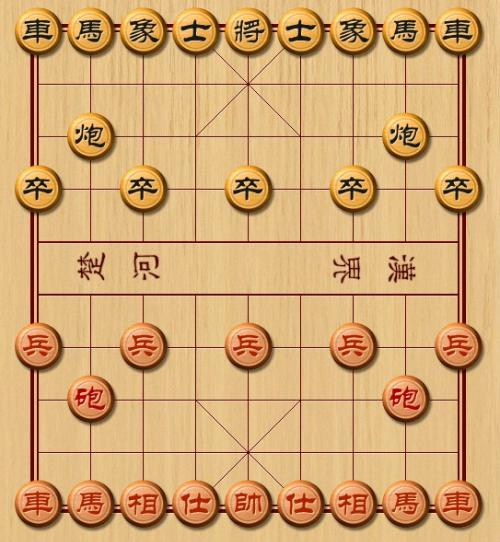## 二维数组的遍历

• 二维数组a,可分解为三个一维数组,其数组名分别为:
• 这三个一维数组都有4个元素,例如:一维数组a的 元素为a,a,a,a。
• 所以遍历二维数组无非就是先取出二维数组中得一维数组, 然后再从一维数组中取出每个元素的值
• 示例
``````char cs = {
{'a', 'b', 'c'},
{'d', 'e', 'f'}
};
printf("%c", cs);// 第一个取出一维数组, 第二个取出一维数组中对应的元素``````
``````char cs = {
{'a', 'b', 'c'},
{'d', 'e', 'f'}
};
for (int i = 0; i < 2; i++) { // 外循环取出一维数组
// i
for (int j = 0; j < 3; j++) {// 内循环取出一维数组的每个元素
printf("%c", cs[i][j]);
}
printf("\n");
}``````

## 二维数组的存储

• 和以为数组一样
• 给数组分配存储空间从内存地址大开始分配
• 给数组元素分配空间, 从所占用内存地址小的开始分配
• 往每个元素中存储数据从高地址开始存储
``````#include <stdio.h>
int main()
{
char cs = {
{'a', 'b', 'c'},
{'d', 'e', 'f'}
};
// cs == &cs == &cs == &cs
printf("cs = %p\n", cs);                // 0060FEAA
printf("&cs = %p\n", &cs);              // 0060FEAA
printf("&cs = %p\n", &cs);        // 0060FEAA
printf("&cs = %p\n", &cs);  // 0060FEAA
return 0;
}``````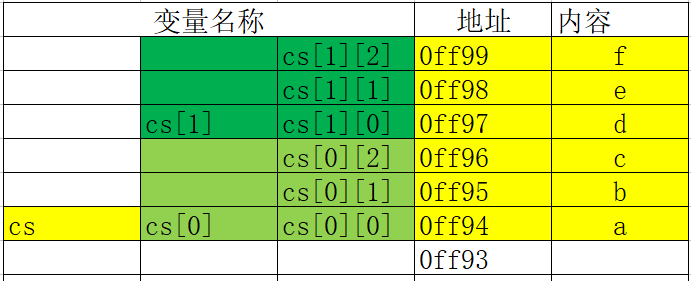## 二维数组与函数

• 值传递
``````#include <stdio.h>

// 和一位数组一样, 只看形参是基本类型还是数组类型
// 如果是基本类型在函数中修改形参不会影响实参
void change(char ch){
ch = 'n';
}
int main()
{
char cs = {
{'a', 'b', 'c'},
{'d', 'e', 'f'}
};
printf("cs = %c\n", cs); // a
change(cs);
printf("cs = %c\n", cs); // a
return 0;
}``````
• 地址传递
``````#include <stdio.h>

// 和一位数组一样, 只看形参是基本类型还是数组类型
// 如果是数组类型在函数中修改形参会影响实参
void change(char ch[]){
ch = 'n';
}
int main()
{
char cs = {
{'a', 'b', 'c'},
{'d', 'e', 'f'}
};
printf("cs = %c\n", cs); // a
change(cs);
printf("cs = %c\n", cs); // n
return 0;
}``````
``````#include <stdio.h>

// 和一位数组一样, 只看形参是基本类型还是数组类型
// 如果是数组类型在函数中修改形参会影响实参
void change(char ch[]){
ch = 'n';
}
int main()
{
char cs = {
{'a', 'b', 'c'},
{'d', 'e', 'f'}
};
printf("cs = %c\n", cs); // a
change(cs);
printf("cs = %c\n", cs); // n
return 0;
}``````

## 二维数组作为函数参数注意点

• 形参错误写法
``````void test(char cs[]) // 错误写法
{
printf("我被执行了\n");
}

void test(char cs) // 正确写法
{
printf("我被执行了\n");
}

void test(char cs[]) // 正确写法
{
printf("我被执行了\n");
}``````
• 二维数组作为函数参数，在被调函数中不能获得其有多少行，需要通过参数传入
``````void test(char cs)
{
int row = sizeof(cs); // 输出4或8
printf("row = %zu\n", row);
}``````
• 二维数组作为函数参数，在被调函数中可以计算出二维数组有多少列
``````void test(char cs)
{
size_t col = sizeof(cs); // 输出3
printf("col = %zd\n", col);
}``````

## 作业

• 玩家通过键盘录入 w,s,a,d控制小人向不同方向移动,其中w代表向上移动,s代表向 下移动,a代表向左移动,d 代表向右移动,当小人移动到出口位置,玩家胜利
• 思路:
• 1.定义二维数组存放地图
``````######
#O #
# ## #
#  # #
##   #
######``````

2.规定地图的方向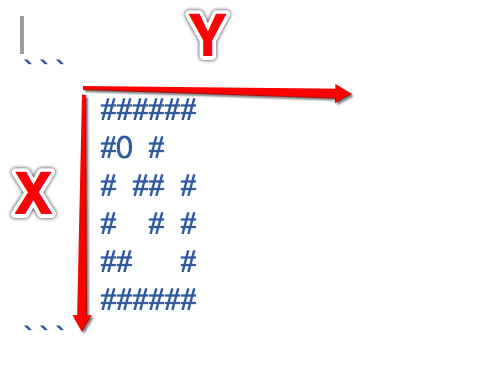• 3.编写程序控制方向
• 当输入w或者W, 小人向上移动. x-1
• 当输入s 或者S, 小人向下. x+1
• 当输入a或者A, 小人向左. y-1
• 当输入d或者D, 小人向右. y+1
• 4.移动小人
• 用变量记录小人当前的位置
• 1)如果小人将要移动的位置是墙,则无法移动
• 2)如果小人将要移动的位置是路,则可以移动
• 5.判断是否走出迷宫

## 字符串的基本概念

• 字符串是位于双引号中的字符序列
• 在内存中以“\0”结束,所占字节比实际多一个## 字符串的初始化

• 在C语言中没有专门的字符串变量,通常用一个字符数组来存放一个字符串。
• 当把一个字符串存入一个数组时,会把结束符‘\0’存入数组,并以此作为该字符串是否结束的标志。
• 有了‘\0’标志后,就不必再用字符数组 的长度来判断字符串的长度了
• 初始化
``````char name = "lnj"; //在内存中以“\0”结束， \0ASCII码值是0
char name1 = {'l','n','j','\0'};
char name2 = {'l','n','j',0};
// 当数组元素个数大于存储字符内容时, 未被初始化的部分默认值是0, 所以下面也可以看做是一个字符串
char name3 = {'l','n','j'};``````
• 错误的初始化方式
``````    //省略元素个数时, 不能省略末尾的\n
// 不正确地写法，结尾没有\0 ，只是普通的字符数组
char name4[] = {'l','n','j'};

//   "中间不能包含\0", 因为\0是字符串的结束标志
//    \0的作用：字符串结束的标志
char name[] = "c\0ool";
printf("name = %s\n",name);

## 字符串输出

• 如果字符数组中存储的是一个字符串, 那么字符数组的输入输出将变得简单方便。
• 不必使用循环语句逐个地输入输出每个字符
• 可以使用printf函数和scanf函数一次性输出输入一个字符数组中的字符串
• 使用的格式字符串为“%s”,表示输入、输出的是一个字符串 字符串的输出

• 输出
• %s的本质就是根据传入的name的地址逐个去取数组中的元素然后输出，直到遇到\0位置
``````char chs[] = "lnj";
printf("%s\n", chs);``````
• 注意点:
• \0引发的脏读问题
``````char name[] = {'c', 'o', 'o', 'l' , '\0'};
char name2[] = {'l', 'n', 'j'};
printf("name2 = %s\n", name2); // 输出结果: lnjcool``````
• 输入
``````char ch;
scanf("%s",ch);``````
• 注意点:
• 对一个字符串数组, 如果不做初始化赋值, 必须指定数组长度
• ch最多存放由9个字符构成的字符串，其中最后一个字符的位置要留给字符串的结尾标示‘\0’
• 当用scanf函数输入字符串时,字符串中不能含有空格,否则将以空格作为串的结束符

## 字符串常用方法

• C语言中供了丰富的字符串处理函数,大致可分为字符串的输入、输出、合并、修改、比较、转 换、复制、搜索几类。
• 使用这些函数可大大减轻编程的负担。
• 使用输入输出的字符串函数,在使用前应包含头文件"stdio.h"
• 使用其它字符串函数则应包含头文件"string.h"

• 字符串输出函数:puts
• 格式: puts(字符数组名)
• 功能:把字符数组中的字符串输出到显示器。即在屏幕上显示该字符串。
• 优点:
• 自动换行
• 可以是数组的任意元素地址
• 缺点
• 不能自定义输出格式, 例如 puts(“hello %i”);
``````char ch[] = "lnj";
puts(ch); //输出结果: lnj``````
• puts函数完全可以由printf函数取代。当需要按一定格式输出时,通常使用printf函数
• 字符串输入函数:gets
• 格式: gets (字符数组名)
• 功能:从标准输入设备键盘上输入一个字符串。
``````char ch;
gets(ch); // 输入:lnj
puts(ch); // 输出:lnj``````
• 可以看出当输入的字符串中含有空格时,输出仍为全部字符串。说明gets函数并不以空格作为字符串输入结束的标志,而只以回车作为输入结束。这是与scanf函数不同的。
• 注意gets很容易导致数组下标越界，是一个不安全的字符串操作函数
• 字符串长度
• 利用sizeof字符串长度
• 因为字符串在内存中是逐个字符存储的,一个字符占用一个字节,所以字符串的结束符长度也是占用的内存单元的字节数。
``````char name[] = "it666";
int size = sizeof(name);// 包含\0
printf("size = %d\n", size); //输出结果:6``````
• 利用系统函数
• 格式: strlen(字符数组名)
• 功能:测字符串的实际长度(不含字符串结束标志‘\0’)并作为函数返回值。
``````char name[] = "it666";
size_t len = strlen(name2);
printf("len = %lu\n", len); //输出结果:5``````
• 以“\0”为字符串结束条件进行统计
``````/**
*  自定义方法计算字符串的长度
*  @param name 需要计算的字符串
*  @return 不包含\0的长度
*/
int myStrlen2(char str[])
{
//    1.定义变量保存字符串的长度
int length = 0;
while (str[length] != '\0')
{
length++;//1 2 3 4
}
return length;
}
/**
*  自定义方法计算字符串的长度
*  @param name  需要计算的字符串
*  @param count 字符串的总长度
*  @return 不包含\0的长度
*/
int myStrlen(char str[], int count)
{
//    1.定义变量保存字符串的长度
int length = 0;
//    2.通过遍历取出字符串中的所有字符逐个比较
for (int i = 0; i < count; i++) {
//        3.判断是否是字符串结尾
if (str[i] == '\0') {
return length;
}
length++;
}
return length;
}``````
• 字符串连接函数:strcat
• 格式: strcat(字符数组名1,字符数组名2)
• 功能:把字符数组2中的字符串连接到字符数组1 中字符串的后面,并删去字符串1后的串标志 “\0”。本函数返回值是字符数组1的首地址。
``````char oldStr = "welcome to";
char newStr = " lnj";
strcat(oldStr, newStr);
puts(oldStr); //输出: welcome to lnj"``````
• 本程序把初始化赋值的字符数组与动态赋值的字符串连接起来。要注意的是,字符数组1应定义足 够的长度,否则不能全部装入被连接的字符串。
• 字符串拷贝函数:strcpy
`- 格式: strcpy(字符数组名1,字符数组名2)`- 功能:把字符数组2中的字符串拷贝到字符数组1中。串结束标志“\0”也一同拷贝。字符数名2, 也可以是一个字符串常量。这时相当于把一个字符串赋予一个字符数组。
``````char oldStr = "welcome to";
char newStr = " lnj";
strcpy(oldStr, newStr);
puts(oldStr); // 输出结果:  lnj // 原有数据会被覆盖``````
• 本函数要求字符数组1应有足够的长度,否则不能全部装入所拷贝的字符串。

• 字符串比较函数:strcmp
• 格式: strcmp(字符数组名1,字符数组名2)
• 功能:按照ASCII码顺序比较两个数组中的字符串,并由函数返回值返回比较结果。
• 字符串1=字符串2,返回值=0;
• 字符串1>字符串2,返回值>0;
• 字符串1<字符串2,返回值<0。
``````char oldStr = "0";
char newStr = "1";
printf("%d", strcmp(oldStr, newStr)); //输出结果:-1
char oldStr = "1";
char newStr = "1";
printf("%d", strcmp(oldStr, newStr));  //输出结果:0
char oldStr = "1";
char newStr = "0";
printf("%d", strcmp(oldStr, newStr)); //输出结果:1``````

## 练习

• 编写一个函数char_contains(char str[],char key)， 如果字符串str中包含字符key则返回数值1，否则返回数值0

## 字符串数组基本概念

• 字符串数组其实就是定义一个数组保存所有的字符串
• 1.一维字符数组中存放一个字符串，比如一个名字char name = “nj”
• 2.如果要存储多个字符串，比如一个班所有学生的名字，则需要二维字符数组，char names可以存放15个学生的姓名(假设姓名不超过20字符)
• 如果要存储两个班的学生姓名，那么可以用三维字符数组char names
##字符串数组的初始化
``````char names = { {'l','n','j','\0'}, {'l','y','h','\0'} };
char names2 = { {"lnj"}, {"lyh"} };
char names3 = { "lnj", "lyh" };``````

## 指针基本概念

• 什么是地址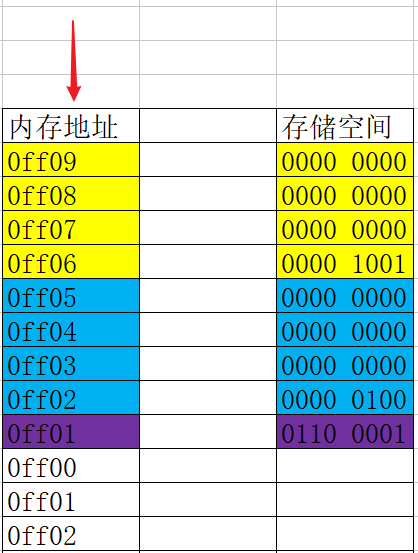• 地址与内存单元中的数据是两个完全不同的概念
• 地址如同房间编号, 根据这个编号我们可以找到对应的房间
• 内存单元如同房间, 房间是专门用于存储数据的
• 变量地址:
• 系统分配给"变量"的"内存单元"的起始地址
``````int num = 6; // 占用4个字节
//那么变量num的地址为: 0ff06

char c = 'a'; // 占用1个字节
//那么变量c的地址为:0ff05``````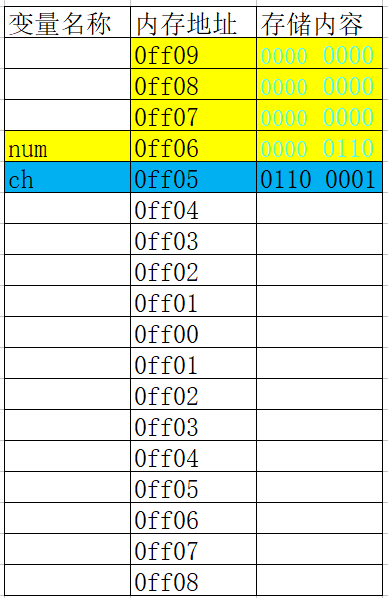## 什么是指针

• 在计算机中所有数据都存储在内存单元中,而每个内存单元都有一个对应的地址, 只要通过这个地址就能找到对应单元中存储的数据.
• 由于通过地址能找到所需的变量单元，所以我们说该地址指向了该变量单元。将地址形象化的称为“指针”
• 内存单元的指针(地址)和内存单元的内容是两个不同的概念。## 什么是指针变量

• 在C语言中,允许用一个变量来存放其它变量的地址, 这种专门用于存储其它变量地址的变量, 我们称之为指针变量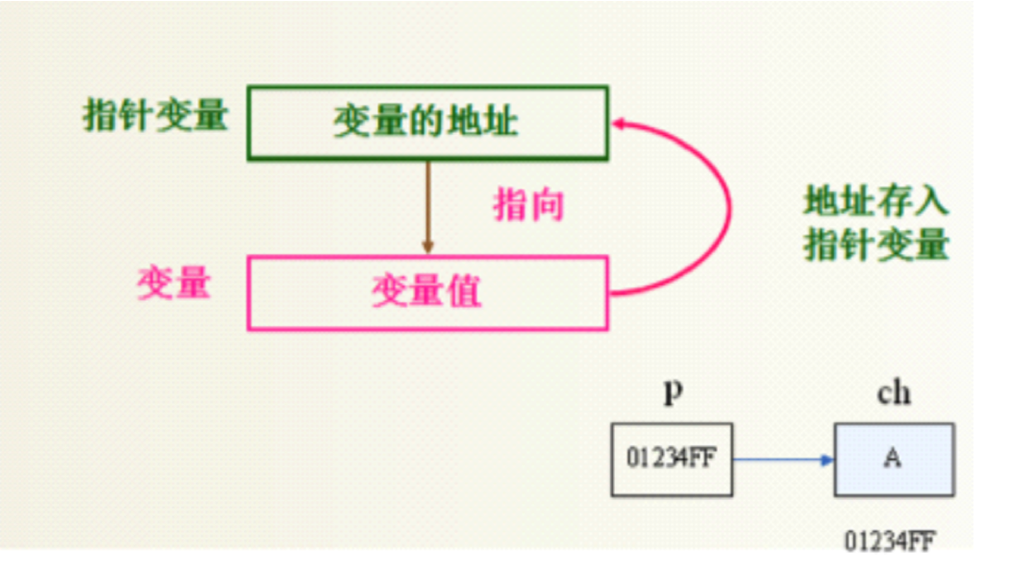• 示例:

``````int age;// 定义一个普通变量
num = 10;
int *pnAge; // 定义一个指针变量
pnAge = &age;``````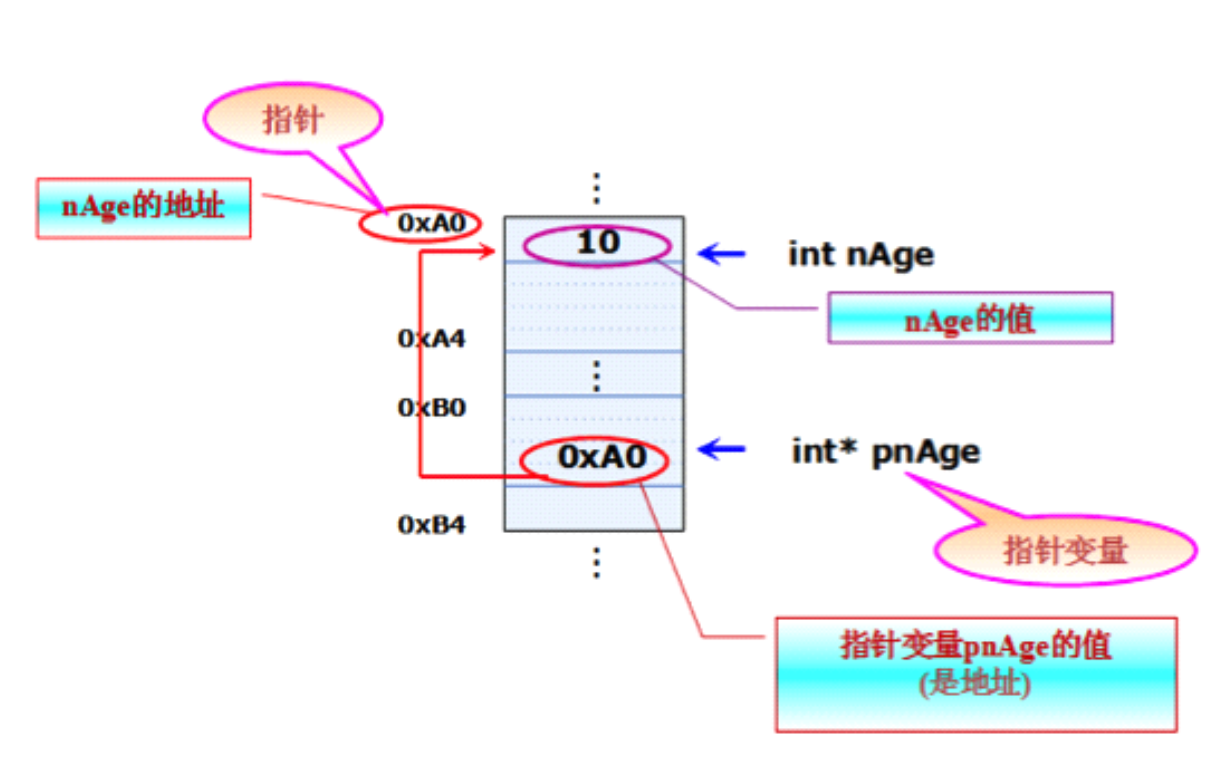## 定义指针变量的格式

• 指针变量的定义包括两个内容:

• 指针类型说明,即定义变量为一个指针变量;
• 指针变量名;• 示例:

``````char ch = 'a';
char *p; // 一个用于指向字符型变量的指针
p = &ch;
int num = 666;
int *q; // 一个用于指向整型变量的指针
q = &num;``````
• 其中,*表示这是一个指针变量
• 变量名即为定义的指针变量名
• 类型说明符表示本指针变量所指向的变量的数据类型

## 指针变量的初始化方法

• 指针变量初始化的方法有两种:定义的同时进行初始化和先定义后初始化
• 定义的同时进行初始化
``````int a = 5;
int *p = &a;``````
• 先定义后初始化
``````int a = 5;
int *p;
p=&a;``````
• 把指针初始化为NULL
``````int *p=NULL;
int *q=0;``````
• 不合法的初始化:
• 指针变量只能存储地址, 不能存储其它类型
``````int *p;
p =  250; // 错误写法``````
• 给指针变量赋值时,指针变量前不能再加“*”
``````int *p;
*p=&a; //错误写法``````

• 多个指针变量可以指向同一个地址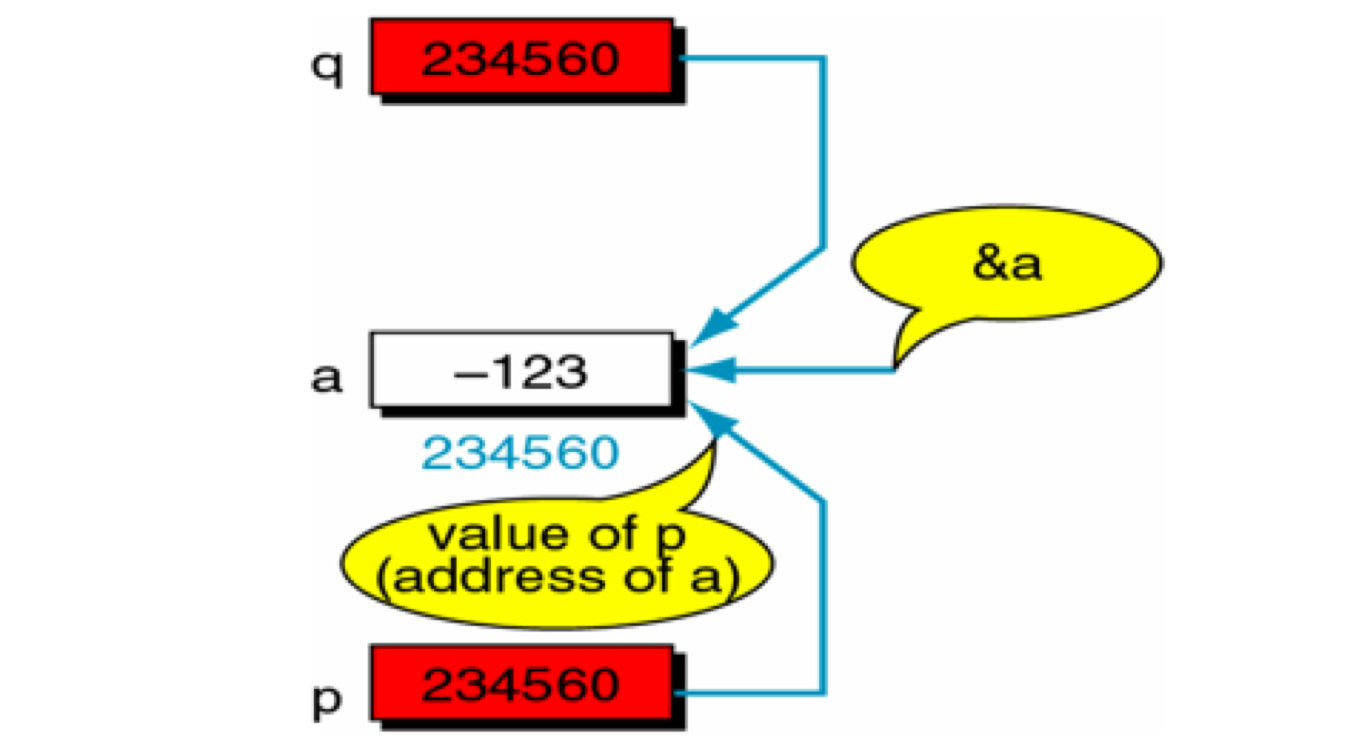• 指针的指向是可以改变的

``````int a = 5;
int *p = &a;
int b = 10;
p = &b; // 修改指针指向``````

• 野指针可能会导致程序崩溃
• 野指针访问你不该访问数据
• 所以指针必须初始化才可以访问其所指向存储区域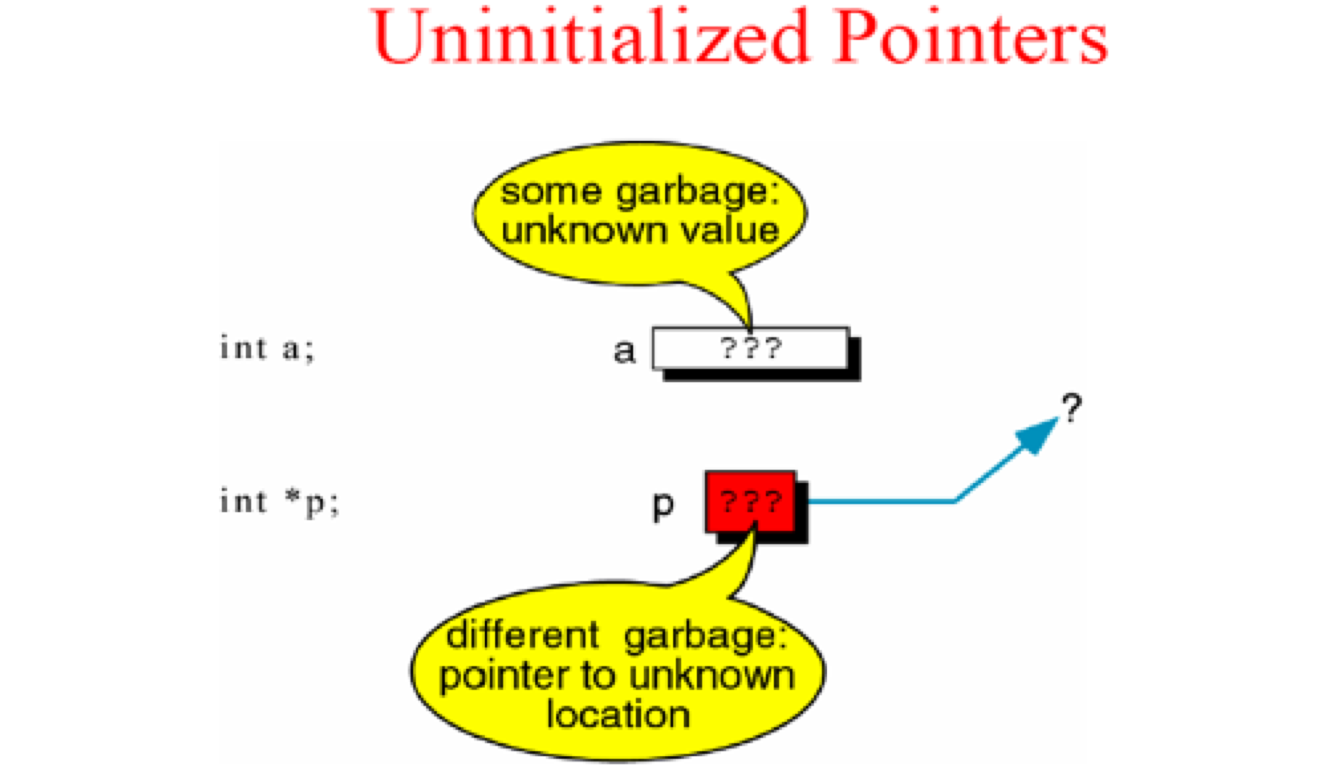## 访问指针所指向的存储空间

• C语言中提供了地址运算符&来表示变量的地址。其一般形式为:
• &变量名;
• C语言中提供了*来定义指针变量和访问指针变量指向的内存存储空间
• 在定义变量的时候 * 是一个类型说明符,说明定义的这个变量是一个指针变量
``int *p=NULL; // 定义指针变量``
• 在不是定义变量的时候 *是一个操作符,代表访问指针所指向存储空间
``````int a = 5;
int *p = &a;
printf("a = %d", *p); // 访问指针变量``````

## 指针类型

• 在同一种编译器环境下,一个指针变量所占用的内存空间是固定的。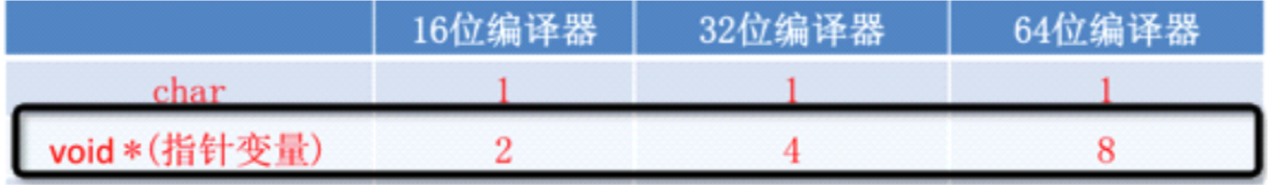• 一个int占用4个字节，一个char占用1个字节，而一个double占用8字节；
• 现在只有一个地址，我怎么才能知道要从这个地址开始向后访问多少个字节的存储空间呢，是4个，是1个，还是8个。
• 所以指针变量需要它所指向的数据类型告诉它要访问多少个字节存储空间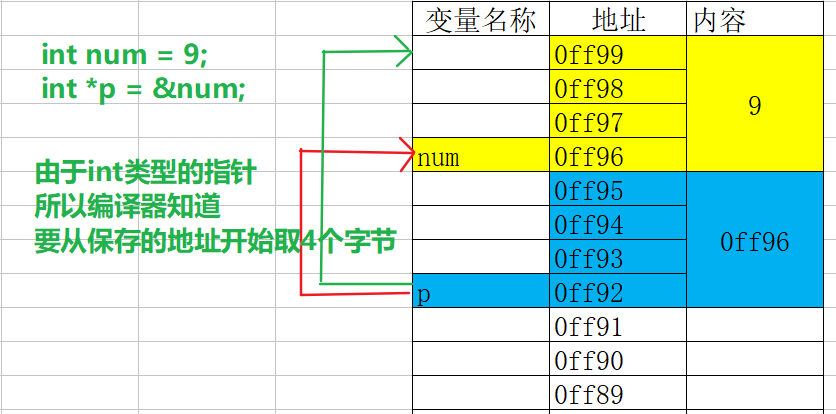## 二级指针

• 如果一个指针变量存放的又是另一个指针变量的地址,则称这个指针变量为指向指针的指针￼变量。也称为“二级指针”
``````char c = 'a';
char *cp;
cp = &c;
char **cp2;
cp2 = &cp;
printf("c = %c", **cp2);``````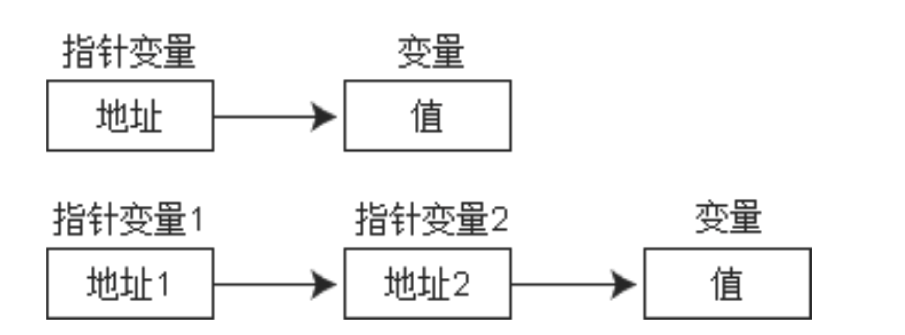• 多级指针的取值规则
``````int ***m1;  //取值***m1
int *****m2; //取值*****m2``````

## 练习

• 定义一个函数交换两个变量的值
• 写一个函数，同时返回两个数的和与差

##数组指针的概念及定义

• 数组元素指针
• 一个变量有地址,一个数组包含若干元素,每个数组元素也有相应的地址, 指针变量也可以保存数组元素的地址
• 只要一个指针变量保存了数组元素的地址, 我们就称之为数组元素指针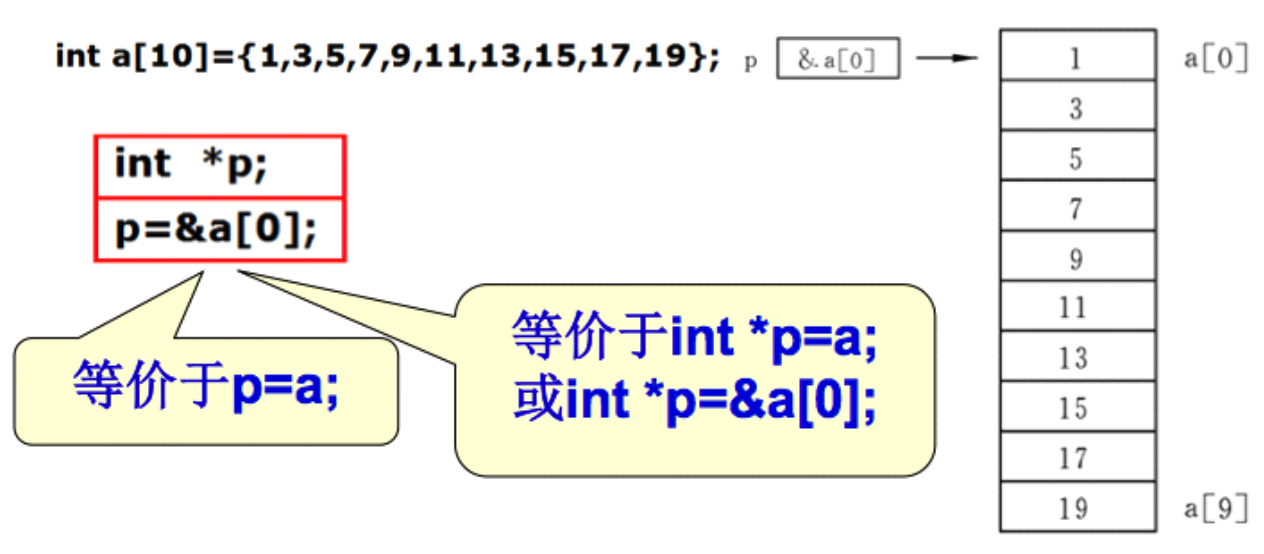``printf(“%p %p”, &(a), a); //输出结果:0x1100, 0x1100``
• 注意: 数组名a不代表整个数组,只代表数组首元素的地址。
• “p=a;”的作用是“把a数组的首元素的地址赋给指针变量p”,而不是“把数组a各元素的值赋给 p”

## 指针访问数组元素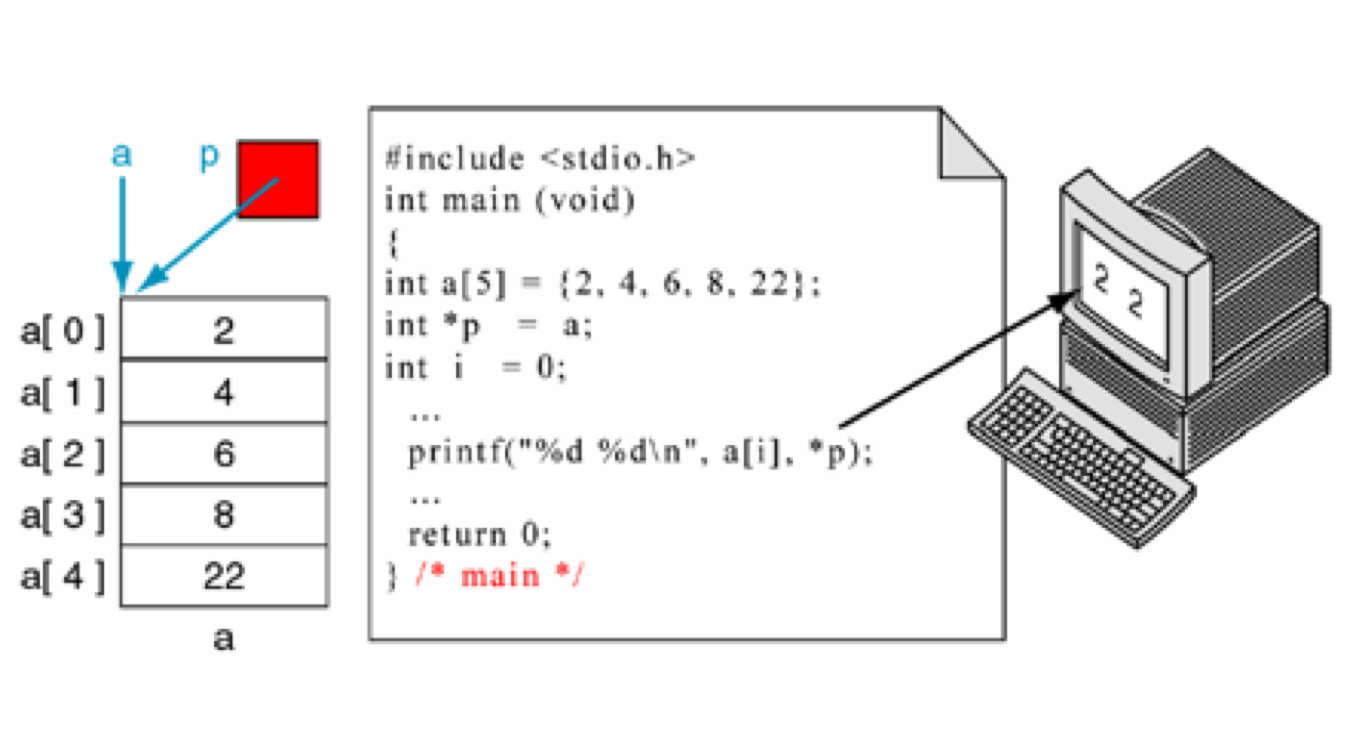``````    int main (void)
{
int a = {2, 4, 6, 8, 22};
int *p;
// p = &(a);
p = a;
printf(“%d %d\n”,a,*p); // 输出结果: 2, 2
}``````
• 在指针指向数组元素时,允许以下运算:
• 加一个整数(用+或+=),如p+1
• 减一个整数(用-或-=),如p-1
• 自加运算,如p++,++p
• 自减运算,如p–,–p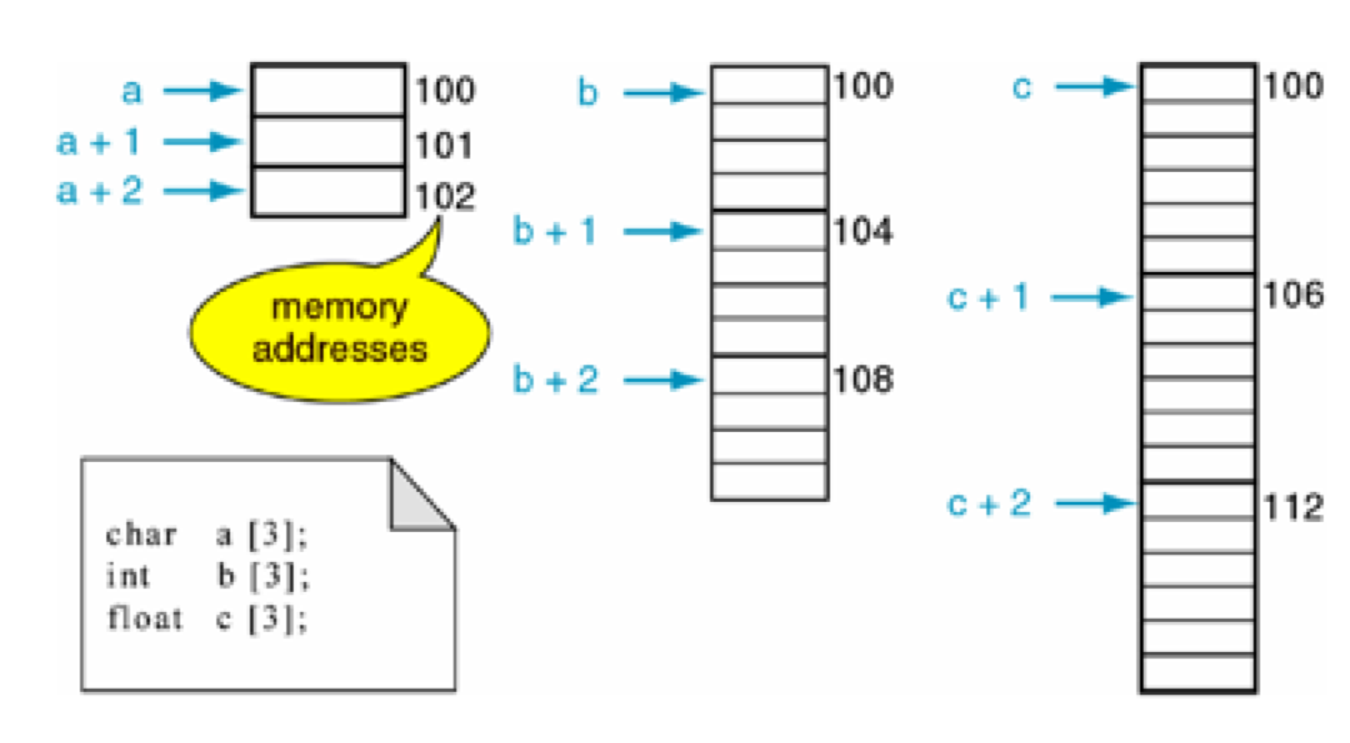• 如果指针变量p已指向数组中的一个元素,则p+1`指向`同一数组中的下一个元素,p-1`指向`同 一数组中的上一个元素。
• 结论: 访问数组元素,可用下面两种方法:
• 下标法, 如a[i]形式
• 指针法, *(p+i)形式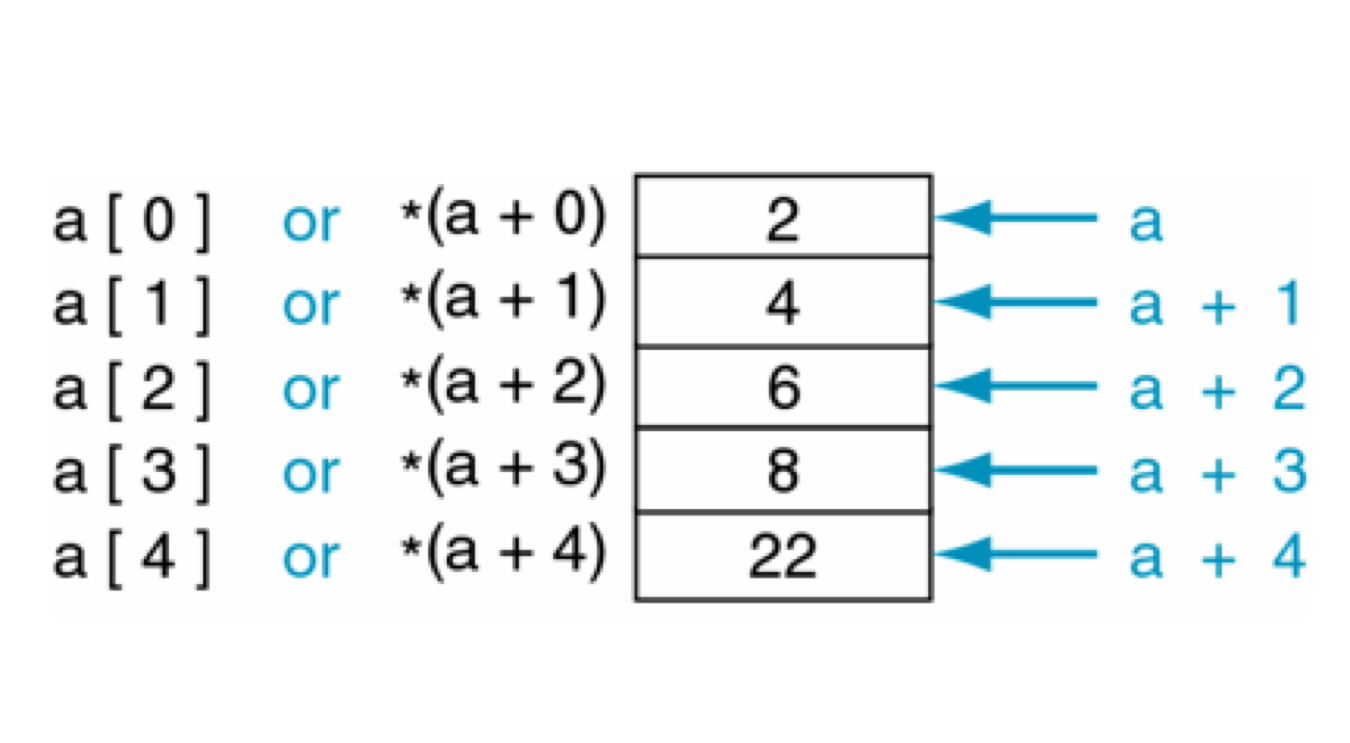• 注意:
• 数组名虽然是数组的首地址，但是数组名所所保存的数组的首地址是不可以更改的
``````   int x;
x++;  //错误
int* p = x;
p++; //正确``````

## 指针与字符串

• 定义字符串的两种方式
• 字符数组
``````char string[]=”I love lnj!”;
printf("%s\n",string);``````
• 字符串指针指向字符串
``````// 数组名保存的是数组第0个元素的地址, 指针也可以保存第0个元素的地址
char *str = "abc"``````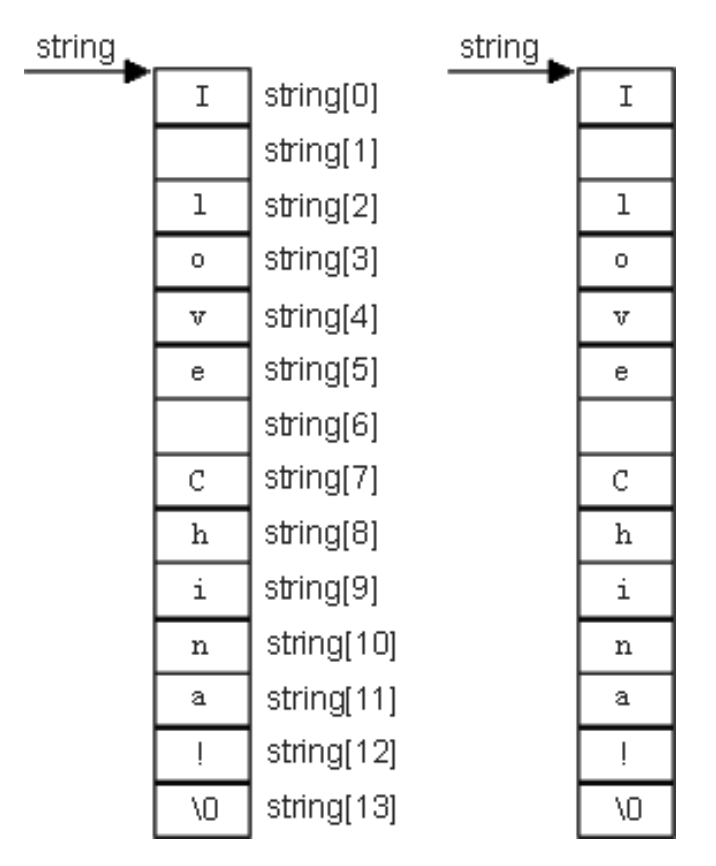• 字符串指针使用注意事项
• 可以查看字符串的每一个字符
``````har *str = "lnj";
for(int i = 0; i < strlen(str);i++)
{
printf("%c-", *(str+i)); // 输出结果:l-n-j
}``````
• 不可以修改字符串内容
``````//   + 使用字符数组来保存的字符串是保存栈里的,保存栈里面东西是可读可写,所有可以修改字符串中的的字符
//   + 使用字符指针来保存字符串,它保存的是字符串常量地址,常量区是只读的,所以我们不可以修改字符串中的字符
char *str = "lnj";
*(str+2) = 'y'; // 错误``````
• 不能够直接接收键盘输入
``````// 错误的原因是:str是一个野指针,他并没有指向某一块内存空间
// 所以不允许这样写如果给str分配内存空间是可以这样用 的
char *str;
scanf("%s", str);``````

## 指向函数指针

• 为什么指针可以指向一个函数？
• 函数作为一段程序，在内存中也要占据部分存储空间，它也有一个起始地址
• 函数有自己的地址，那就好办了，我们的指针变量就是用来存储地址的。
• 因此可以利用一个指针指向一个函数。其中，函数名就代表着函数的地址。
• 指针函数的定义
• 格式: `返回值类型 (*指针变量名)(形参1, 形参2, ...);`
``````int sum(int a,int b)
{
return a + b;
}

int (*p)(int,int);
p = sum;``````
• 指针函数定义技巧
• 1、把要指向函数头拷贝过来
• 2、把函数名称使用小括号括起来
• 3、在函数名称前面加上一个*
• 4、修改函数名称
• 应用场景
• 调用函数
• 将函数作为参数在函数间传递
• 注意点:
• 由于这类指针变量存储的是一个函数的入口地址，所以对它们作加减运算(比如p++)是无意义的
• 函数调用中"(指针变量名)"的两边的括号不可少,其中的不应该理解为求值运算,在此处它 只是一种表示符号

## 什么是结构体

• 结构体和数组一样属于构造类型
• 数组是用于保存一组相同类型数据的, 而结构体是用于保存一组不同类型数组的
• 例如,在学生登记表中,姓名应为字符型;学号可为整型或字符型;年龄应为整型;性别应为字符型;成绩可为整型或实型。
• 显然这组数据不能用数组来存放, 为了解决这个问题,C语言中给出了另一种构造数据类型——“结构(structure)”或叫“结构体”。

## 定义结构体类型

• 在使用结构体之前必须先定义结构体类型, 因为C语言不知道你的结构体中需要存储哪些类型数据, 我们必须通过定义结构体类型来告诉C语言, 我们的结构体中需要存储哪些类型的数据
• 格式:
``````struct　结构体名{
类型名1　成员名1;
类型名2　成员名2;
……
类型名n　成员名n;
};``````
• 示例:
``````struct Student {
char *name; // 姓名
int age; // 年龄
float height; // 身高
};``````

## 定义结构体变量

• 定好好结构体类型之后, 我们就可以利用我们定义的结构体类型来定义结构体变量
• 格式: `struct 结构体名 结构体变量名;`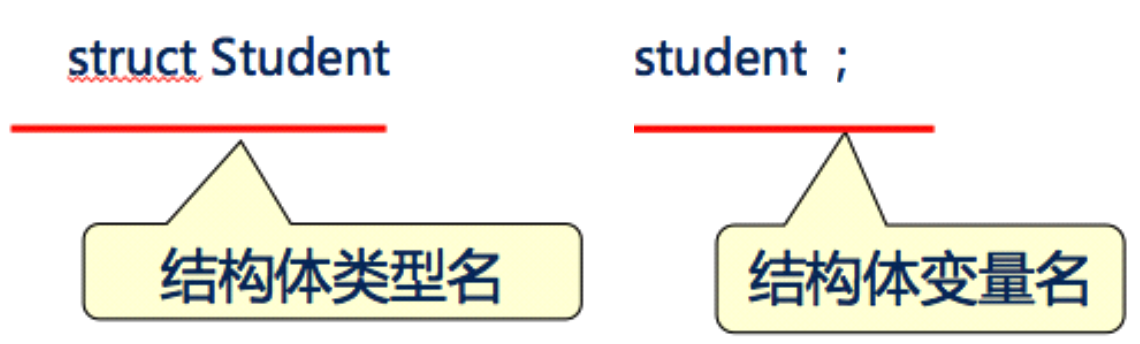• 先定义结构体类型，再定义变量
``````struct Student {
char *name;
int age;
};

struct Student stu;``````
• 定义结构体类型的同时定义变量
``````struct Student {
char *name;
int age;
} stu;``````
• 匿名结构体定义结构体变量
``````struct {
char *name;
int age;
} stu;``````
• 第三种方法与第二种方法的区别在于,第三种方法中省去了结构体类型名称,而直接给出结构变量,这种结构体最大的问题是结构体类型不能复用

## 结构体成员访问

• 一般对结构体变量的操作是以成员为单位进行的，引用的一般形式为：`结构体变量名.成员名`
``````struct Student {
char *name;
int age;
};
struct Student stu;
// 访问stu的age成员
stu.age = 27;
printf("age = %d", stu.age);``````

## 结构体变量的初始化

• 定义的同时按顺序初始化
``````struct Student {
char *name;
int age;
};
struct Student stu = {“lnj", 27};``````
• 定义的同时不按顺序初始化
``````struct Student {
char *name;
int age;
};
struct Student stu = {.age = 35, .name = “lnj"};``````
• 先定义后逐个初始化
``````struct Student {
char *name;
int age;
};
struct Student stu;
stu.name = "lnj";
stu.age = 35;``````
• 先定义后一次性初始化
``````struct Student {
char *name;
int age;
};
struct Student stu;
stu2 = (struct Student){"lnj", 35};``````

## 结构体类型作用域

• 结构类型定义在函数内部的作用域与局部变量的作用域是相同的
• 从定义的那一行开始, 直到遇到return或者大括号结束为止
• 结构类型定义在函数外部的作用域与全局变量的作用域是相同的
• 从定义的那一行开始,直到本文件结束为止
``````//定义一个全局结构体,作用域到文件末尾
struct Person{
int age;
char *name;
};

int main(int argc, const char * argv[])
{
//定义局部结构体名为Person,会屏蔽全局结构体
//局部结构体作用域,从定义开始到“}”块结束
struct Person{
int age;
};
// 使用局部结构体类型
struct Person pp;
pp.age = 50;
pp.name = "zbz";

test();
return 0;
}

void test() {

//使用全局的结构体定义结构体变量p
struct Person p = {10,"sb"};
printf("%d,%s\n",p.age,p.name);
}``````

## 结构体数组

• 结构体数组和普通数组并无太大差异, 只不过是数组中的元素都是结构体而已
• 格式: `struct 结构体类型名称 数组名称[元素个数]`
``````struct Student {
char *name;
int age;
};
struct Student stu;``````
• 结构体数组初始化和普通数组也一样, 分为先定义后初始化和定义同时初始化
• 定义同时初始化
``````struct Student {
char *name;
int age;
};
struct Student stu = {{"lnj", 35},{"zs", 18}};``````
• 先定义后初始化
``````struct Student {
char *name;
int age;
};
struct Student stu;
stu = {"lnj", 35};
stu = {"zs", 18};``````

## 结构体指针

• 一个指针变量当用来指向一个结构体变量时,称之为结构体指针变量

• 格式: `struct 结构名 *结构指针变量名`

• 示例:

``````     // 定义一个结构体类型
struct Student {
char *name;
int age;
};

// 定义一个结构体变量
struct Student stu = {“lnj", 18};

// 定义一个指向结构体的指针变量
struct Student *p;

// 指向结构体变量stu
p = &stu;

/*
这时候可以用3种方式访问结构体的成员
*/
// 方式1：结构体变量名.成员名
printf("name=%s, age = %d \n", stu.name, stu.age);

// 方式2：(*指针变量名).成员名
printf("name=%s, age = %d \n", (*p).name, (*p).age);

// 方式3：指针变量名->成员名
printf("name=%s, age = %d \n", p->name, p->age);

return 0;
}``````
• 通过结构体指针访问结构体成员, 可以通过以下两种方式

• (*结构指针变量).成员名
• 结构指针变量->成员名(用熟)
• (pstu)两侧的括号不可少,因为成员符“.”的优先级高于“”。
• 如去掉括号写作pstu.num则等效于(pstu.num),这样,意义就完全不对了。

## 结构体内存分析

• 给结构体变量开辟存储空间和给普通开辟存储空间一样, 会从内存地址大的位置开始开辟
• 给结构体成员开辟存储空间和给数组元素开辟存储空间一样, 会从所占用内存地址小的位置开始开辟
• 结构体变量占用的内存空间永远是所有成员中占用内存最大成员的倍数(对齐问题)

+多实际的计算机系统对基本类型数据在内存中存放的位置有限制,它们会要求这些数据的起始地址的值是 某个数k的倍数,这就是所谓的内存对齐,而这个k则被称为该数据类型的对齐模数(alignment modulus)。

• 这种强制的要求一来简化了处理器与内存之间传输系统的设计,二来可以提升读取数据的速度。比如这么一种处理器,它每次读写内存的时候都从某个8倍数的地址开始,一次读出或写入8个字节的数据,假如软件能 保证double类型的数据都从8倍数地址开始,那么读或写一个double类型数据就只需要一次内存操作。否则,我们就可能需要两次内存操作才能完成这个动作,因为数据或许恰好横跨在两个符合对齐要求的8字节 内存块上

## 结构体变量占用存储空间大小

``````struct Person{
int age; // 4
char ch; // 1
double score; // 8
};
struct Person p;
printf("sizeof = %i\n", sizeof(p)); // 16``````
• 占用内存最大属性是score, 占8个字节, 所以第一次会分配8个字节
• 将第一次分配的8个字节分配给age4个,分配给ch1个, 还剩下3个字节
• 当需要分配给score时, 发现只剩下3个字节, 所以会再次开辟8个字节存储空间
• 一共开辟了两次8个字节空间, 所以最终p占用16个字节
``````struct Person{
int age; // 4
double score; // 8
char ch; // 1
};
struct Person p;
printf("sizeof = %i\n", sizeof(p)); // 24``````
• 占用内存最大属性是score, 占8个字节, 所以第一次会分配8个字节
• 将第一次分配的8个字节分配给age4个,还剩下4个字节
• 当需要分配给score时, 发现只剩下4个字节, 所以会再次开辟8个字节存储空间
• 将新分配的8个字节分配给score, 还剩下0个字节
• 当需要分配给ch时, 发现上一次分配的已经没有了, 所以会再次开辟8个字节存储空间
• 一共开辟了3次8个字节空间, 所以最终p占用24个字节

## 结构体嵌套定义

• 成员也可以又是一个结构,即构成了嵌套的结构
``````struct Date{
int month;
int day;
int year;
}
struct  stu{
int num;
char *name;
char sex;
struct Date birthday;
Float score;
}``````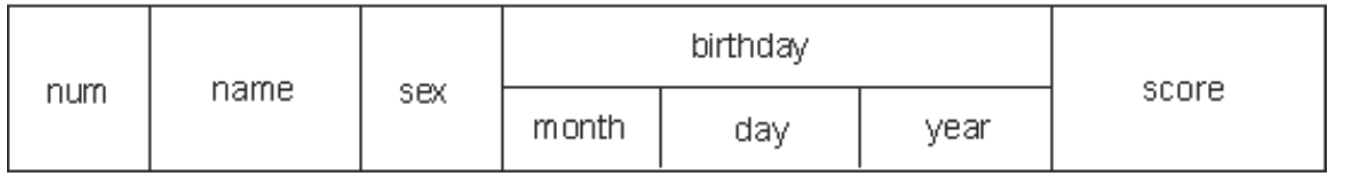• 注意:
• 结构体不可以嵌套自己变量,可以嵌套指向自己这种类型的指针
``````struct Student {
int age;
struct Student stu;
};``````
• 对嵌套结构体成员的访问
• 如果某个成员也是结构体变量，可以连续使用成员运算符"."访问最低一级成员
``````struct Date {
int year;
int month;
int day;
};

struct Student {
char *name;
struct Date birthday;
};

struct Student stu;
stu.birthday.year = 1986;
stu.birthday.month = 9;
stu.birthday.day = 10;``````

## 结构体和函数

• 结构体虽然是构造类型, 但是结构体之间赋值是值拷贝, 而不是地址传递
``````struct Person{
char *name;
int age;
};
struct Person p1 = {"lnj", 35};
struct Person p2;
p2 = p1;
p2.name = "zs"; // 修改p2不会影响p1
printf("p1.name = %s\n", p1.name); // lnj
printf("p2.name = %s\n", p2.name); //  zs``````
• 所以结构体变量作为函数形参时也是值传递, 在函数内修改形参, 不会影响外界实参
``````#include <stdio.h>

struct Person{
char *name;
int age;
};

void test(struct Person per);

int main()
{
struct Person p1 = {"lnj", 35};
printf("p1.name = %s\n", p1.name); // lnj
test(p1);
printf("p1.name = %s\n", p1.name); // lnj
return 0;
}
void test(struct Person per){
per.name = "zs";
}``````

## 共用体

• 和结构体不同的是, 结构体的每个成员都是占用一块独立的存储空间, 而共用体所有的成员都占用同一块存储空间
• 和结构体一样, 共用体在使用之前必须先定义共用体类型, 再定义共用体变量
• 定义共用体类型格式:
``````union 共用体名{
数据类型 属性名称;
数据类型 属性名称;
...   ....
};``````
• 定义共用体类型变量格式:
``union 共用体名 共用体变量名称;``
• 特点: 由于所有属性共享同一块内存空间, 所以只要其中一个属性发生了改变, 其它的属性都会受到影响
• 示例:
``````union Test{
int age;
char ch;
};
union Test t;
printf("sizeof(p) = %i\n", sizeof(t));

t.age = 33;
printf("t.age = %i\n", t.age); // 33
t.ch = 'a';
printf("t.ch = %c\n", t.ch); // a
printf("t.age = %i\n", t.age); // 97``````
• 共用体的应用场景
• （1）通信中的数据包会用到共用体，因为不知道对方会发送什么样的数据包过来，用共用体的话就简单了，定义几种格式的包，收到包之后就可以根据包的格式取出数据。
• （2）节约内存。如果有2个很长的数据结构，但不会同时使用，比如一个表示老师，一个表示学生，要统计老师和学生的情况，用结构体就比较浪费内存，这时就可以考虑用共用体来设计。
+（3）某些应用需要大量的临时变量，这些变量类型不同，而且会随时更换。而你的堆栈空间有限，不能同时分配那么多临时变量。这时可以使用共用体让这些变量共享同一个内存空间，这些临时变量不用长期保存，用完即丢，和寄存器差不多，不用维护。

## 枚举

• 什么是枚举类型?

• 在实际问题中,有些变量的取值被限定在一个有限的范围内。例如,一个星期内只有七天,一年只有十二个月,一个班每周有六门课程等等。如果把这些量说明为整型,字符型或其它类型 显然是不妥当的。
• C语言提供了一种称为“枚举”的类型。在“枚举”类型的定义中列举出所有可能的取值, 被说明为该“枚举”类型的变量取值不能超过定义的范围。
• 该说明的是,枚举类型是一种基本数据类型,而不是一种构造类型,因为它不能再分解为任何基本类型。• 枚举类型的定义

• 格式:
``````enum　枚举名　{
枚举元素1,
枚举元素2,
……
};``````
• 示例:
``````// 表示一年四季
enum Season {
Spring,
Summer,
Autumn,
Winter
};``````
• 枚举变量
• 先定义枚举类型，再定义枚举变量
``````enum Season {
Spring,
Summer,
Autumn,
Winter
};
enum Season s;``````
• 定义枚举类型的同时定义枚举变量
``````enum Season {
Spring,
Summer,
Autumn,
Winter
} s;``````
• 省略枚举名称，直接定义枚举变量
``````enum {
Spring,
Summer,
Autumn,
Winter
} s;``````
• 枚举类型变量的赋值和使用
``````enum Season {
Spring,
Summer,
Autumn,
Winter
} s;
s = Spring; // 等价于 s = 0;
s = 3; // 等价于 s = winter;
printf("%d", s);``````
• 枚举使用的注意
• C语言编译器会将枚举元素(spring、summer等)作为整型常量处理，称为枚举常量。
• 枚举元素的值取决于定义时各枚举元素排列的先后顺序。默认情况下，第一个枚举元素的值为0，第二个为1，依次顺序加1。
• 也可以在定义枚举类型时改变枚举元素的值
``````enum Season {
Spring,
Summer,
Autumn,
Winter
};
// 也就是说spring的值为0，summer的值为1，autumn的值为2，winter的值为3``````
``````enum Season {
Spring = 9,
Summer,
Autumn,
Winter
};
// 也就是说spring的值为9，summer的值为10，autumn的值为11，winter的值为12``````

## 全局变量和局部变量

• 变量作用域基本概念
• 变量作用域：变量的可用范围
• 按照作用域的不同，变量可以分为：局部变量和全局变量
• 局部变量
• 定义在函数内部的变量以及函数的形参, 我们称为局部变量
• 作用域：从定义的那一行开始, 直到遇到}结束或者遇到return为止
• 生命周期: 从程序运行到定义哪一行开始分配存储空间到程序离开该变量所在的作用域
• 存储位置: 局部变量会存储在内存的栈区中
• 特点：
• 相同作用域内不可以定义同名变量
• 不同作用范围可以定义同名变量，内部作用域的变量会覆盖外部作用域的变量
• 全局变量
• 定义在函数外面的变量称为全局变量
• 作用域范围：从定义哪行开始直到文件结尾
• 生命周期:程序一启动就会分配存储空间,直到程序结束
• 存储位置：静态存储区
• 特点: 多个同名的全局变量指向同一块存储空间

## auto和register关键字

• auto关键字(忘记)
• 只能修饰局部变量, 局部变量如果没有其它修饰符, 默认就是auto的
• 特点: 随用随开, 用完即销
``auto int num; // 等价于 int num;``
• register关键字(忘记)
• 只能修饰局部变量, 原则上将内存中变量提升到CPU寄存器中存储, 这样访问速度会更快
• 但是由于CPU寄存器数量相当有限, 通常不同平台和编译器在优化阶段会自动转换为auto
``register int num;``

## static关键字

• 对局部变量的作用
• 延长局部变量的生命周期,从程序启动到程序退出,但是它并没有改变变量的作用域
• 定义变量的代码在整个程序运行期间仅仅会执行一次
``````#include <stdio.h>
void test();
int main()
{
test();
test();
test();

return 0;
}
void test(){
static int num = 0; // 局部变量
num++;
// 如果不加static输出 1 1 1
// 如果添加static输出 1 2 3
printf("num = %i\n", num);
}``````
• 对全局变量的作用

• 全局变量分类：

• 内部变量:只能在本文件中访问的变量

• 外部变量:可以在其他文件中访问的变量,默认所有全局变量都是外部变量

• 默认情况下多个同名的全局变量共享一块空间, 这样会导致全局变量污染问题

• 如果想让某个全局变量只在某个文件中使用, 并且不和其他文件中同名全局变量共享同一块存储空间, 那么就可以使用static

``````// A文件中的代码
int num; // 和B文件中的num共享
void test(){
printf("ds.c中的 num = %i\n", num);
}``````
``````// B文件中的代码
#include <stdio.h>
#include "ds.h"

int num; // 和A文件中的num共享
int main()
{
num = 666;
test(); // test中输出666
return 0;
}``````
``````// A文件中的代码
static int num; // 不和B文件中的num共享
void test(){
printf("ds.c中的 num = %i\n", num);
}``````
``````// B文件中的代码
#include <stdio.h>
#include "ds.h"

int num; // 不和A文件中的num共享
int main()
{
num = 666;
test(); // test中输出0
return 0;
}``````

## extern关键字

• 对局部变量的作用
• extern不能用于局部变量
• extern代表声明一个变量, 而不是定义一个变量, 变量只有定义才会开辟存储空间
• 所以如果是局部变量, 虽然提前声明有某个局部变量, 但是局部变量只有执行到才会分配存储空间
``````#include <stdio.h>

int main()
{
extern int num;
num = 998; // 使用时并没有存储空间可用, 所以声明了也没用
int num; // 这里才会开辟
printf("num = %i\n", num);
return 0;
}``````
• 对全局变量的作用
• 声明一个全局变量, 代表告诉编译器我在其它地方定义了这个变量, 你可以放心使用
``````#include <stdio.h>

int main()
{
extern int num; // 声明我们有名称叫做num变量
num = 998; // 使用时已经有对应的存储空间
printf("num = %i\n", num);
return 0;
}
int num; // 全局变量, 程序启动就会分配存储空间``````

## static与extern对函数的作用

• 内部函数:只能在本文件中访问的函数
• 外部函数:可以在本文件中以及其他的文件中访问的函数
• 默认情况下所有的函数都是外部函数
• static 作用
• 声明一个内部函数
``static int sum(int num1,int num2);``
• 定义一个内部函数
``````static int sum(int num1,int num2)
{
return num1 + num2;
}``````
• extern作用
• 声明一个外部函数
``extern int sum(int num1,int num2);``
• 定义一个外部函数
``````extern int sum(int num1,int num2)
{
return num1 + num2;
}``````
• 注意点:
• 由于默认情况下所有的函数都是外部函数, 所以extern一般会省略
• 如果只有函数声明添加了static与extern, 而定义中没有添加static与extern, 那么无效

## Qt Creator编译过程做了什么?

•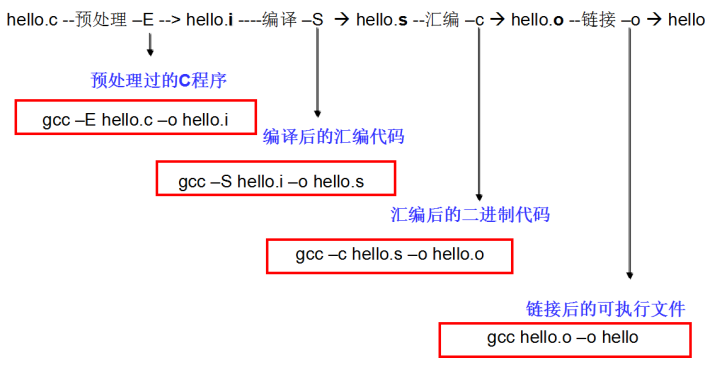• 当我们按下运行按钮的时, 其实Qt Creator编译器做了5件事情
• 对源文件进行预处理, 生成预处理文件
• 对预处理文件进行编译, 生成汇编文件
• 对汇编文件进行编译, 生成二进制文件
• 对二进制文件进行链接, 生成可执行文件
• 运行可执行文件

Qt Creator编译过程验证

• 1.编写代码, 保存源文件:
``````#include <stdio.h>
int main(){
printf("hello lnj\n");
return 0;
}``````

2.执行预处理编译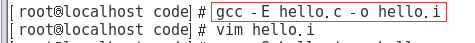• 执行预处理编译后生成的文件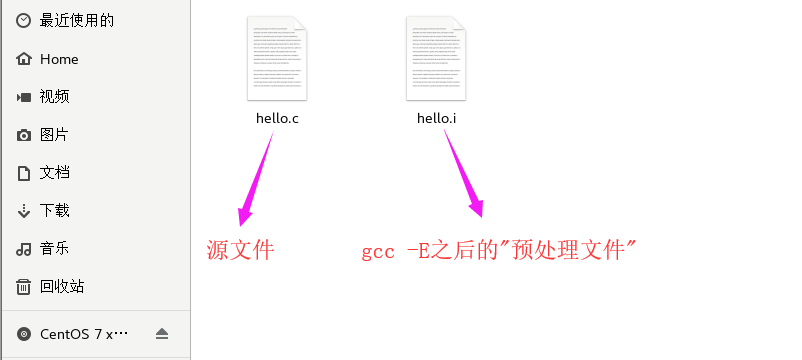• 处理源文件中预处理相关的指令

• 处理源文件中多余注释等
[外链图片转存中…(img-66BPT6H1-1648814825905)]

• 3.执行汇编编译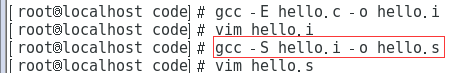• 执行汇编编译后生成的文件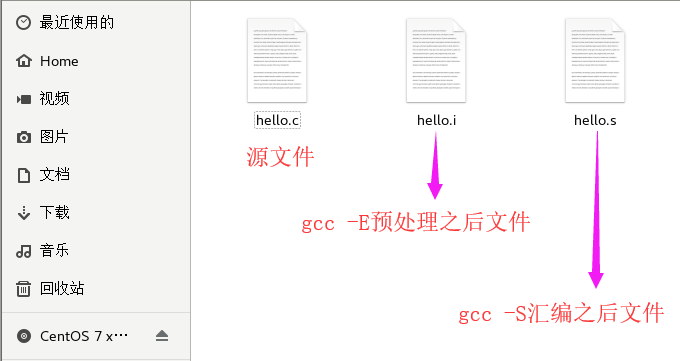• 打开汇编编译后生成的文件
[外链图片转存中…(img-UPPwxlFB-1648814825906)]

• 4.执行二进制编译
[外链图片转存中…(img-HeL6nIvy-1648814825906)]
• 执行二进制编译后生成的文件
[外链图片转存中…(img-9OTp8b2w-1648814825906)]
• 打开二进制编译后生成的文件
[外链图片转存中…(img-tmtp1Rr4-1648814825907)]

• 5.执行链接操作
• 将依赖的一些C语言函数库和我们编译好的二进制合并为一个文件
[外链图片转存中…(img-kvA4ZJwX-1648814825907)]
• 执行链接操作后生成的文件
[外链图片转存中…(img-ECOKJpk2-1648814825907)]

• 6.运行链接后生成的文件
[外链图片转存中…(img-kxKrlE7c-1648814825908)]

## 计算机是运算过程分析

• 1.编写一个简单的加法运算
• 2.调试编写好的代码, 查看对应的汇编文件
[外链图片转存中…(img-Ka0bxhr7-1648814825908)]
[外链图片转存中…(img-K5uGITsh-1648814825908)]
[外链图片转存中…(img-JXTyS91w-1648814825909)]
• 结论:
• 1.通过地址线找到对应地址的存储单元
• 2.通过控制线发送内存读取指令
• 3.通过数据线将内存中的值传输到CPU寄存器中
• 4.在CPU中完成计算操作
• 5.通过地址线找到对应地址的存储单元
• 6.通过控制线发送内存写入指令
• 7.通过数据线将计算结果传输到内存中

## 预处理指令的概念

• C语言在对源程序进行编译之前，会先对一些特殊的预处理指令作解释(比如之前使用的#include文件包含指令)，产生一个新的源程序(这个过程称为编译预处理),之后再进行通常的编译
• 为了区分预处理指令和一般的C语句，所有预处理指令都以符号“#”开头，并且结尾不用分号
• 预处理指令可以出现在程序的任何位置，它的作用范围是从它出现的位置到文件尾。习惯上我们尽可能将预处理指令写在源程序开头，这种情况下，它的作用范围就是整个源程序文件
• C语言提供了多种预处理功能,如宏定义、文件包含、条件编译等。合理地使用预处理功能编写的程序便于阅读、修改、移植和调试,也有利于模块化程序设计。

## 宏定义

• 被定义为“宏”的标识符称为“宏名”。在编译预处理时,对程序中所有出现的“宏名”,都用宏定义中的字符串去代换,这称为“宏代换”或“宏展开”。

• 宏定义是由源程序中的宏定义命令完成的。宏代换是由预处理程序自动完成的。在C语言中,“宏”分为有参数和无参数两种。
##不带参数的宏定义

• 格式:

``#define 标识符 字符串``
• 其中的“#”表示这是一条预处理命令。凡是以“#”开头的均为预处理命令。“define”为宏定义命令。“标识符”为所定义的宏名。“字符串”可以是常数、表达式、格式串等。
``````#include <stdio.h>

// 源程序中所有的宏名PI在编译预处理的时候都会被3.14所代替
#define PI 3.14

}

int main ()
{
float g = girth(2);

printf("周长为：%f", g);
return 0;
}``````
• 注意点:
1. 宏名一般用大写字母，以便与变量名区别开来，但用小写也没有语法错误
• 2)对程序中用双引号扩起来的字符串内的字符，不进行宏的替换操作
``````#define R 10
int main ()
{

return 0;
}``````
• 3.在编译预处理用字符串替换宏名时，不作语法检查，只是简单的字符串替换。只有在编译的时候才对已经展开宏名的源程序进行语法检查
``````#define I 100
int main ()
{
int i = I;
return 0;
}``````
• 4.宏名的有效范围是从定义位置到文件结束。如果需要终止宏定义的作用域，可以用#undef命令
``````#define PI 3.14
int main ()
{
printf("%f", PI);
return 0;
}
#undef PI
void test()
{
printf("%f", PI); // 不能使用
}``````
• 5.定义一个宏时可以引用已经定义的宏名
``````#define R  3.0
#define PI 3.14
#define L  2*PI*R
#define S  PI*R*R``````
• 6.可用宏定义表示数据类型,使书写方便
``````#define String char *
int main(int argc, const char * argv[])
{
String str = "This is a string!";
return 0;
}``````

## 带参数的宏定义

• C语言允许宏带有参数。在宏定义中的参数称为形式参数,在宏调用中的参数称为实际参数。对带参数的宏,在调用中,不仅要宏展开,而且要用实参去代换形参
• 格式: `#define 宏名(形参表) 字符串`
``````// 第1行中定义了一个带有2个参数的宏average，
#define average(a, b) (a+b)/2

int main ()
{
// 第4行其实会被替换成：int a = (10 + 4)/2;，
int a = average(10, 4);
// 输出结果为：7是不是感觉这个宏有点像函数呢？
printf("平均值：%d", a);
return 0;
}``````
• 注意点:
• 1)宏名和参数列表之间不能有空格，否则空格后面的所有字符串都作为替换的字符串.
``````#define average (a, b) (a+b)/2

int main ()
{
int a = average(10, 4);
return 0;
}

int a = (a, b) (a+b)/2(10, 4);

• 2)带参数的宏在展开时，只作简单的字符和参数的替换，不进行任何计算操作。所以在定义宏时，一般用一个小括号括住字符串的参数。
``````#include <stdio.h>
// 下面定义一个宏D(a)，作用是返回a的2倍数值：
#define D(a) 2*a
// 如果定义宏的时候不用小括号括住参数

int main ()
{
// 将被替换成int b = 2*3+4;，输出结果10，如果定义宏的时候用小括号括住参数，把上面的第3行改成：#define D(a) 2*(a)，注意右边的a是有括号的，第7行将被替换成int b = 2*(3+4);，输出结果14

int b = D(3+4);
printf("%d", b);
return 0;
}``````
• 3)计算结果最好也用括号括起来
``````#include <stdio.h>
// 下面定义一个宏P(a)，作用是返回a的平方
#define Pow(a) (a) * (a) // 如果不用小括号括住计算结果

int main(int argc, const char * argv[])      {
// 代码被替换为:int b = (10) * (10) / (2) * (2);
// 简化之后：int b = 10 * (10 / 2) * 2;，最后变量b为:100
int b = Pow(10) / Pow(2);

printf("%d", b);
return 0;
}``````
``````#include <stdio.h>
// 计算结果用括号括起来
#define Pow(a) ( (a) * (a) )

int main(int argc, const char * argv[])      {
// 代码被替换为:int b = ( (10) * (10) ) / ( (2) * (2) );
// 简化之后：int b = (10 * 10) / (2 *2);，最后输出结果：25
int b = Pow(10) / Pow(2);

printf("%d", b);
return 0;
}``````

## 条件编译

• 在很多情况下，我们希望程序的其中一部分代码只有在满足一定条件时才进行编译，否则不参与编译(只有参与编译的代码最终才能被执行)，这就是条件编译。
• 为什么要使用条件编译
• 1)按不同的条件去编译不同的程序部分,因而产生不同的目标代码文件。有利于程序的移植和调试。
• 2)条件编译当然也可以用条件语句来实现。 但是用条件语句将会对整个源程序进行编译,生成 的目标代码程序很长,而采用条件编译,则根据条件只编译其中的程序段1或程序段2,生成的目 标程序较短。
##if-#else 条件编译指令
• 第一种格式:
• 它的功能是,如常量表达式的值为真(非0),则将code1 编译到程序中,否则对code2编译到程序中。
• 注意:
• 是将代码编译进可执行程序, 而不是执行代码
• 条件编译后面的条件表达式中不能识别变量,它里面只能识别常量和宏定义
``````#if 常量表达式
..code1...
#else
..code2...
#endif``````
``````#define SCORE 67
#if SCORE > 90
printf("优秀\n");
#else
printf("不及格\n");
#endif``````
• 第二种格式:
``````#if 条件1
...code1...
#elif 条件2
...code2...
#else
...code3...
#endif``````
``````#define SCORE 67
#if SCORE > 90
printf("优秀\n");
#elif SCORE > 60
printf("良好\n");
#else
printf("不及格\n");
#endif``````

## typedef关键字

• C语言不仅􏰀供了丰富的数据类型,而且还允许由用户自己定义类型说明符,也就是说允许由用户为数据类型取“别名”。

• 格式:

``typedef 原类型名 新类型名;``
• 其中原类型名中含有定义部分,新类型名一般用大写表示,以便于区别。
• 有时也可用宏定义来代替typedef的功能,但是宏定义是由预处理完成的,而typedef则是在编译 时完成的,后者更为灵活方便。
##typedef使用
• 基本数据类型

``````typedef int INTEGER
INTEGER a; // 等价于 int a;``````
• 也可以在别名的基础上再起一个别名
``````typedef int Integer;

typedef Integer MyInteger;``````
• 用typedef定义数组、指针、结构等类型将带来很大的方便,不仅使程序书写简单而且使意义更为 明确,因而增强了可读性。
• 数组类型
``````typedef char NAME; // 表示NAME是字符数组类型,数组长度为20。然后可用NAME 说明变量,
NAME a; // 等价于 char a;``````
• 结构体类型
• 第一种形式:
`````` struct Person{
int age;
char *name;
};

typedef struct Person PersonType;``````
``+ 第二种形式:``
``````typedef struct Person{
int age;
char *name;
} PersonType;``````
``+ 第三种形式:``
``````typedef struct {
int age;
char *name;
} PersonType;``````
• 枚举
• 第一种形式:
``````enum Sex{
SexMan,
SexWoman,
SexOther
};
typedef enum Sex SexType;``````
``+ 第二种形式:``
``````typedef enum Sex{
SexMan,
SexWoman,
SexOther
} SexType;``````
``+ 第三种形式:``
``````typedef enum{
SexMan,
SexWoman,
SexOther
} SexType;``````
• 指针
• typedef与指向结构体的指针
``````// 定义一个结构体并起别名
typedef struct {
float x;
float y;
} Point;

// 起别名
typedef Point *PP;``````
• typedef与指向函数的指针
``````// 定义一个sum函数，计算a跟b的和
int sum(int a, int b) {
int c = a + b;
printf("%d + %d = %d", a, b, c);
return c;
}
typedef int (*MySum)(int, int);

// 定义一个指向sum函数的指针变量p
MySum p = sum;``````

## 宏定义与函数以及typedef区别

• 与函数的区别
• 从整个使用过程可以发现，带参数的宏定义，在源程序中出现的形式与函数很像。但是两者是有本质区别的：
• 1> 宏定义不涉及存储空间的分配、参数类型匹配、参数传递、返回值问题
• 2> 函数调用在程序运行时执行，而宏替换只在编译预处理阶段进行。所以带参数的宏比函数具有更高的执行效率
• typedef和#define的区别
• 用宏定义表示数据类型和用typedef定义数据说明符的区别。
• 宏定义只是简单的字符串替换,￼是在预处理完成的
• typedef是在编译时处理的,它不是作简单的代换,而是对类型说明符￼重新命名。被命名的标识符具有类型定义说明的功能
``````typedef char *String;
int main(int argc, const char * argv[])
{
String str = "This is a string!";
return 0;
}

#define String char *
int main(int argc, const char * argv[])
{
String str = "This is a string!";
return 0;
}``````
``````typedef char *String1; // 给char *起了个别名String1
#define String2 char * // 定义了宏String2
int main(int argc, const char * argv[]) {
/*
只有str1、str2、str3才是指向char类型的指针变量
由于String1就是char *，所以上面的两行代码等于:
char *str1;
char *str2;
*/
String1 str1, str2;
/*
宏定义只是简单替换, 所以相当于
char *str3, str4;
*号只对最近的一个有效, 所以相当于
char *str3;
char str4;
*/
String2 str3, str4;
return 0;
}``````

## const关键字

• const是一个类型修饰符
• 使用const修饰变量则可以让变量的值不能改变
##const有什么主要的作用?
• (1)可以定义const常量,具有不可变性
``````const int Max=100;
int Array[Max];``````
• (2)便于进行类型检查,使编译器对处理内容有更多了解,消除了一些隐患。
``void f(const int i) { .........}``
• 编译器就会知道i是一个常量,不允许修改;
• (3)可以避免意义模糊的数字出现,同样可以很方便地进行参数的调整和修改。 同宏定义一样,可以做到不变则已,一变都变!如(1)中,如果想修改Max的内容,只需要:const int Max=you want;即可!
• (4)可以保护被修饰的东西,防止意外的修改,增强程序的健壮性。 还是上面的例子,如果在 函数体内修改了i,编译器就会报错;
``void f(const int i) { i=10;//error! }``
• (5) 可以节省空间,避免不必要的内存分配。
``````#define PI 3.14159 //常量宏
const doulbe Pi=3.14159; //此时并未将Pi放入ROM中 ...... double i=Pi; //此时为Pi分配内存,以后不再分配!
double I=PI; //编译期间进行宏替换,分配内存
double j=Pi; //没有内存分配
double J=PI; //再进行宏替换,又一次分配内存! const定义常量从汇编的角度来看,只是给出了对应的内存地址,而不是象#define一样给出的是立即数,所以,const定义的常量在程序运行过程中只有一份拷贝,而#define定义的常量在内存 中有若干个拷贝。``````
• (6) 􏰀高了效率。编译器通常不为普通const常量分配存储空间,而是将它们保存在符号表 中,这使得它成为一个编译期间的常量,没有了存储与读内存的操作,使得它的效率也很高。

## 如何使用const?

• (1)修饰一般常量一般常量是指简单类型的常量。这种常量在定义时,修饰符const可以用在类型说明符前,也可以用在类型说明符后
``int const x=2; 或 const int x=2;``
• (当然,我们可以偷梁换柱进行更新: 通过强制类型转换,将地址赋给变量,再作修改即可以改变const常量值。)
``````// const对于基本数据类型, 无论写在左边还是右边, 变量中的值不能改变
const int a = 5;
// a = 666; // 直接修改会报错
// 偷梁换柱, 利用指针指向变量
int *p;
p = &a;
// 利用指针间接修改变量中的值
*p = 10;
printf("%d\n", a);
printf("%d\n", *p);``````
• (2)修饰常数组(值不能够再改变了)定义或说明一个常数组可采用如下格式:
``````int const a={1, 2, 3, 4, 5};
const int a={1, 2, 3, 4, 5};``````
``````const int a={1, 2, 3, 4, 5};
a = 55; // 错误``````
• (3)修饰函数的常参数const修饰符也可以修饰函数的传递参数,格式如下:void Fun(const int Var); 告诉编译器Var在函数体中的无法改变,从而防止了使用者的一些无 意的或错误的修改。
• (4)修饰函数的返回值: const修饰符也可以修饰函数的返回值,是返回值不可被改变,格式如 下:
``````const int Fun1();
const MyClass Fun2();``````
• (5)修饰常指针
• const int *A; //const修饰指针,A可变,A指向的值不能被修改
• int const *A; //const修饰指向的对象,A可变,A指向的对象不可变
• int *const A; //const修饰指针A, A不可变,A指向的对象可变
• const int *const A;//指针A和A指向的对象都不可变
• 技巧
``````先看“*”的位置

## 进程空间

• 程序，是经源码编译后的可执行文件，可执行文件可以多次被执行，比如我们可以多次打开 office。
• 而进程，是程序加载到内存后开始执行，至执行结束，这样一段时间概念，多次打开的wps,每打开一次都是一个进程，当我们每关闭一个 office，则表示该进程结束。
• 程序是静态概念，而进程动态/时间概念。
###进程空间图示
有了进程和程序的概念以后，我们再来看一下，程序被加载到内存以后内存空间布局是什么样的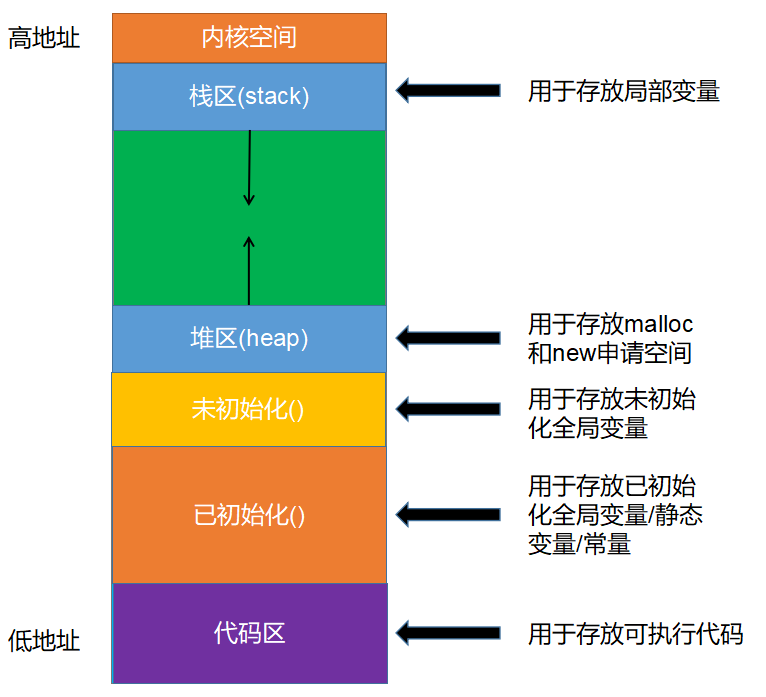## 栈内存(Stack)

• 栈中存放任意类型的变量，但必须是 auto 类型修饰的，即自动类型的局部变量， 随用随开，用完即消。
• 内存的分配和销毁系统自动完成，不需要人工干预
• 栈的最大尺寸固定，超出则引起栈溢出
• 局部变量过多，过大 或 递归层数太多等就会导致栈溢出
``int ages[10240*10240]; // 程序会崩溃, 栈溢出``
``````#include <stdio.h>

int main()
{
// 存储在栈中, 内存地址从大到小
int a = 10;
int b = 20;
printf("&a = %p\n", &a); // &a = 0060FEAC
printf("&b = %p\n", &b); // &b = 0060FEA8

return 0;
}``````

## 堆内存(Heap)

• 堆内存可以存放任意类型的数据，但需要自己申请与释放
• 堆大小，想像中的无穷大，但实际使用中，受限于实际内存的大小和内存是否连续性
``int *p = (int *)malloc(10240 * 1024); // 不一定会崩溃``
``````#include <stdio.h>
#include <stdlib.h>

int main()
{
// 存储在栈中, 内存地址从小到大
int *p1 = malloc(4);
*p1 = 10;
int *p2 = malloc(4);
*p2 = 20;

printf("p1 = %p\n", p1); //  p1 = 00762F48
printf("p2 = %p\n", p2); // p2 = 00762F58

return 0;
}``````

## malloc函数

``````#include <stdio.h>
#include <stdlib.h>
#include <string.h>

int main()
{
/*
* malloc
* 第一个参数: 需要申请多少个字节空间
* 返回值类型: void *
*/
int *p = (int *)malloc(sizeof(int));
printf("p = %i\n", *p); // 保存垃圾数据
/*
* 第一个参数: 需要初始化的内存地址
* 第二个初始: 需要初始化的值
* 第三个参数: 需要初始化对少个字节
*/
memset(p, 0, sizeof(int)); // 对申请的内存空间进行初始化
printf("p = %i\n", *p); // 初始化为0
return 0;
}``````

## free函数

• 注意: 通过malloc申请的存储空间一定要释放, 所以malloc和free函数总是成对出现

``````#include <stdio.h>
#include <stdlib.h>
#include <string.h>

int main()
{
// 1.申请4个字节存储空间
int *p = (int *)malloc(sizeof(int));
// 2.初始化4个字节存储空间为0
memset(p, 0, sizeof(int));
// 3.释放申请的存储空间
free(p);
return 0;
}``````

## calloc函数

``````#include <stdio.h>
#include <stdlib.h>
#include <string.h>

int main()
{
/*
// 1.申请3块4个字节存储空间
int *p = (int *)malloc(sizeof(int) * 3);
// 2.使用申请好的3块存储空间
p = 1;
p = 3;
p = 5;
printf("p = %i\n", p);
printf("p = %i\n", p);
printf("p = %i\n", p);
// 3.释放空间
free(p);
*/

// 1.申请3块4个字节存储空间
int *p = calloc(3, sizeof(int));
// 2.使用申请好的3块存储空间
p = 1;
p = 3;
p = 5;
printf("p = %i\n", p);
printf("p = %i\n", p);
printf("p = %i\n", p);
// 3.释放空间
free(p);

return 0;
}``````

## realloc函数

• 注意点:
• 若参数ptr==NULL，则该函数等同于 malloc
• 返回的指针，可能与 ptr 的值相同，也有可能不同。若相同，则说明在原空间后面申请，否则，则可能后续空间不足，重新申请的新的连续空间，原数据拷贝到新空间， 原有空间自动释放
``````#include <stdio.h>
#include <stdlib.h>
#include <string.h>

int main()
{
// 1.申请4个字节存储空间
int *p = NULL;
p = realloc(p, sizeof(int)); // 此时等同于malloc
// 2.使用申请好的空间
*p = 666;
printf("*p = %i\n",  *p);
// 3.释放空间
free(p);

return 0;
}``````
``````#include <stdio.h>
#include <stdlib.h>
#include <string.h>

int main()
{
// 1.申请4个字节存储空间
int *p = malloc(sizeof(int));
printf("p = %p\n", p);
// 如果能在传入存储空间地址后面扩容, 返回传入存储空间地址
// 如果不能在传入存储空间地址后面扩容, 返回一个新的存储空间地址
p = realloc(p, sizeof(int) * 2);
printf("p = %p\n", p);
// 2.使用申请好的空间
*p = 666;
printf("*p = %i\n",  *p);
// 3.释放空间
free(p);

return 0;
}``````

## 链表

• 链表实现了，内存零碎数据的有效组织。比如，当我们用 malloc 来进行内存申请的时候，当内存足够，但是由于碎片太多，没有连续内存时，只能以申请失败而告终，而用链表这种数据结构来组织数据，就可以解决上类问题。
[外链图片转存中…(img-wcaQsM5W-1648814825910)]

## 静态链表

[外链图片转存中…(img-zwaxM2zR-1648814825910)]

``````#include <stdio.h>
#include <stdlib.h>
#include <string.h>

// 1.定义链表节点
typedef struct node{
int data;
struct node *next;
}Node;
int main()
{

// 2.创建链表节点
Node a;
Node b;
Node c;

// 3.初始化节点数据
a.data = 1;
b.data = 3;
c.data = 5;

// 4.链接节点
a.next = &b;
b.next = &c;
c.next = NULL;

// 5.创建链表头

// 6.使用链表
printf("currentData = %i\n", currentData);
}
return 0;
}``````

## 动态链表

• 静态链表的意义不是很大，主要原因，数据存储在栈上，栈的存储空间有限，不能动态分配。所以链表要实现存储的自由，要动态的申请堆里的空间。
• 有一个点要说清楚，我们的实现的链表是带头节点。至于，为什么带头节点，需等大家对链表有个整体的的认知以后，再来体会，会更有意义。
• 空链表
• 头指针带了一个空链表节点, 空链表节点中的next指向NULL
[外链图片转存中…(img-Avlo3vjr-1648814825910)]
``````#include <stdio.h>
#include <stdlib.h>

// 1.定义链表节点
typedef struct node{
int data;
struct node *next;
}Node;
int main()
{
return 0;
}
// 创建空链表
Node *createList(){
// 1.创建一个节点
Node *node = (Node *)malloc(sizeof(Node));
if(node == NULL){
exit(-1);
}
// 2.设置下一个节点为NULL
node->next = NULL;
// 3.返回创建好的节点
return node;
}``````

• 头指针带了一个非空节点, 最后一个节点中的next指向NULL
[外链图片转存中…(img-jIY1Xw5f-1648814825911)]

## 动态链表头插法

• 1.让新节点的下一个节点等于头结点的下一个节点
• 2.让头节点的下一个节点等于新节点
``````#include <stdio.h>
#include <stdlib.h>

// 1.定义链表节点
typedef struct node{
int data;
struct node *next;
}Node;
Node *createList();
void printNodeList(Node *node);
int main()
{
return 0;
}
/**
* @brief createList 创建链表
* @return  创建好的链表
*/
Node *createList(){
// 1.创建头节点
return NULL;
}

// 2.接收用户输入数据
int num = -1;
printf("请输入节点数据\n");
scanf("%i", &num);

// 3.通过循环创建其它节点
while(num != -1){
// 3.1创建一个新的节点
Node *cur = (Node *)malloc(sizeof(Node));
cur->data = num;

// 3.2让新节点的下一个节点指向头节点的下一个节点
// 3.3让头节点的下一个节点指向新节点

// 3.4再次接收用户输入数据
scanf("%i", &num);
}

// 3.返回创建好的节点
}
/**
* @brief printNodeList 遍历链表
* @param node 链表指针头
*/
void printNodeList(Node *node){
printf("currentData = %i\n", currentData);
}
}``````

## 动态链表尾插法

• 1.定义变量记录新节点的上一个节点
• 2.将新节点添加到上一个节点后面
• 3.让新节点成为下一个节点的上一个节点
``````#include <stdio.h>
#include <stdlib.h>

// 1.定义链表节点
typedef struct node{
int data;
struct node *next;
}Node;
Node *createList();
void printNodeList(Node *node);
int main()
{
return 0;
}
/**
* @brief createList 创建链表
* @return  创建好的链表
*/
Node *createList(){
// 1.创建头节点
return NULL;
}

// 2.接收用户输入数据
int num = -1;
printf("请输入节点数据\n");
scanf("%i", &num);

// 3.通过循环创建其它节点
// 定义变量记录上一个节点
while(num != -1){
// 3.1创建一个新的节点
Node *cur = (Node *)malloc(sizeof(Node));
cur->data = num;

// 3.2让新节点链接到上一个节点后面
pre->next = cur;
// 3.3当前节点下一个节点等于NULL
cur->next = NULL;
// 3.4让当前节点编程下一个节点的上一个节点
pre = cur;

// 3.5再次接收用户输入数据
scanf("%i", &num);
}

// 3.返回创建好的节点
}
/**
* @brief printNodeList 遍历链表
* @param node 链表指针头
*/
void printNodeList(Node *node){
printf("currentData = %i\n", currentData);
}
}``````

## 动态链优化

``````#include <stdio.h>
#include <stdlib.h>

// 1.定义链表节点
typedef struct node{
int data;
struct node *next;
}Node;
Node *createList();
void printNodeList(Node *node);
int main()
{
// 1.创建一个空链表
// 2.往空链表中插入数据
return 0;
}
/**
* @brief createList 创建空链表
* @return  创建好的空链表
*/
Node *createList(){
// 1.创建头节点
return NULL;
}
// 3.返回创建好的节点
}
/**
* @brief insertNode1 尾插法插入节点
* @param data 需要插入的数据
* @return  插入之后的链表
*/
// 1.定义变量记录最后一个节点
while(pre != NULL && pre->next != NULL){
pre = pre->next;
}
// 2.创建一个新的节点
Node *cur = (Node *)malloc(sizeof(Node));
cur->data = data;

// 3.让新节点链接到上一个节点后面
pre->next = cur;
// 4.当前节点下一个节点等于NULL
cur->next = NULL;
// 5.让当前节点编程下一个节点的上一个节点
pre = cur;
}
/**
* @brief insertNode1 头插法插入节点
* @param data 需要插入的数据
* @return  插入之后的链表
*/
// 1.创建一个新的节点
Node *cur = (Node *)malloc(sizeof(Node));
cur->data = data;

// 2.让新节点的下一个节点指向头节点的下一个节点
// 3.让头节点的下一个节点指向新节点
}
/**
* @brief printNodeList 遍历链表
* @param node 链表指针头
*/
void printNodeList(Node *node){
printf("currentData = %i\n", currentData);
}
}``````

## 链表销毁

``````/**
* @brief destroyList 销毁链表
*/
Node *cur = NULL;
}
}``````

## 链表长度计算

``````/**
* @brief listLength 计算链表长度
* @return 链表长度
*/
int count = 0;
count++;
}
return count;
}``````

## 链表查找

``````/**
* @brief searchList 查找指定节点
* @param key 需要查找的值
* @return
*/
break;
}else{
}
}
}``````

## 链表查找

``````/**
* @brief searchList 查找指定节点
* @param key 需要查找的值
* @return
*/
break;
}else{
}
}
}``````

## 链表删除

``````void deleteNodeList(Node *head, Node *find){
}
free(find);
}``````

## 作业

• 给链表排序
``````/**
* @brief bubbleSort 对链表进行排序
*/
// 1.计算链表长度
// 2.定义变量记录前后节点
Node *cur = NULL;
// 3.相邻元素进行比较, 进行冒泡排序
for(int i = 0; i < len - 1; i++){
for(int j = 0; j < len - 1 - i; j++){
printf("%i, %i\n", cur->data, cur->next->data);
if((cur->data) > (cur->next->data)){
int temp = cur->data;
cur->data = cur->next->data;
cur->next->data = temp;
}
cur = cur->next;
}
}
}``````
``````/**
* @brief sortList 对链表进行排序
*/
// 0.计算链表长度
// 1.定义变量保存前后两个节点
Node *sh, *pre, *cur;
for(int i = 0; i < len - 1; i ++){
pre = sh->next; // 第一个节点
cur = pre->next; // 第二个节点
for(int j = 0; j < len - 1 - i; j++){
if(pre->data > cur->data){
// 交换节点位置
sh->next = cur;
pre->next = cur->next;
cur->next = pre;
// 恢复节点名称
Node *temp = pre;
pre = cur;
cur = temp;
}
// 让所有节点往后移动
sh = sh->next;
pre = pre->next;
cur = cur->next;
}
}
}``````
• 链表反转
``````/**
* @brief reverseList 反转链表
*/
// 1.将链表一分为二
Node *pre, *cur;
// 2.重新插入节点
while(pre){
cur = pre->next;

pre = cur;
}
}``````

## 文件基本概念

• 文件流:
• C 语言把文件看作是一个字符的序列，即文件是由一个一个字符组成的字符流，因此 c 语言将文件也称之为文件流。
• 文件分类
• 文本文件
• 以 ASCII 码格式存放，一个字节存放一个字符`文本文件的每一个字节存放一个 ASCII 码，代表一个字符`。这便于对字符的逐个处理，但占用存储空间
较多，而且要花费时间转换。
• .c文件就是以文本文件形式存放的
• 二进制文件
• 以补码格式存放。二进制文件是把数据以二进制数的格式存放在文件中的，其占用存储空间较少。`数据按其内存中的存储形式原样存放`
• .exe文件就是以二进制文件形式存放的

• 文本文件和二进制文件示例
• 下列代码暂时不要求看懂, 主要理解什么是文本文件什么是二进制文件
``````#include <stdio.h>

int main()
{
/*
* 以文本形式存储
* 会将每个字符先转换为对应的ASCII,
* 然后再将ASCII码的二进制存储到计算机中
*/
int num = 666;
FILE *fa = fopen("ascii.txt", "w");
fprintf(fa, "%d", num);
fclose(fa);

/*
* 以二进制形式存储
* 会将666的二进制直接存储到文件中
*/
FILE *fb = fopen("bin.txt", "w");
fwrite(&num, 4, 1, fb);
fclose(fb);

return 0;
}``````
• 内存示意图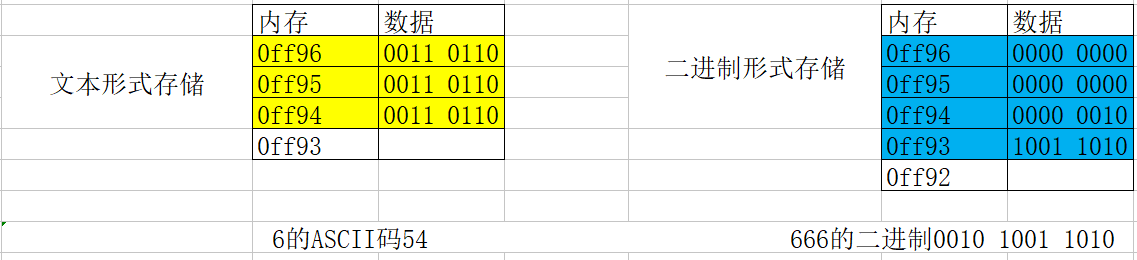• 通过文本工具打开示意图
[外链图片转存中…(img-U0dBDJz0-1648814825912)]
• 文本工具默认会按照ASCII码逐个直接解码文件, 由于文本文件存储的就是ASCII码, 所以可以正常解析显示, 由于二进制文件存储的不是ASCII码, 所以解析出来之后是乱码

## 文件的打开和关闭

• FILE 结构体
• FILE 结构体是对缓冲区和文件读写状态的记录者，所有对文件的操作，都是通过FILE 结构体完成的。
``````struct _iobuf {
char *_ptr;  //文件输入的下一个位置
int _cnt;  //当前缓冲区的相对位置
char *_base; //文件的起始位置)
int _flag; //文件标志
int _file;  //文件的有效性验证
int _charbuf; //检查缓冲区状况,如果无缓冲区则不读取
int _bufsiz; // 缓冲区大小
char *_tmpfname; //临时文件名
};
typedef struct _iobuf FILE;``````
• fileopen函数

mode 处理方式 当文件不存在时 当文件存在时 向文件输入 从文件输出
r 读取 出错 打开文件 不能 可以
w 写入 建立新文件 覆盖原有文件 可以 不能
a 追加 建立新文件 在原有文件后追加 可以 不能
r+ 读取/写入 出错 打开文件 可以 可以
w+ 写入/读取 建立新文件 覆盖原有文件 可以 可以
a++ 读取/追加 建立新文件 在原有文件后追加 可以 可以

• Windows如果读写的是二进制文件，则还要加 b,比如 rb, r+b 等。 unix/linux 不区分文本和二进制文件
• fclose函数

``````#include <stdio.h>

int main()
{
FILE *fp = fopen("test.txt", "w+");
fclose(fp);
return 0;
}``````

## 一次读写一个字符

• 写入

``````#include <stdio.h>

int main()
{
// 1.打开一个文件
FILE *fp = fopen("test.txt", "w+");

// 2.往文件中写入内容
for(char ch = 'a'; ch <= 'z'; ch++){
// 一次写入一个字符
char res = fputc(ch, fp);
printf("res = %c\n", res);
}

// 3.关闭打开的文件
fclose(fp);
return 0;
}``````
• 读取

``````#include <stdio.h>

int main()
{
// 1.打开一个文件
FILE *fp = fopen("test.txt", "r+");

// 2.从文件中读取内容
char res = EOF;
while((res = fgetc(fp)) != EOF){
printf("res = %c\n", res);
}

// 3.关闭打开的文件
fclose(fp);
return 0;
}``````
• 判断文件末尾
• feof函数

``````#include <stdio.h>

int main()
{
// 1.打开一个文件
FILE *fp = fopen("test.txt", "r+");

// 2.从文件中读取内容
char res = EOF;
// 注意: 由于只有先读了才会修改标志位,
// 所以通过feof判断是否到达文件末尾, 一定要先读再判断, 不能先判断再读
while((res = fgetc(fp)) && (!feof(fp))){
printf("res = %c\n", res);
}

// 3.关闭打开的文件
fclose(fp);
return 0;
}``````
• 注意点:
• feof 这个函数，是去读标志位判断文件是否结束的。
• 而标志位只有读完了才会被修改, 所以如果先判断再读标志位会出现多打一次的的现象
• 所以企业开发中使用feof函数一定要先读后判断, 而不能先判断后读
• 作业
• 实现文件的简单加密和解密
``````#include <stdio.h>
#include <string.h>
void encode(char *name, char *newName, int code);
void decode(char *name, char *newName, int code);
int main()
{
encode("main.c", "encode.c", 666);
decode("encode.c", "decode.c", 666);
return 0;
}
/**
* @brief encode 加密文件
* @param name 需要加密的文件名称
* @param newName 加密之后的文件名称
* @param code 秘钥
*/
void encode(char *name, char *newName, int code){
FILE *fw = fopen(newName, "w+");
FILE *fr = fopen(name, "r+");
char ch = EOF;
while((ch = fgetc(fr)) && (!feof(fr))){
fputc(ch ^ code, fw);
}
fclose(fw);
fclose(fr);
}
/**
* @brief encode 解密文件
* @param name 需要解密的文件名称
* @param newName 解密之后的文件名称
* @param code 秘钥
*/
void decode(char *name, char *newName, int code){
FILE *fw = fopen(newName, "w+");
FILE *fr = fopen(name, "r+");
char ch = EOF;
while((ch = fgetc(fr)) && (!feof(fr))){
fputc(ch ^ code, fw);
}
fclose(fw);
fclose(fr);
}``````

## 一次读写一行字符

• 什么是行

• 行是文本编辑器中的概念，文件流中就是一个字符。这个在不同的平台是有差异的。window 平台 ‘\r\n’，linux 平台是’\n’

• 平台差异

• windows 平台在写入’\n’是会体现为’\r\n’，linux 平台在写入’\n’时会体现为’\n’。windows 平台在读入’\r\n’时，体现为一个字符’\n’，linux 平台在读入’\n’时，体现为一个字符’\n’
• linux 读 windows 中的换行，则会多读一个字符，windows 读 linux 中的换行，则没有问题
``````#include <stdio.h>

int main()
{
FILE *fw = fopen("test.txt", "w+");
fputc('a', fw);
fputc('\n', fw);
fputc('b', fw);
fclose(fw);
return 0;
}``````

[外链图片转存中…(img-YHwGq2tR-1648814825912)]

• 写入一行

``````#include <stdio.h>

int main()
{
FILE *fw = fopen("test.txt", "w+");
// 注意: fputs不会自动添加\n
fputs("lnj\n", fw);
fputs("it666\n", fw);
fclose(fw);
return 0;
}``````
• 遇到\0自动终止写入
``````#include <stdio.h>

int main()
{
FILE *fp = fopen("test.txt", "w+");
// 注意: fputs写入时遇到\0就会自动终止写入
fputs("lnj\0it666\n", fp);

fclose(fp);
return 0;
}``````
• 读取一行

• 最多只能读取N-1个字符
``````#include <stdio.h>

int main()
{
FILE *fp = fopen("test.txt", "w+");
// 注意: fputs不会自动添加\n
fputs("it666\n", fp);

// 将FILE结构体中的读写指针重新移动到最前面
// 注意: FILE结构体中读写指针每读或写一个字符后都会往后移动
rewind(fp);
char str;
// 从fp中读取4个字符, 存入到str中
// 最多只能读取N-1个字符, 会在最后自动添加\0
fgets(str, 4, fp);

printf("str = %s", str); // it6
fclose(fp);
return 0;
}``````
• 遇到\n自动结束
``````#include <stdio.h>
int main()
{
FILE *fp = fopen("test.txt", "w+");
// 注意: fputs不会自动添加\n
fputs("lnj\n", fp);
fputs("it666\n", fp);

// 将FILE结构体中的读写指针重新移动到最前面
// 注意: FILE结构体中读写指针每读或写一个字符后都会往后移动
rewind(fp);
char str;
// 从fp中读取1024个字符, 存入到str中
// 但是读到第4个就是\n了, 函数会自动停止读取
// 注意点: \n会被读取进来
fgets(str, 1024, fp);

printf("str = %s", str); // lnj
fclose(fp);
return 0;
}``````
• 读取到EOF自动结束
``````#include <stdio.h>

int main()
{
FILE *fp = fopen("test.txt", "w+");
// 注意: fputs不会自动添加\n
fputs("lnj\n", fp);
fputs("it666", fp);

// 将FILE结构体中的读写指针重新移动到最前面
// 注意: FILE结构体中读写指针每读或写一个字符后都会往后移动
rewind(fp);
char str;
// 每次从fp中读取1024个字符, 存入到str中
// 读取到文件末尾自动结束
while(fgets(str, 1024, fp)){
printf("str = %s", str);
}
fclose(fp);
return 0;
}``````
• 注意点:
• 企业开发中能不用feof函数就不用feof函数
• 如果最后一行，没有行‘\n’的话则少读一行
``````#include <stdio.h>

int main()
{
FILE *fp = fopen("test.txt", "w+");
// 注意: fputs不会自动添加\n
fputs("12345678910\n", fp);
fputs("12345678910\n", fp);
fputs("12345678910", fp);

// 将FILE结构体中的读写指针重新移动到最前面
// 注意: FILE结构体中读写指针每读或写一个字符后都会往后移动
rewind(fp);
char str;
// 每次从fp中读取1024个字符, 存入到str中
// 读取到文件末尾自动结束
while(fgets(str, 1024, fp) && !feof(fp)){
printf("str = %s", str);
}
fclose(fp);
return 0;
}``````
• 作业:
• 利用fgets(str, 5, fp)读取下列文本会读取多少次?
``````12345678910
12345
123``````

## 一次读写一块数据

• C 语言己经从接口的层面区分了，文本的读写方式和二进制的读写方式。前面我们讲的是文本的读写方式。

• 所有的文件接口函数，要么以 ‘\0’，表示输入结束，要么以 ‘\n’， EOF(0xFF)表示读取结束。 ‘\0’ ‘\n’ 等都是文本文件的重要标识，而所有的二进制接口对于这些标识，是不敏感的。
+二进制的接口可以读文本，而文本的接口不可以读二进制

• 一次写入一块数据

int num_bytes: 每个要写的字段的字节数count
int count : 要写的字段的个数
FILE* fp : 要写的文件指针

``````#include <stdio.h>
#include <string.h>

int main()
{
FILE *fp = fopen("test.txt", "wb+");
// 注意: fwrite不会关心写入数据的格式
char *str = "lnj\0it666";
/*
* 第一个参数: 被写入数据指针
* 第二个参数: 每次写入多少个字节
* 第三个参数: 需要写入多少次
* 第四个参数: 已打开文件结构体指针
*/
fwrite((void *)str, 9, 1, fp);

fclose(fp);
return 0;
}``````
• 一次读取一块数据

int num_bytes: 每个要读的字段的字节数count
int count : 要读的字段的个数
FILE* fp : 要读的文件指针

``````#include <stdio.h>

int main()
{
// test.txt中存放的是"lnj\0it666"
FILE *fr = fopen("test.txt", "rb+");
char buf = {0};
/*
* 第一个参数: 存储读取到数据的容器
* 第二个参数: 每次读取多少个字节
* 第三个参数: 需要读取多少次
* 第四个参数: 已打开文件结构体指针
*/
int n = fread(buf, 1, 1024, fr);
printf("%i\n", n);
for(int i = 0; i < n; i++){
printf("%c", buf[i]);
}
fclose(fr);
return 0;
}``````
• 注意点:
• 读取时num_bytes应该填写读取数据类型的最小单位, 而count可以随意写
• 如果读取时num_bytes不是读取数据类型最小单位, 会引发读取失败
• 例如: 存储的是char类型 6C 6E 6A 00 69 74 36 36 36
如果num_bytes等于1, count等于1024, 那么依次取出 6C 6E 6A 00 69 74 36 36 36 , 直到取不到为止
如果num_bytes等于4, count等于1024, 那么依次取出[6C 6E 6A 00][69 74 36 36] , 但是最后还剩下一个36, 但又不满足4个字节, 那么最后一个36则取不到
``````#include <stdio.h>
#include <string.h>

int main()
{

// test.txt中存放的是"lnj\0it666"
FILE *fr = fopen("test.txt", "rb+");
char buf = {0};
/*
while(fread(buf, 4, 1, fr) > 0){
printf("%c\n", buf);
printf("%c\n", buf);
printf("%c\n", buf);
printf("%c\n", buf);
}
*/
/*
while(fread(buf, 1, 4, fr) > 0){
printf("%c\n", buf);
printf("%c\n", buf);
printf("%c\n", buf);
printf("%c\n", buf);
}
*/
while(fread(buf, 1, 1, fr) > 0){
printf("%c\n", buf);
}
fclose(fr);
return 0;
}``````
• 所以下面用法才是它们的正确打开方法
``````#include <stdio.h>

int main()
{

FILE *fp = fopen("test.txt", "wb+");
int ages = {1, 3, 5, 6};
fwrite(ages, sizeof(ages), 1, fp);
rewind(fp);
int data;
while(fread(&data, sizeof(int), 1, fp) > 0){
printf("data = %i\n", data);
}
return 0;
}``````

## 读写结构体

• 结构体中的数据类型不统一，此时最适合用二进制的方式进行读写
• 读写单个结构体
``````#include <stdio.h>

typedef struct{
char *name;
int age;
double height;
} Person;

int main()
{
Person p1 = {"lnj", 35, 1.88};
//    printf("name = %s\n", p1.name);
//    printf("age = %i\n", p1.age);
//    printf("height = %lf\n", p1.height);

FILE *fp = fopen("person.stu", "wb+");
fwrite(&p1, sizeof(p1), 1, fp);

rewind(fp);
Person p2;
printf("name = %s\n", p2.name);
printf("age = %i\n", p2.age);
printf("height = %lf\n", p2.height);

return 0;
}``````
• 读写结构体数组
``````#include <stdio.h>

typedef struct{
char *name;
int age;
double height;
} Person;

int main()
{
Person ps[] = {
{"zs", 18, 1.65},
{"ls", 21, 1.88},
{"ww", 33, 1.9}
};

FILE *fp = fopen("person.stu", "wb+");
fwrite(&ps, sizeof(ps), 1, fp);

rewind(fp);
Person p;
while(fread(&p, sizeof(p), 1, fp) > 0){
printf("name = %s\n", p.name);
printf("age = %i\n", p.age);
printf("height = %lf\n", p.height);
}
return 0;
}``````
• 读写结构体链表
``````#include <stdio.h>
#include <stdlib.h>

typedef struct person{
char *name;
int age;
double height;
struct person* next;
} Person;
Person *createEmpty();
void  insertNode(Person *head, char *name, int age, double height);

int main()
{

return 0;
}

/**
* @param name 文件名称
* @return  加载好的链表头指针
*/
// 1.打开文件
FILE *fp = fopen(name, "rb+");
if(fp == NULL){
return NULL;
}
// 2.创建一个空链表
// 3.创建一个节点
Person *node = (Person *)malloc(sizeof(Person));
while(fread(node, sizeof(Person), 1, fp) > 0){
// 3.进行插入
// 3.1让新节点的下一个节点 等于 头节点的下一个节点
// 3.2让头结点的下一个节点 等于 新节点

// 给下一个节点申请空间
node = (Person *)malloc(sizeof(Person));
}
// 释放多余的节点空间
free(node);
fclose(fp);
}

/**
* @brief saveList 存储链表到文件
* @param name 存储的文件名称
* @return  是否存储成功 -1失败 0成功
*/
// 1.打开文件
FILE *fp = fopen(name, "wb+");
if(fp == NULL){
return -1;
}
// 2.取出头节点的下一个节点
// 3.将所有有效节点保存到文件中
while(cur != NULL){
fwrite(cur, sizeof(Person), 1, fp);
cur = cur->next;
}
fclose(fp);
return 0;
}
/**
* @brief printfList 遍历链表
*/
// 1.取出头节点的下一个节点
// 2.判断是否为NULL, 如果不为NULL就开始遍历
while(cur != NULL){
// 2.1取出当前节点的数据, 打印
printf("name = %s\n", cur->name);
printf("age = %i\n", cur->age);
printf("height = %lf\n", cur->height);
printf("next = %x\n", cur->next);
printf("-----------\n");
// 2.2让当前节点往后移动
cur = cur->next;
}
}

/**
* @brief insertNode 插入新的节点
* @param p 需要插入的结构体
*/
void  insertNode(Person *head, char *name, int age, double height){
// 1.创建一个新的节点
Person *node = (Person *)malloc(sizeof(Person));
// 2.将数据保存到新节点中
node->name = name;
node->age = age;
node->height = height;

// 3.进行插入
// 3.1让新节点的下一个节点 等于 头节点的下一个节点
// 3.2让头结点的下一个节点 等于 新节点
}
/**
* @brief createEmpty 创建一个空链表
* @return 链表头指针, 创建失败返回NULL
*/
Person *createEmpty(){
// 1.定义头指针
// 2.创建一个空节点, 并且赋值给头指针
}
// 3.返回头指针
}``````

## 其它文件操作函数

• ftell 函数

``````#include <stdio.h>

int main()
{
char *str = "123456789";
FILE *fp = fopen("test.txt", "w+");
long cp = ftell(fp);
printf("cp = %li\n", cp); // 0
// 写入一个字节
fputc(str, fp);
cp = ftell(fp);
printf("cp = %li\n", cp); // 1
fclose(fp);
return 0;
}``````
• rewind 函数

``````#include <stdio.h>

int main()
{
char *str = "123456789";
FILE *fp = fopen("test.txt", "w+");
long cp = ftell(fp);
printf("cp = %li\n", cp); // 0
// 写入一个字节
fputc(str, fp);
cp = ftell(fp);
printf("cp = %li\n", cp); // 1
// 新指向一个流的开头
rewind(fp);
cp = ftell(fp);
printf("cp = %li\n", cp); // 0
fclose(fp);
return 0;
}``````
• fseek 函数

long offset 偏移量
int where 偏移起始位置

• 常用宏
``````#define SEEK_CUR 1 当前文字
#define SEEK_END 2 文件结尾
#define SEEK_SET 0 文件开头``````
``````#include <stdio.h>

int main()
{
FILE *fp = fopen("test.txt", "w+");
fputs("123456789", fp);
// 将文件指针移动到文件结尾, 并且偏移0个单位
fseek(fp, 0, SEEK_END);
int len = ftell(fp); // 计算文件长度
printf("len = %i\n", len);
fclose(fp);
return 0;
}``````
``````#include <stdio.h>

int main()
{
FILE *fp;
fp = fopen("file.txt","w+");
fputs("123456789", fp);

fseek( fp, 7, SEEK_SET );
fputs("lnj", fp);
fclose(fp);
return 0;
}``````